# US6976956B2 - Stereoendoscope wherein images having passed through plural incident pupils are transmitted by common relay optical systems - Google Patents

Stereoendoscope wherein images having passed through plural incident pupils are transmitted by common relay optical systems Download PDF

## Info

Publication number
US6976956B2
US6976956B2 US09/941,984 US94198401A US6976956B2 US 6976956 B2 US6976956 B2 US 6976956B2 US 94198401 A US94198401 A US 94198401A US 6976956 B2 US6976956 B2 US 6976956B2
Authority
US
United States
Prior art keywords
optical system
images
image
embodiment
image taking
Prior art date
Legal status (The legal status is an assumption and is not a legal conclusion. Google has not performed a legal analysis and makes no representation as to the accuracy of the status listed.)
Expired - Fee Related
Application number
US09/941,984
Other versions
US20020082476A1 (en
Inventor
Susumu Takahashi
Shinichi Nakamura
Tsutomu Takebayashi
Current Assignee (The listed assignees may be inaccurate. Google has not performed a legal analysis and makes no representation or warranty as to the accuracy of the list.)
Olympus Corp
Original Assignee
Olympus Corp
Priority date (The priority date is an assumption and is not a legal conclusion. Google has not performed a legal analysis and makes no representation as to the accuracy of the date listed.)
Filing date
Publication date
Priority to JP04718994A priority Critical patent/JP3628717B2/en
Priority to JPH6-47189 priority
Priority to JPH6-103084 priority
Priority to JP10308494A priority patent/JP3668257B2/en
Priority to JPH6-264004 priority
Priority to JP6264004A priority patent/JPH08122665A/en
Priority to US08/404,890 priority patent/US5743846A/en
Priority to US09/053,094 priority patent/US6306082B1/en
Priority to US09/941,984 priority patent/US6976956B2/en
Application filed by Olympus Corp filed Critical Olympus Corp
Publication of US20020082476A1 publication Critical patent/US20020082476A1/en
Assigned to OLYMPUS CORPORATION reassignment OLYMPUS CORPORATION CHANGE OF NAME (SEE DOCUMENT FOR DETAILS). Assignors: OLYMPUS OPTICAL CO., LTD.
Application granted granted Critical
Publication of US6976956B2 publication Critical patent/US6976956B2/en
Anticipated expiration legal-status Critical
Assigned to OLYMPUS CORPORATION reassignment OLYMPUS CORPORATION CHANGE OF ADDRESS Assignors: OLYMPUS CORPORATION
Application status is Expired - Fee Related legal-status Critical

## Images

•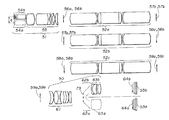•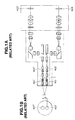•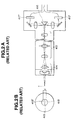•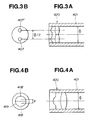•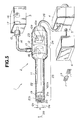•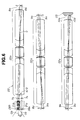•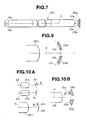•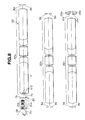•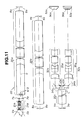•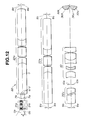•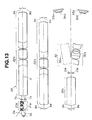•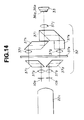•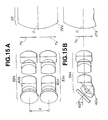•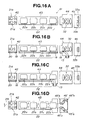•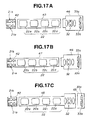••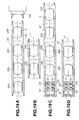•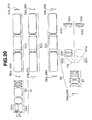•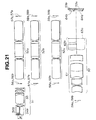•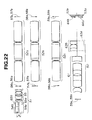•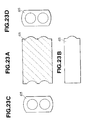•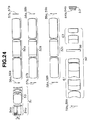•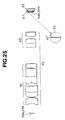•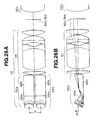•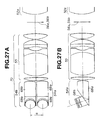••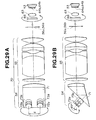•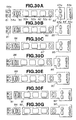•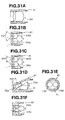•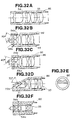•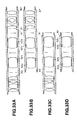•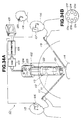•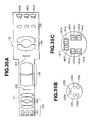•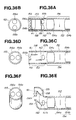•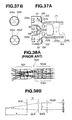•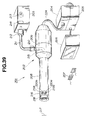•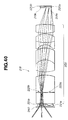•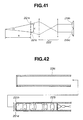•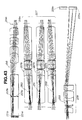•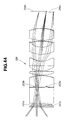•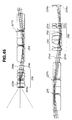•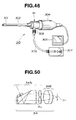•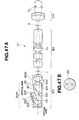•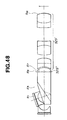•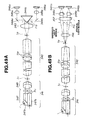•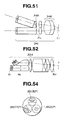••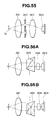••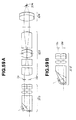••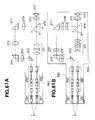## Classifications

• GPHYSICS
• G02OPTICS
• G02BOPTICAL ELEMENTS, SYSTEMS, OR APPARATUS
• G02B23/00Telescopes, e.g. binoculars; Periscopes; Instruments for viewing the inside of hollow bodies; Viewfinders; Optical aiming or sighting devices
• G02B23/24Instruments or systems for viewing the inside of hollow bodies, e.g. fibrescopes
• G02B23/2407Optical details
• G02B23/2415Stereoscopic endoscopes
• AHUMAN NECESSITIES
• A61MEDICAL OR VETERINARY SCIENCE; HYGIENE
• A61BDIAGNOSIS; SURGERY; IDENTIFICATION
• A61B1/00Instruments for performing medical examinations of the interior of cavities or tubes of the body by visual or photographical inspection, e.g. endoscopes; Illuminating arrangements therefor
• A61B1/00163Optical arrangements
• A61B1/00193Optical arrangements adapted for stereoscopic vision

## Abstract

The illuminating light transmitted by the light guide inserted through the elongate inserted section is projected out of the distal end surface of the inserted section. The illuminated objects pass through the respective pupils of the two objective lens systems arranged in parallel within the distal end section of the inserted section and their images are formed on the focal surface. The respective images are transmitted to the rear side by one common relay lens system. The transmitted final images are formed respectively on the image taking surfaces of the image taking devices. The respective images photoelectrically converted by the respective image taking devices are processed to be signals, are displayed in the monitor and are stereo-inspected through shutter spectacles.

## Description

This application is a divisional of Ser. No. 09/053,094 filed Apr. 1, 1998, now U.S. Pat. No. 6,306,082 which is a divisional of Ser. No. 08/404,890 filed Mar. 16, 1995 which has issued as U.S. Pat. No. 5,743,846.

BACKGROUND OF THE INVENTION Field of the Invention and Description of Related Arts

This invention relates to a stereoendoscope wherein images having passed through plural incident pupils are transmitted by common relay optical systems so that an observation providing a stereo-feel may be possible.

Recently, particularly in the surgical field, there is noted a so-called endoscope operation wherein, in order to reduce the burden on the patient, without opening the abdomen, a small hole is made in the abdominal part, an endoscope is inserted through the hole for the observation and treatment. In this field, the operation has been already made by directly seeing and stereo-observing the affected part with both eyes and therefore, even in the endoscope operation, the stereo-inspection is strongly desired. If the stereo-inspection can be made, the operation will be easy, the operation time will be reduced and the burden on the patient will be further reduced.

As a stereo-inspection endoscope whereby stereo-inspection is possible, there is a first related art example suggested in a Japanese patent application No.309078/1992 shown in FIG. 1A wherein two exactly the same optical systems are arranged in parallel and the images formed by the objective optical systems 401 and 401′ are transmitted for a predetermined distance by the transmitting optical systems 402 and 402′ (in this case, relay lens systems) and are taken by such image taking devices 403 and 403′ as CCD's.

The taken pair of the right and left images are converted to electric signals and are displayed in a TV monitor not illustrated. At this time, when the displayed right and left images are switched at a high speed and simultaneously shutter spectacles synchronized with the images are used, the image for the right eye will be observed with the right eye and the image for the left eye will be observed with the left eye so as to be able to be stereo-inspected.

Also, as another type stereoendoscope, there is a second related art example suggested in a Japanese patent application No.28278/1993 shown in FIG. 2A wherein the objective optical system 414 and the relay lens system 415 which is a transmitting optical system are formed of one axially symmetrical optical system. A prism 416 is arranged at the rear end of the relay lens system 415 and a pair of right and left images having a parallax are formed and taken in the the image taking devices 417 and 417′ by spatially dividing the pupil into two with the prism. FIGS. 1B and 2B on the left side of FIGS. 1A and 2A show respective incident pupils.

In order to make a stereo-inspection, it is necessary to obtain a pair of right and left images having a parallax from each other. Therefor, the incident pupil for the right image of the optical system and the incident pupil for the left image must be positioned as spatially separated. Also, the magnitude of the stereo-feel in the case of the stereo-inspection is proportional to the center distance between the right and left incident pupils.

In the two above mentioned related art examples, in the case of the first type in which the same two optical systems are arranged, when the objective optical systems 401 and 401′ to the image taking means 403 and 403′ are separately formed and the left and right incident pupils 407 and 407′ are separately positioned, images having a parallax from each other will be obtained. The center distance d between the left and right incident pupils 407 and 407′ coincides with the optical axis distance D between the left and right objective optical systems 407 and 407′.

In the second type in which the pupil is divided among the above mentioned related art, the objective optical system 414 and transmitting optical system 415 are formed of one axially symmetrical optical system and the pupil is one in this part but, when this one pupil is spatially divided into two by the pupil dividing means (in the above mentioned case, the pupil dividing prism) 416 and respective images are produced, images having a parallax from each other will be obtained. The center distance d between the left and right incident pupils 418 and 418′ is ½ the size of the incident pupil 419 of the objective lens.

In the type in which the same two optical systems are arranged, as it is formed of separate right and left parts, the number of the parts is high and the assemblability is low. Also, the magnification difference between the right and left images due to the errors of the respective parts is large, the displacement of the focusing position is large, the normal stereo-inspection can not be made and therefore a fine adjustment is necessary.

In the type of dividing the pupil, there are advantages that the parts common to the right and left light paths are many, the number of parts is low and the displacement of the right and left images can be made little. On the other hand, when compared with the same thickness, the magnitude of the parallax will be smaller than in the first type and a sufficient stereo-feel will be hard to obtain. That is to say, there is a problem that the center distance between the right and left incident pupils is hard to make large. This point shall be explained with reference to FIGS. 3A to 4B.

FIG. 3A shows as magnified the objective optical system on the distal end side of the first related art example. FIG. 3B shows its incident pupil. Also, FIG. 4A shows as magnified the objective optical system on the distal end side of the second related art example. FIG. 4B shows its incident pupil.

In the type in which the same two optical systems are arranged, that is, the first related art example, against the inside diameter Φ of the objective lens frame 421 of the endoscope distal end 420, the optical axis distance between the right and left objective optical systems is substantially Φ/2. Therefore, the center distance between the right and left incident pupils 407 and 407′ is also substantially Φ/2.

On the other hand, in the type in which the pupil is divided, against the inside diameter Φ of the objective lens frame 421 at the endoscope distal end 420, the diameter of the incident pupil 419 of the objective optical system is smaller than Φ, because the incident pupil of the objective optical system is smaller than the pupil of the relay lens system as the NA of the endoscope is limited by the outside diameter of the relay lens system and the picture angle of the objective optical system is larger than of the relay lens system.

Therefore, the center distance between the right and left incident pupils is smaller then Φ/2 and is usually about Φ/6 to Φ/10. Therefore, in this type, the magnitude of the parallax is about ⅓ that in the above mentioned type. Particularly, in case the distal end is thin, no sufficient stereo-feel will be obtained.

OBJECT AND SUMMARY OF THE INVENTION

In view of such circumstances, an object of this invention is to provide a stereoendoscope wherein, as in the type in which the pupil is divided, the parts common to the right and left light paths are made as many as possible, the variations of the right and left images by the production errors or the like can be made few and images having a stereo-feel by a parallax as large as of the type in which the same two optical systems are arranged are obtained.

The stereoendoscope of the present invention is characterized by comprising an objective optical system which has plural incident pupils formed in different positions and forms plural images having passed through these plural incident pupils and having a parallax from each other and a common image transmitting optical system which transmits the plural images having a parallax from each other.

When thus formed, as the objective optical system has plural independent incident pupils, irrespective of the size of the diameter of the incident pupil of the objective optical system, the parallax will be able to be made large. Also, as the plural images and pupils are not separately transmitted by the plural transmitting systems but are transmitted by the common image transmitting optical system, the number of parts will be able to be reduced. Even if a production error is present in the individual image transmitting optical system, as the images are transmitted by the common image transmitting system, the variation between the plural transmitted images will be able to be reduced.

In order to realize such formation, there are the following two systems (a) and (b):

• (a) A formation comprising an objective optical system which forms plural images having parallaxes in spatially separated positions and one image transmitting optical system which transmits the plural incident pupils and the plural images of the object optical system so that the images transmitted by this image transmitting optical system may be taken finally by one or more image taking means. More concretely, it is as in the following:

A stereoendoscope having an objective optical system, image transmitting optical system and image taking device, characterized in that the objective optical system comprises plural optical systems arranged in parallel and forms plural images having a parallax from each other and the image transmitting optical system comprises an optical system arranged along one optical axis and transmits plural images formed by the objective optical system.

In this formation, as the operation common to (a) and (b) is made and the image transmitted by the image transmitting optical system is also spatially separated, the image can be stereo-inspected through an image taking means taking images or an ocular optical system making observation with the naked eyes. The image taking means can use one or more image taking devices and can take plural images transmitted and spatially separated by the transmitting optical system and stereo-inspection is thereby possible.

The other formation realizing such formation is as follows:

• (b) A formation comprising an objective optical system forming plural images having parallaxes where they spatially substantially coincide (superimposed), jetting pupils corresponding to the plural incident pupils of the objective optical system and one image transmitting optical system transmitting the plural images, wherein the images transmitted by the image transmitting optical system are taken finally by one or more image taking means.

More concretely it is as follows:

A stereoendoscope having an objective optical system, image transmitting optical system and image taking device, characterized in that the objective optical system comprises plural front group optical systems arranged in parallel to take in plural images having a parallax from each other and rear group optical systems arranged so as to be on the same optical axis as of the image transmitting optical system and forming images of beams from the plural front group optical systems where the beams are substantially superimposed and the image transmitting optical system transmits the plural images formed by the rear group optical systems, having a parallax from each other and substantially superimposed and the jetting pupils of the objective optical system.

In this formation, as a common operation is made and images having parallaxes are transmitted to be formed where they are substantially superimposed, the diameter of the relay optical system can be made small.

BRIEF DESCRIPTION OF THE DRAWINGS

FIGS. 1A and 1B show a first related art example. FIG. 1A is a formation view showing its stereoendoscope. FIG. 1B is an explanatory view showing incident pupils.

FIGS. 2A and 2B show a second related art example. FIG. 2A is a formation view showing its stereoendoscope. FIG. 2B is an explanatory view showing an incident pupil.

FIG. 3A is a magnified sectioned view of an objective optical system part on the distal end side of the first related art example. FIG. 3B is an explanatory view showing incident pupils of FIG. 3A.

FIG. 4A is a magnified sectioned view of an objective optical system part on the distal end side of the second related art example. FIG. 4B is an explanatory view showing an incident pupil of FIG. 4A.

FIGS. 5 and 6 relate to the first embodiment of the present invention. FIG. 5 is a formation view showing the whole of a stereoendoscope apparatus provided with the first embodiment.

FIG. 6 is a formation view showing an image taking optical system in the stereoendoscope of the first embodiment of the present invention.

FIG. 7 is a formation view showing an image taking optical system in the second embodiment of the present invention.

FIG. 8 is a formation view showing an image taking optical system in the third embodiment of the present invention.

FIG. 9 is an explanatory view showing an arrangement example of an image taking device.

FIGS. 10A and 10B relate to a modification of the third embodiment. FIG. 10A is an explanatory view showing images formed by an objective optical system and relay optical system and a final image by the image transmission.

FIG. 10B is an explanatory view showing the arrangement of a final image and an image taking device arranged in the position in case the objective optical system and relay optical system of FIG. 10A are used.

FIG. 11 is a formation view showing an image taking optical system in the fourth embodiment of the present invention.

FIG. 12 is a formation view showing an image taking optical system in the fifth embodiment of the present invention.

FIG. 13 is a formation view showing an image taking optical system in the sixth embodiment of the present invention.

FIG. 14 is a formation view showing a main part of an image taking optical system in the seventh embodiment of the present invention.

FIGS. 15A and 15B relate to the eighth embodiment of the present invention. FIG. 15A is a plan view showing an objective optical system. FIG. 15B is a side view showing the objective optical system.

FIGS. 16A and 16B show the ninth embodiment of the present invention. FIG. 16A is a plan view showing a unit formation of the ninth embodiment.

FIG. 16B is a side view of FIG. 16A.

FIGS. 16C and 16D show a first modification of the ninth embodiment. FIG. 16C is a plan view showing a unit formation of the first modification.

FIG. 16D is a side view of FIG. 16C as an ocular adapter is connected.

FIGS. 17A to 17C are explanatory views respectively showing unit formations in the second to fourth modifications of the ninth embodiment.

FIGS. 18A to 18E are views respectively showing formations of objective optical system units.

FIGS. 19A to 19D are views respectively showing formations of relay optical system units.

FIG. 20 is a formation view showing an image taking optical system in the tenth embodiment of the present invention.

FIG. 21 is a formation view showing an image taking optical system in the eleventh embodiment of the present invention.

FIG. 22 is a formation view showing an image taking optical system in the twelfth embodiment of the present invention.

FIGS. 23A to 23D show a meniscus lens in a modification of the twelfth embodiment. FIG. 23A is a sectioned plan view. FIG. 23B is a side view as seen from the side of FIG. 23A. FIGS. 23C and 23D are respectively a front view and back view as seen respectively from the front surface and back surface sides.

FIG. 24 is a formation view showing an image taking optical system in the thirteenth embodiment of the present invention.

FIG. 25 is a formation view showing a main part of an image taking optical system in the fourteenth embodiment of the present invention.

FIGS. 26A and 26B show an objective optical system in the fifteenth embodiment of the present invention. FIG. 26A is a plan view. FIG. 26B is a side view.

FIGS. 27A and 27B show an objective optical system in a modification of the fifteenth embodiment. FIG. 27A is a plan view. FIG. 27B is a side view.

FIGS. 28A and 28B show an image taking optical system in the sixteenth embodiment. FIG. 28A is a plan view. FIG. 28B is a side view.

FIGS. 29A and 29B show an image taking optical system in the seventeenth embodiment. FIG. 29A is a plan view. FIG. 29B is a side view.

FIGS. 30A to 30G are explanatory views respectively showing unit formations of the eighteenth embodiment.

FIGS. 31A to 31F are views respectively showing concrete formations of front group units.

FIGS. 32A to 32F are views respectively showing concrete formations of objective optical system units.

FIGS. 33A to 33D are sectioned views respectively showing formations of rear group and relay lens system units.

FIGS. 34A and 34B relate to the nineteenth embodiment of the present invention. FIG. 34A is a general formation view of a stereoendoscope apparatus provided with the nineteenth embodiment.

FIG. 34B is a view of the arrangement of an objective optical system on the distal end surface of the stereoendoscope of the nineteenth embodiment.

FIGS. 35A to 35C relate to the twentieth embodiment of the present invention. FIG. 35A is a view showing the formation of a stereoendoscope of the twentieth embodiment.

FIG. 35B is an elevation showing the arrangement of an objective optical system as seen from the distal end surface.

FIG. 35C is an explanatory view showing the arrangement of an image taking device as seen from the distal end side.

FIGS. 36A to 36F show the formation on the distal end side of the twenty-first embodiment of the present invention. FIG. 36A is a vertically sectioned view. FIG. 36B is an elevation of FIG. 36A.

FIG. 36C is a horizontally sectioned view.

FIG. 36D is an elevation of FIG. 36B

FIG. 36E is a horizontally sectioned view.

FIG. 36F is an elevation of FIG. 36F.

FIGS. 37A and 37B show the formation on the distal end side of the twenty-second embodiment of the present invention. FIG. 37A is a vertically sectioned view. FIG. 37B is an elevation of FIG. 37A.

FIGS. 38A and 38B relate to a prior example. FIG. 38A is a formation view showing the formation of an objective optical system in a stereoendoscope of the prior example.

FIG. 38B is an explanatory view of a power arrangement for the objective optical system of FIG. 38A.

FIGS. 39 to 42 relate to the twenty-third embodiment of the present invention. FIG. 39 is a general formation view of a stereoendoscope apparatus provided with the twenty-third embodiment.

FIG. 40 is a formation view of an image taking optical system including the objective optical system in the stereoendoscope of the twenty-third embodiment.

FIG. 41 is an explanatory view of a power arrangement of the objective optical system of FIG. 40.

FIG. 42 is a sectioned view showing a frame structure at the distal end of the stereoendoscope in FIG. 39.

FIG. 43 is a formation view of the objective optical system and image transmitting optical system relating to the twenty-fourth embodiment of the present invention.

FIG. 44 is a formation view of the objective optical system in the twenty-fifth embodiment of the present invention.

FIG. 45 is a formation view of the image transmitting optical system including the objective optical system in FIG. 44.

FIGS. 46 to 48 relate to the twenty-sixth embodiment. FIG. 46 is a general formation view of an endoscope apparatus.

FIG. 47A is a formation view of a plural visual field direction type endoscope.

FIG. 47B is a view showing the formation of a brightness diaphragm.

FIG. 48 is a formation view of an objective optical system utilizing a pupil division.

FIGS. 49A to 54 relate to the twenty-seventh embodiment. FIG. 49A is a formation view of a plural visual field direction type endoscope including an objective optical system utilizing an eccentric optical system. FIG. 49B is a formation view of an endoscope relating to a modification of the twenty-seventh embodiment.

FIG. 50 is a formation view of an objective optical system in which an eccentric optical system is utilized and an afocal part is partly in common.

FIG. 51 is a formation view of an objective optical system in which an eccentric optical system is utilized and a perspective is made by refraction.

FIG. 52 is a formation view of a design of an objective optical system in which an eccentric optical system is utilized.

FIG. 53 is a formation view of a design in which a relay lens system is combined with an objective optical system.

FIG. 54 is an elevation of an objective optical system having three visual field directions.

FIGS. 55 to 58 relate to the twenty-eighth embodiment. FIG. 55 is a formation view of a plural visual field direction type endoscope having a pupil switching apparatus.

FIGS. 56A and 56B are formation views of a plural visual field direction type endoscope in which the visual field direction can be switched by an image rotator.

FIG. 57 is a formation view of a plural visual field direction type endoscope in which the visual field direction can be switched by the movement of a solid state image taking device or the like.

FIG. 58A is a formation view of a plural visual field direction type endoscope in which a pupil switching apparatus is provided near the pupil of an objective optical system.

FIG. 58B is a formation view of a plural visual field direction type endoscope different from that of FIG. 58A.

FIG. 59A is a formation view of a plural visual field direction type endoscope relating to the twenty-ninth embodiment. FIG. 59B is a formation view of an endoscope in which the objective optical system is made partly common.

FIG. 60A is a formation view of an optical system of a plural visual field direction type endoscope of the thirtieth embodiment.

FIG. 60B is a formation view of an optical system of a plural visual field direction type endoscope of a modification of the thirtieth embodiment.

FIG. 61A is a formation view of an optical system of a plural visual field direction type endoscope of the thirty-first embodiment.

FIG. 61B is a formation view of an optical system of a plural visual field direction type endoscope of a modification of the thirty-first embodiment.

DESCRIPTION OF THE PREFERRED EMBODIMENTS

The present invention shall be concretely explained in the following with reference to the drawings. The stereoendoscope in each of the first embodiment to the twenty-second embodiment is characterized by having an objective optical system which has plural incident pupils formed in different positions and forms plural images having passed through these plural incident pupils and having a parallax from each other and a common image transmitting optical system which transmits the plural images having a parallax from each other.

Each of the first embodiment to the modification of the ninth embodiment is of the formation (a). That is to say, images having a parallax from each other are formed in separated positions by plural objective optical systems arranged at the distal end of an endoscope and the images separated from each other are transmitted by one image transmitting optical system becoming common.

As shown in FIG. 5, a stereoendoscope apparatus 1 comprises a stereoendoscope 2 of the first embodiment having an image taking optical system for stereo-inspection built-in, a light source apparatus 3 feeding an illuminating light to an illuminating light transmitting means provided in this stereoendoscope to transmit the illuminating light, a camera controlling unit (abbreviated as a CCU hereinafter) 4 processing signals for an image taking means built-in in this stereoendoscope 2, a scan converter 5 converting the signal from this CCU 4 to a video signal, a color monitor 6 displaying the video signal put out of this scan converter 5 and shutter spectacles 27 having a shutter function for stereo-inspecting the image displayed in this color monitor 6.

The stereoendoscope 2 has an elongate inserted section 11 to be inserted into a body cavity or the like and a gripped section formed to be large in the diameter at the proximal end of this inserted section so as to be gripped by the operator. This inserted section 11 is formed of a cylindrical rigid jacket tube made of such metal as stainless steel. That is to say, this stereoendoscope 2 is a rigid endoscope having the rigid inserted section 11.

The same as an ordinary endoscope, this stereoendoscope has an illuminating light transmitting means transmitting the illuminating light fed from the light source apparatus 3, an illuminating optical system projecting this transmitted illuminating light out of an illuminating window and illuminating the object side and an observing optical system obtaining two images having a parallax so that the object illuminated by this illuminating optical system may be stereo-inspected.

By the way, in this specification, this observing optical system is mostly explained in an embodiment acting to form two images having a parallax on an image taking device provided with a photoelectrically converting function and is therefore also called an image taking optical system.

The gripped section 12 is provided with a light guide mouthpiece 13 and a light guide connector 15 at the other end of a light guide cable 14 removably connected at one end to this light guide mouthpiece 13 is removably connected to the light source apparatus 3.

A lamp 16 generating a white illuminating light and a lens 17 condensing this white light are arranged within the light source apparatus 3. The illuminating light condensed by this lens 17 is radiated on the end surface of the light guide connector 15, the illuminating light radiated on this end surface is transmitted by the light guide within the light guide cable 14 and the transmitted illuminating light is fed to the light guide 18 side within the stereoendoscope 2 from the light guide mouthpiece 13.

The light guide 18 as an illuminating light transmitting means is bent within the gripped section 12 and is inserted through the inserted section 11. This light guide 18 transmits the fed illuminating light and projects the illuminating light forward from the distal end surface fixed to the distal end 19 of the inserted section 11 and further through an illuminating lens 20 fitted to an illuminating window.

The respective optical images (represented by reference numerals 7 a and 7 b in FIG. 6) of the object (represented by the arrow in FIG. 5) 29 illuminated by this illuminating light are formed in image forming positions by objective optical systems 21 a and 21 b fitted to two observing windows arranged adjacently to the illuminating window within the distal end 19. The two objective optical systems 21 a and 21 b are of the same formation and are formed of optical lenses preferably of the same characteristics.

As shown in FIG. 5, the two objective optical systems 21 a and 21 b have the respective optical axes Oa and Ob in parallel with the center axis of the inserted section, are arranged in parallel on both sides of this center axis and are separated from each other by d in the distance (interval) between both optical axes Oa and Ob. Also, both optical axes Oa and Ob are arranged as separated in the diametral direction crossing the center axis and are therefore arranged symmetrically with the center axis. Two optical images large in the parallax can be formed by the objective optical systems 21 a and 21 b of the same formation with the optical axes arranged in parallel as separated by the distance d between them.

The images 7 a and 7 b are formed, as shown in FIG. 6, in separate positions by the two objective optical systems 21 a and 21 b and are transmitted rearward by a common relay optical system 22, that is, one image transmitting optical system or image transmitting means.

These images are equimultiply transmitted rearward by this relay optical system 22 and finally the same images 10 a and 10 b as the two images 7 a and 7 b by the two objective optical systems 21 a and 21 b are separately formed on a photoelectrically converting surface (image taking surface) of an image taking device 23 arranged within the gripped section 12. For example, in FIG. 5, if the separating direction in the two objective optical systems 21 a and 21 b is a horizontal direction, two images 10 a and 10 b will be separately formed in the horizontal direction on the image taking surface of the image taking device 23.

As shown in FIG. 5, the image taking device 23 has, for example, a square image taking surface and is arranged so that the vertical or horizontal direction of this image taking surface may coincide with the horizontal direction in which the two objective optical systems 21 a and 21 b are arranged as separated and the center of the image taking surface may be on the optical axis of the relay optical system.

By the way, the light guide 18 inserted through the inserted section 1 may be inserted through outside the relay optical system 22 (for example, like a ring). As shown in FIG. 5, a part of the vertical direction intersecting at right angles with the horizontal direction of the relay optical system 22 may be contained in an incised groove formed by incising in the axial direction a part of the vertical direction intersecting at right angles with the horizontal direction of the relay optical system 22. (One incised groove is shown in FIG. 5 but two incised grooves may be formed in the vertical direction.) When such incised groove is formed, the part which does not in principle substantially contribute to image transmission will be deleted, the image transmitting function will not be reduced, the illuminating light will be able to be transmitted and the inserted section 11 will be able to be made small in the diameter.

As the effective sectioned area of the relay optical system can be made large, the eccentricity (the distance d between the optical axes) of the two objective optical systems 21 a and 21 b arranged as opposed to each other eccentrically in the horizontal direction from the optical axis of this relay optical system 22 at the front end of this relay optical system 22, that is, the parallax will be able to be made large and the stereo-inspecting function will be able to be improved. Further, there is a function of reducing the superimposing (cross talk) of two images.

The gripped section can be fittably separated into the output section 24 in which the image taking device 23 is built-in and the input section 25 on its forward side. The input section 25 has an image taking optical system (observing optical system) comprising the two objective optical series 21 a and 21 b and relay optical system 22.

By making the output section 24 separable, there is made a flexible structure wherein the failing image taking device 23 can be easily repaired or can be replaced with one high in the sensitivity or the number of pixels to improve the performance and an ocular adapter can be connected to make stereo-inspection with the naked eyes. (The structure shown in the later described FIG. 19 may be adopted for the structure of the connecting part.)

The image taking device 23 is extended out of the rear end of the output section 24 and is connected with the CCU 4 through the signal cable 26 and the image taking signal photoelectrically converted by the image taking device 23 is processed. The image signal processed by this CCU 4 is further put into the scan converter 5, is converted to a video signal and is then put out to the color monitor 6. Two images corresponding to the optical images formed by the two objective optical systems 21 a and 21 b are alternately displayed in this color monitor 6. By observing the images of the color monitor 6 with shutter spectacles 27, the operator can stereo-inspect the images.

FIG. 6 shows the formations of the image taking optical systems, that is, the two objective optical systems 21 a and 21 b and relay optical system in the stereoendoscope 2 of the first embodiment.

The images 7 a and 7 b having a parallax from each other are formed by the plural (two in this embodiment) independent objective optical systems 21 a and 21 b arranged in the distal end section 19. These images 7 a and 7 b separated from each other are transmitted by the relay optical system 22 as one image transmitting optical system.

As shown in FIG. 6, the objective optical systems 21 a and 21 b, for example, the three relay lens systems 22 a, 22 b and 22 c forming the relay optical system 22 and the image taking device 23 having a function of photoelectrically converting optical images are arranged in the order mentioned from the object side. The two images 7 a and 7 b having a parallax are formed in the spatially separated positions (in this case, in the positions separated from each other in the horizontal direction) by the objective optical systems 21 a and 21 b of the same formation arranged in parallel as separated from each other by d (for example, d=4 mm) of the distance between their optical axes.

The images 7 a and 7 b are equimultiply relayed by the relay lens systems 22 a, 22 b and 22 c of the same formation arranged in series so that the optical axes may coincide with each other. That is to say, the images 7 a and 7 b formed on both left and right sides of the optical axis O of the relay optical system 22 (by the objective optical systems 21 a and 21 b arranged eccentrically on the left and right from this optical axis O) respectively form images 8 a and 8 b respectively on both right and left sides of this optical axis O in the rear side positions of the optical axis O by the relay lens system 22 a. These images 8 a and 8 b respectively form images 9 a and 9 b on both left and right sides of this optical axis O in the rear side positions of the optical axis O by the relay lens system 22 b. These images 9 a and 9 b respectively form images 10 a and 10 b on both right and left sides of this optical axis O in the rear side positions of the optical axis O by the relay lens system 22 c.

In this position, the image taking surface of the image taking device 23 is arranged and the images 10 a and 10 b are photoelectrically converted and put out. A masking means is provided so that the two images 10 a and 10 b on this image taking surface may not be superimposed. (As shown in the later described FIG. 8, for example, a visual field diaphragm 30 may be provided on the image forming surfaces of the objective optical systems 21 a and 21 b to limit the visual field. The invention is not limited to this. (The visual field diaphragm may be provided, for example, in the image forming position in the relay optical system 22.)

The optical axes O of the relay lens systems 22 a, 22 b and 22 c are respectively eccentric by the same amount on the right and left from the optical axes Oa and Ob of the objective optical systems 21 a and 21 b. The eccentricity can be selected in conformity with the desired parallax magnitude, that is, stereo-feel size and is d/2 (for example, d/2=2 mm) in this embodiment.

The number of relaying times is three times in this embodiment but can be set multiply from one time to ten and several times depending on such specification as the brightness of the optical system.

By the way, in FIG. 6, the reference numerals 28 a and 28 b respectively represent the positions of the incident pupils of the left and right objective optical systems 21 a and 21 b and the left and right images 7 a and 7 b are formed of the lights incident through the respective incident pupils 28 a and 28 b. The respective incident pupils 28 a and 28 b are transmitted by the relay lens systems 22 a, 22 b and 22 c forming the relay optical system 22.

During the transmission by the relay lens systems 22 a, 22 b and 22 c, the two pupils may be horizontally displaced but the relay lens systems 22 a, 22 b and 22 c had better be superimposed in order to be made small. Therefor, it is preferable that the two objective optical systems 21 a and 21 b are respectively formed to be telecentric optical systems, that is, the projecting pupils are formed to be infinitely far.

By the way, the magnitude of the parallax, that is, the center distance between the left and right incident pupils 28 a and 28 b is determined by the distance d between the optical axes Oa and Ob of the objective optical systems 21 a and 21 b and is independent of the brightness of the optical system.

According to this embodiment, as the relay optical system 22 is made common, the trouble of adjusting the lenses can be more extremely omitted than in the case that it is not made common (in the first related art) and a favorable stereo-observation can be made.

Also, as can be judged from FIG. 5, as an image having a parallax can be obtained by arranging the two objective optical systems 21 a and 21 b as separated from each other, the parallax can be made larger than in the case of using a common objective optical system (in the second related art) and therefore the function of obtaining a stereo-feel can be made large. (The same stereo-feel as in the case that two optical systems are arranged as in the first related art can be obtained.)

Therefore, according to this embodiment, the common optical components can be made few, the adjusted parts can be made few, the cost can be made low and the image having the same stereo-feel as in the case that two optical systems are arranged in the related art can be obtained.

As the two images 7 a and 7 b having a parallax are transmitted by the relay lens systems 22 a, 22 b and 22 c used in common with the axially symmetrical one, during the transmission, the qualities (the magnification, MTF, image position, chromatic aberration, coloring and the like) of the two images will lag little during the transmission.

That is to say, even if the individual characteristics of the relay lens system 22 a and the others are dispersed by the production error, in this embodiment, as the left and right images are transmitted by the common relay lens system 22 a and the others, the influence of the individual dispersion will not be substantially received. Therefore, the left and right images obtained by this embodiment will be images of a good quality having little lag.

In case an operation is made under the observation with this stereoendoscope, a good picture quality and a sufficient stereo-feel will be obtained, a picture image of an observation close to directly observing the affected part will be able to be realized and therefore an environment in which the operation is easy to make will be able to be provided.

Also, in this embodiment, as the left and right images 7 a and 7 b are formed in the positions spatially separated by the objective optical systems 21 a and 21 b and are formed in the positions spatially separated by the common relay optical system 22, therefore a stereo-inspection will be able to be made with the image taking device or the like without using an image separating means newly spatially separating the images.

Also, in this embodiment, the final images 10 a and 10 b by the relay lens system 22 c are taken by one image taking device 23. Therefore, the output section 24 is very simplified in the structure and a light weight stereoendoscope can be realized.

By the way, the image taking device 23 may be any of various solid state image taking devices (known generally by the names of CCD, PCD, CMD, AMI and SIT) and image taking tubes (known generally by the names of Sachicon, Busicon and HARP TUBE).

Also, the sensitivity may be improved by utilizing an image intensifier or the like.

The image taking device 23 may be a device for taking color images with a single plate or may take colored images with a formation as a 2-plate or 3-plate camera. Also, as shown in FIG. 6, the final images 10 a and 10 b by the relay lens system 22 c are taken by the common image taking device 23 to reduce the cost and weight.

In order that a stereo-feel optimum to the desire or operation type of the operator may be obtained, the distance between the respective optical axes of the two objective optical systems 21 a and 21 b may be made variable so that the magnitude of the parallax may be variable.

In this case, in order that the distal end section 19 may be made small, the two objective optical systems 21 a and 21 b may be made movable to the side opposite to each other in the horizontal direction vertical to the optical axis O of the relay lens systems 22 a, 22 b and 22 c. However, in this case, when the objective optical systems 21 a and 21 b move, the final images 10 a and 10 b will be also moved by the relay lens system 22 c and therefore, in case the image taking device 23 is fixed, the movement will be limited to be within the image taking range.

By the way, it has been explained that the image taking surface of the image taking device 23 is square. However, a rectangular surface long in the horizontal direction in which the objective optical systems 21 a and 21 b are arranged as separated may be used. In this case, the image taking range in which the image having a parallax is obtained will be able to be substantially expanded.

By the way, in FIG. 5 is adopted a simultaneous illuminating and image taking system wherein a color image is taken by using the image taking device 23 in which such color separating filter as a mosaic filter is arranged under a white color light illumination. However, the invention is not limited to this. A surface sequent image taking system wherein a color image is taken by obtaining such color component image as of three primary colors by taking an image with an image taking device having no color separating filter under a surface sequent illumination in which illuminating lights of such wavelength ranges as of red, green and blue are sequentially emitted on the object side will also do.

By the way, in the first embodiment, instead of connecting the output section to the input section 25, an ocular adapter 45′ shown in the later described FIG. 16D is fitted so that the stereo-inspection may be made with the naked eyes. In this case, it is preferable to set the number of relaying times by the relay optical system 22 at an even number of times so that the left and right images 7 a and 7 b by the objective optical systems 21 a and 21 b may be respectively observed with the left and right ocular lenses. (In FIG. 16D, the number of relaying times is four times.)

By the way, the lens data of the first embodiment are as in Table 1 shown at last in the specification. FIG. 2 and others are collectively shown after FIG. 1. In Tables 1 to 14, r1, r2, . . . , represent radii of curvatures of respective surfaces, d1, d2, . . . , represent surface distances, n1, n2, . . . , represent refractive indices of respective lenses and ν1, ν2, . . . , represent Abbe numbers of respective lenses.

In the following, the second to ninth embodiments are modifications of the first embodiment and, the same as in the first embodiment, the image having a parallax is formed in a position spatially separated by the objective optical systems 21 a and 21 b.

FIG. 7 shows a structure near the final images 10 a and 10 b of the relay lens system 22 c of the image taking optical system in the stereoendoscope of the second embodiment of the present invention. The final images 10 a and 10 b are respectively taken by the two image taking devices 23 a and 23 b. Signal lines (not illustrated) are connected respectively to the two image taking devices 23 a and 23 b and are connected to a CCU partly different in the internal formation from the CCU 4 in FIG. 5. The others are of the same formation as of the stereoendoscope 2 of the first embodiment.

By the way, in the CCU processing signals for the two image taking devices 23 a and 23 b, the same driving signal may be simultaneously applied, for example, to the two image taking devices 23 a and 23 b, may be simultaneously read out and may be memorized respectively in two frame memories. The same driving signal may be applied alternately respectively to the two image taking devices 23 a and 23 b and may be read out alternately and the image signal read out may be memorized alternately in the two frame memories.

The image signal simultaneously or alternately memorized in the two frame memories are alternately read out by the scan converter and are alternately displayed in the color monitor. The operator wears shutter spectacles 27 and can observe and stereo-inspect the image displayed in the color monitor 6.

The stereoendoscope apparatus provided with this second embodiment can be realized in substantially the same formation as of the stereoendoscope apparatus 1 in FIG. 5.

This second embodiment has an advantage that the image taking devices 23 a and 23 b can be focused respectively independently. If they are precisely adjusted, an image higher in the quality than in the case of a common image taking device 23 will be able to be made.

Also, the parallax can be made variable the same as in the first embodiment. However, this embodiment has an advantage that, when the left and right image taking devices 23 a and 23 b are moved as operatively connected with the movement of the objective optical systems 21 a and 21 b, the movement will not be restricted to be within the image taking range in the case of the common image taking device 23.

That is to say, in the first embodiment, as the image taking device 23 is common, the moving range of the left and right images 10 a and 10 b is restricted to be within the image taking range. However, according to the present embodiment, in case the final images 10 a and 10 b are fixed, when the movement deviates (separates) from the imaging range, the two image taking devices 23 a and 23 b will be moved horizontally as operatively connected with the movement of the objective optical systems 21 a and 21 b and the final images 10 a and 10 b will be able to be maintained within the image taking range of the respective image taking devices 23 a and 23 b.

Therefore, there is a merit that a stereo-endoscope in which an image having a stereo-feel is obtained can be realized. The others have the same effects as in the first embodiment. By the way, the lens data of the second embodiment are the same as of the first embodiment.

FIGS. 8 and 9 relate to the third embodiment of the present invention. FIG. 8 shows an image taking optical system in the third embodiment. FIG. 9 shows as magnified the arrangement of the image taking devices 23 a and 23 b. In this embodiment, the two image taking devices 23 a and 23 b are used the same as in the second embodiment and the light receiving surfaces of the image taking devices 23 a and 23 b arranged not vertically to the optical axis O of the relay optical system 22 but as inclined from the vertical direction. In other words, in the central part of the light receiving surface of each of the image taking devices 23 a and 23 b, the optical axis vertical to this light receiving surface is arranged not to be parallel with the optical axis O of the relay optical system 22 but to make an angle larger than O.

That is to say, when the light receiving surface of each of the two image taking devices 23 a and 23 b is arranged as inclined in conformity with the image surface curvature aberration 10 c generated by the relay lens systems 22 a, 22 b and 22 c and shown in FIG. 2, the deterioration of the image by the curvature aberration will be controlled or reduced.

As the petzval of the relay lens systems 22 a, 22 b and 22 c is positive, even if the image surface by the objective optical systems 21 a and 21 b is flat, in the case of the transmission by the relay lens systems 22 a, 22 b and 22 c, the image surface will bend on the curved surface with the concave surface directed to the objective side.

Therefore, with the image taking surface or light receiving surface left to be arranged vertically to the optical axis of the relay lens systems 22 a, 22 b and 22 c, a partial fog will be likely to be produced and it will be difficult to keep all the Image taking surface focused.

Therefore, in the third embodiment, as shown in FIG. 9, the light receiving surface is arranged as inclined in conformity with the contact surface of the curved image surface. In FIG. 9, the light receiving surface is inclined by 25.332 degrees to the surface vertical to the optical axis of the relay lens system 22 c.

According to this third embodiment, not only the effects of the second embodiment are retained but also a picture image having little curvature aberration is obtained. By the way, the lens data of the third embodiment are as in Table 2.

By the way, as the petzval sum of the relay lens systems 22 a, 22 b and 22 c is a positive value, the petzval sum of the objective optical systems 21 a and 21 b may be made a negative value to control the image surface curvature aberration of the final images 10 a and 10 b having passed through the relay lens system 22 c.

FIGS. 10A and 10B are of modifications showing this manner.

As shown in FIG. 10A, the petzval sum of the objective optical systems 21 a and 21 b is made a negative value to form images 7 a and 7 b becoming concave on the rear side (the local radius of curvature of each image surface shall be represented by R). In case the image on the flat image surface is transmitted by the relay lens systems 22 a, 22 b and 22 c, the local radius of curvature of the image surface of the final images 23 a and 23 b is represented by R′ and, as shown in FIG. 10, the light receiving surfaces of the image taking devices 23 a and 23 b are arranged on the contact surface of the curved surface of a local curvature 1/R″=1/R−1/R″, the influence of the image surface curvature aberration will be further controlled by this embodiment than by the third embodiment.

By the way, in this case, 1/R−1/R″=0 or the absolute value of 1/R−1/R″ may be made small.

FIG. 11 shows an image taking optical system in the fourth embodiment. The final images 10 a and 10 b of the relay lens system are relayed once more by adapter lens systems 32 a and 32 b forming an adapter optical system to connect images 36 a and 36 b and these images 36 a and 36 b are taken respectively by image taking devices 33 a and 33 b.

The adapter lens systems 32 a and 32 b are formed respectively of mirror parts 34 a and 34 b and lens parts 35 a and 35 b, a beam is parallel moved outside by the mirror parts 34 a and 34 b (in this embodiment, the displacement L is 6 mm) and the lens parts 35 a and 35 b act to re-form the final images 10 a and 10 b of the relay lens system at any magnification.

The optical axis of each of the lens parts 35 a and 35 b is eccentric by d/2(2 mm) from the optical axis of the relay lens system 22 c except the parallel moved part by the mirror parts 34 a and 34 b.

In this embodiment, when the parallel moving distance in the mirror sections 34 a and 34 b and the magnification in the lens sections 35 a and 35 b are properly set, images 36 a and 36 b optimum to any size image taking devices 33 a and 33 b will be able to be obtained.

Also, as the image taking devices 33 a and 33 b larger in the size than in the first and second embodiments can be used, those larger in the number of pixels in response to the size can be used and a favorable stereo-observed image high in the resolving degree can be obtained. The others have the same effects as in the second embodiment. The lens data of this embodiment are as in Table 3.

FIG. 12 shows an image taking optical system in the fifth embodiment. This embodiment is an improvement of the fourth embodiment.

The images 36 a and 36 b are respectively formed by relaying further once the final images 10 a and 10 b of the relay lens system with the common adapter optical system 32 formed of one lens system and are taken by the image taking devices 33 a and 33 b. The adapter optical system 32 is formed of a lens system arranged so as to be of the same optical axis as of the relay lens systems 22 a, 22 b and 22 c, the final images 10 a and 10 b of the relay lens system are formed again at any magnification and the image taking devices 33 a and 33 b are arranged in the image forming positions.

In this embodiment, the formation can be made simpler by the part having no mirror section within the adapter optical system 32 and has the operations and effects of the fourth embodiment. That is to say, when the magnification of the adapter optical system 32 is optionally set, the images 36 a and 36 b optimum to the image taking devices 33 a and 33 b of any size will be able to be obtained.

Also, in this embodiment, the same as in the third embodiment, the light receiving surface of each of the image taking devices 33 a and 33 b is inclined in conformity with the image surface curvature aberration generated by the relay lens systems 22 a, 22 b and 22 c and adapter optical system 32 to control the deterioration of the image. In FIG. 12, the light receiving surface is arranged as inclined by 11.902 degrees to the surface vertical to the optical axis of the relay lens system 22 c. The lens data of this embodiment are as in Table 4.

FIG. 13 shows an image taking optical system in the sixth embodiment.

The final images 10 a and 10 b of the relay lens system are further once relayed by the adapter lens systems 32 a and 32 b forming the adapter optical system 32 and are taken by the image taking devices 33 a and 33 b. The adapter optical system 32 is formed of the two inclined adapter lens systems 32 a and 32 b of the same formation. One lens system 32 b and the image taking device 33 b are parallel eccentric by d/2(=2 mm) from the optical axis of the relay lens system 22 c and are then inclined by 10.076 degrees with the point at which the optical axis of the lens system 32 b intersects with the final image 10 b of the relay lens system 22 c as a center. The lens system 32 a illustrated by the two-point chain lines is also arranged as inclined the same on the opposite side of the optical axis of the relay lens system 22 c.

In this embodiment, too, the same as in the fifth embodiment, no mirror section is present and, by freely setting the magnification of the adapter optical system, the images 36 a and 36 b optimum to the image taking device of any size can be obtained. That is to say, this embodiment has substantially the same effects as of the fifth embodiment. The lens data of this embodiment are as in Table 5.

FIG. 14 shows a main part of an image taking optical system in the seventh embodiment of the present invention.

The final images 10 a and 10 b of the relay lens system are further once relayed by the adapter optical system 32 and are formed in the same positions and the common image taking device 33 is arranged in the image forming position in the formation.

In the adapter optical system 32, the final images 10 a and 10 b of the relay lens system are led to the shutter means 37 e side through an optical axis distance extending means comprising respectively lenses 37 a and 37 b and prisms 37 c and 37 d and are led to the opposed lens 37 f and 37 g side so that, when one is shielding light, the other will be passing light. A beam having passed through the lens 37 f arranged as opposed to one side of the shutter means 37 e passes through the prism 37 h, half prism 37 i and lens 37 j and forms an image 36 a in the position in which the image taking device 33 is arranged.

Also, a beam having passed through the lens 37 g arranged as opposed to the other side of the shutter means 27 e passes through the optical device 37 k, half prism 37 i and lens 37 j and forms an image 36 b in the position in which the image taking device 33 is arranged.

In this embodiment, the relayed images 36 a and 36 b are formed in the same position and are taken by one image taking device 33. The shutter means 37 e is arranged on the way of the adapter optical system 32 and alternately shields the beam so that two images may not be simultaneously formed by the image taking device 33.

This embodiment has an advantage that one image taking device 33 will do and the cost can be reduced. The others have the same effects as of the fourth embodiment.

FIGS. 15A and 15B show a formation of an objective optical system in the eighth embodiment of the present invention.

In this embodiment, an objective optical system is formed of perspective objective optical systems 39 a and 39 b having a perspective front as a visual field.

In this embodiment, a beam incident from the diagonal front side is reflected by using reflecting prisms 40 a and 40 b as visual field direction changing means and is changed to be in a direction parallel to the optical axis O of the relay optical system 22 (FIGS. 15A and 15B show only a part of the relay lens system 22 a). In this embodiment, the visual field direction is 45 degrees with the lengthwise direction (the optical axis direction of the relay optical system 22) of the inserted section. The reflecting prisms 40 a and 40 b may be two separate bodies or one integral body.

The rear side formation of the relay optical system 22 may be the formation of any of the first to sixth embodiments. This embodiment has the same effects as of the first to seventh embodiments except that the visual field direction is different.

Otherwise than the eighth embodiment, the visual field direction can be varied by varying the angles of the reflecting prisms 40 a and 40 b. Also, if the objective optical system parts are replaceably formed, various visual field directions, visual field angles and parallaxes will be able to be obtained by replacing only the objective optical system.

FIGS. 16A to 16D show the ninth embodiment of the present invention and a unit formation in its first modification.

The stereoendoscope 41 of the ninth embodiment shown in FIG. 16A comprises an objective optical system unit 42, relay optical system unit 43, adapter optical system unit 44 and image taking device unit 45.

The objective optical system unit 42 has objective optical systems 21 a and 21 b of uniform optical characteristics built-in. The relay optical system unit 43 has relay lens systems 22 a, 22 b, 22 c and 22 d of the same formation built-in. The adapter optical system unit 45 has a common adapter optical system 32 built-in. The image taking device unit 45 has image taking devices 33 a and 33 b of uniform characteristics built-in.

FIG. 16A as seen from the side is as in FIG. 16B. The objective optical system unit 42 has a distal end side section of a light guide 18 and an illuminating lens 20 built-in. The relay optical system unit 43 has an intermediate section of the light guide 18 built-in. The adapter optical system unit 44 has a rear end side section of the light guide 18 built-in. A light guide mouthpiece 13 is provided.

Also, in this embodiment, the relay lens systems 22 a, 22 b, 22 c and 22 d within the relay optical unit 43, for example, (for example, are cut off in the lengthwise direction on the lower side to be in the direction vertical to the horizontal direction in which the objective optical systems 21 a and 21 b are arranged to secure a space to contain the light guide 18 and) make the inserted section small in the diameter. Also, the adapter optical system 32 within the adapter optical system unit 44 is cut off on the light guide mouthpiece 13 side.

In this embodiment, the objective optical system unit 42 is connected to the distal end of the relay optical system unit 43, the distal end of the adapter optical system unit 44 is connected to the proximal end of the relay optical system unit 43 and the image taking device unit 45 is connected to the proximal end of this adapter optical system unit 44 to form a stereoendoscope 41.

Therefore, by combining the respective units different in the optical characteristics and image taking characteristic, stereoendoscopes of different characteristics can be simply realized. Therefore, the stereoendoscopes 41 of different characteristics can be provided so as to be selected by the users for their using objects.

In this embodiment, the connecting part of the proximal end of the relay optical system unit 43 and the distal end of the adapter optical system unit 44 corresponds to the border of the input section 25 and output section 24 shown in FIG. 5.

By the way, in FIGS. 16A and 16B, the part after the adapter optical system unit 44 is made large in the diameter. However, as shown in FIG. 16C, the proximal end side of the relay optical system unit 43 may be made large in the diameter on the proximal end side, the proximal end side part of the light guide 18 may be built-in near this proximal end and the light guide mouth piece 13 may be provided there in the structure.

In this first modification, the light guide 18 need not be built-in in the adapter optical unit 44 and therefore the structure will be simple.

In this modification, the image taking device unit 45 may be fitted directly to the relay optical system unit 43 without using the adapter optical system unit 44 in the structure. In such case, the formation of the second embodiment will be made. Further, in case one common image taking device is built-in as the image taking device unit 45, the formation of the first embodiment will be made.

This first modification is higher in the freedom of combination than the ninth embodiment and can simply realize stereoendoscopes 41 different in the characteristics. Also, as shown in FIG. 16D, when an ocular adapter 45′ is connected to the proximal end of the relay optical system unit 43, a stereoendoscope by which stereo-inspection can be made with the naked eyes will be able to be formed.

The ocular adapter 45′ shown in FIG. 16D is of a structure whereby the final images by the relay optical system unit 43 can be magnified and observed respectively through prisms and ocular lenses 45a and 45b fitted to an ocular window corresponding to the distance between both eyes of the operator so that the left and right images by the objective optical systems 21 a and 21 b may be respectively stereo-inspected through the left and right ocular lenses 45a and 45b.

By the way, in this case, as the final images are inverted images, the ocular adapter 45′ is provided with lenses 45a and 45b as means for making them upright to form upright images in front of the ocular lenses 45a and 45b. Instead of providing the lenses 45a and 45b, the two prisms for extending the distance between the optical axes may be made such prisms for inverting images as Porro prisms.

The ocular adapter for observation with the naked eyes may be connectable to the relay optical system unit 43 in FIG. 16A in the structure or may be connectable to the second to fourth modifications of the ninth embodiment shown in FIGS. 17A to 17C explained in the following.

In the second modification shown in FIG. 17A, in FIG. 16A, the adapter optical system 32 and the image taking devices 33 a and 33 b are formed of an adapter optical system image taking unit 46 as one unit.

In the third modification shown in FIG. 17B, in FIG. 17A, further, the objective optical systems 21 a and 21 b and the relay optical system 22 are formed of an objective optical system relay optical system unit 47 as one unit. In the fourth modification shown in FIG. 17C, in FIG. 16A, the relay optical systems 22 and the adapter optical system 32 are formed of a relay optical system adapter optical system unit 48 as one unit.

FIGS. 18A to 18E show more concrete formations of various units used in the ninth embodiment and its modifications.

FIG. 18A shows an objective optical system unit 42 of a visual field angle of 70 degrees. FIG. 18B shows an objective optical system unit 42 of a visual field angle of 40 degrees. When they are replaced and are connected to a relay optical system unit 43, any desired visual field angle will be obtained.

A male screw is formed at the proximal end of the jacket tube of the objective optical system unit 42 and can be removably connected by being screwed to a female screw at the distal end of the jacket tube of the relay optical system unit 43. A projection is provided at the proximal end of the jacket tube of the objective optical system unit 43 and can be contacted with a level difference surface made by cutting off the inner peripheral surface on the distal end side of the jacket tube of the relay optical system unit 43 to determine the position in the lengthwise direction. By the way, both jacket tubes are of the same outside diameter so that, in case they are connected with each other, no level difference will be made on the inserted section.

Also, a positioning mark and screw hole are provided as peripheral positioning means near the proximal end of the jacket tube of the objective optical system unit 42. When this mark is made to meet a positioning mark at the distal end of the jacket tube of the relay optical system unit 43, both screw holes will be able to be set to communicate with each other and will be able to be fixed with a screw not illustrated.

By the way, the same connecting means or connecting mechanism as on the proximal end side of the jacket tube of the objective optical system unit 42 is provided on the proximal end side of the jacket tube of the relay optical system unit 43 and can be removably connected to the distal end of the jacket tube of the adapter optical system unit 44.

FIG. 18C shows an objective optical system unit 42 perspective in the visual field direction of 45 degrees. In FIG. 18C, by replacing the reflecting prism 40, the objective optical system unit 42 perspective in any visual field direction can be formed. By the way, FIG. 18D shows FIG. 18C as seen from the rear end side and a pair of objective optical systems 39 a and 39 b arranged on the left and right.

FIG. 18E shows an objective optical system 42 in which the parallax is reduced and the optical axes of two objective optical systems 21 a and 21 b are near to each other and the distance d′ between the optical axes is d′<d. In the case of this formation, the function of obtaining a stereo-feel will reduce but, as the objective optical systems are arranged on the center axis side, a space for inserting them through other internal organs or the like will be able to be secured, therefore, for example, the cross-sectioned area of the light guide will be able to be made large, the illuminating light amount will be able to be increased and a bright image will be obtained.

By the way, when the image taking device unit or the adapter optical system unit is replaced, as required, in response to the optical axis distance and visual field angle of the objective optical systems 21 a and 21 b, an optimum stereoendoscope conforming to the equivalent will be able to be provided.

FIG. 19A shows a formation of a relay optical system unit 43. The proximal end of this relay optical system 43 can be removably connected to the distal end of the adapter optical system unit 44. Also, the proximal end of this adapter optical system unit 44 can be removably connected to the image taking unit 45.

As shown, for example, in FIG. 19B, the relay optical system unit may be the relay optical system unit 43 in which the number of relaying times is made twice. Further, a relay optical system unit in which the number of relaying times is different depending on the inserted length inserted into the body cavity or the like can be also used.

FIG. 19C shows a formation of an objective optical system relay optical system unit 47 integrating an objective optical system and a relay optical system. FIG. 19D is a modification of FIG. 19C and shows a unit in which the number of relaying times of the relay optical system is made twice. Various numbers of relaying times of the relay optical system can be prepared. A different length of the inserted section can be selected as required.

In the following, the tenth to eighteenth embodiments are embodiments of the formation (b) in the above mentioned paragraph of the summary. Images having a parallax with each other are taken into the plural front group optical systems of the objective optical systems arranged in the distal end section of the endoscope and plural images in one rear group optical system are formed in substantially coinciding positions. These substantially superimposed images are transmitted by a common rear group optical system and a common image transmitting system coinciding with this rear group optical system in the optical axis.

FIG. 20 shows an image taking optical system in the tenth embodiment.

An objective optical system 51 in which the object side opening part is separated into two parts, relay lens systems 52 a, 52 b and 52 c, an adapter optical system 50 and image taking devices 53 a and 53 b are arranged in the order mentioned from the object side. The objective optical system 51 is formed of front group optical systems (abbreviated merely as front groups) 54 a and 54 b of the same formation which are parallel arranged as separated by the distance d(=4 mm) between the optical axes of each other and a rear group optical system (abbreviated merely as a rear group) 55 arranged to be of one same optical axis. Two images 56 a and 56 b having a parallax are formed in spatially substantially coinciding positions.

The images 56 a and 56 b form a relay optical system and are equimultiply relayed by (for example, three) relay lens systems 52 a, 52 b and 52 c of the same formation arranged in series so as to be of the same optical axis with each other.

That is to say, by the relay lens system 52 a, the images 56 a and 56 b form images 57 a and 57 b with equal sizes in substantially the same positions in the rear of this relay lens system 52 a. By the relay lens system 52 b, these images 57 a and 57 b form images 58 a and 58 b with equal sizes in substantially the same positions in the rear of this relay lens system 52 b. By the relay lens system 52 c, these images 58 a and 58 b form images 59 a and 59 b with equal sizes in substantially the same positions in the rear of this relay lens system 52 c.

The rear group 55 of the objective optical system 51 and the optical axis of the relay lens systems 52 a, 52 b and 52 c are on the same axis. This optical axis and the optical axis of the front groups 54 a and 54 b are eccentric respectively on the left and right.

The eccentricity can be selected in conformity with a desired size, that is, the size of the stereo-feel and is respectively d/2(=2 mm) in this embodiment. An afocal beam may not be between the front groups 54 a and 54 b and the rear group 55. However, for making small, this part may be of an afocal beam. An image formed by the objective optical system had better be substantially superimposed.

For the picture angle of the ordinary relay system, the picture angle required by the endoscope is large. From the condition that, as described above, the front groups 54 a and 54 b had better be nearly afocal and from the condition that non-common parts had better be few, the front groups 54 a and 54 b had better be formed of two groups of a concave group and convex group from the object side.

When the plural images having a parallax and transmitted by the relay optical system are substantially superimposed, the relay optical system will be able to be made small in the diameter. Therefore, the projected pupil of the objective optical system 51 may be made substantially infinite. Therefore, the front side focal position of the rear group 55 of the objective optical system 51 will be the pupil position. In order that the beam entering the front groups 54 a and 54 b from the object may be well transmitted to the rear group 55, it is preferable to coincide with the projected pupil of the front groups 54 a and 54 b. Concretely, it is preferable that the final surfaces of the front groups 54 a and 54 b are arranged on the image side rather than in the front side focal position of the rear group 55.

In this embodiment, the number of relaying times is three times but can be selected and set multiply to be usually one time to ten and several times depending on such specifications as the length and diameter of the inserted section of the endoscope and the brightness and the like of the optical system.

The magnitude of the parallax, that is, the center distance between the right and left incident pupils is determined by the optical axis distance d between the front groups 54 a and 54 b of the objective optical system 51 and is independent of the brightness of the optical system.

According to this embodiment, the same as in the first embodiment, the two images 56 a and 56 b having a parallax are transmitted by one axially symmetrical relay optical system and therefore an error will be little generated in the qualities (the magnification, MTF, image position, chromatic aberration, coloring and the like) of the two images being transmitted.

Non-common parts are less on the right and left of the objective optical system 51 than in the first embodiment. Therefore, the trouble of adjusting the lenses can be extremely omitted and a favorable stereo-observed image can be obtained.

Further, in this embodiment, as spatially substantially superimposed images are transmitted by the relay optical system, when each of the front groups 54 a and 54 b is formed of an elliptic lens system in which, for example, the horizontal direction is a short axis and the vertical direction is a long axis and the pupil is also made elliptic, the objective optical system and relay optical system will be able to be made small in the diameter without deteriorating the parallax, brightness and the like. In this case, the inserted section will be able to be made small in the diameter from the distal end to the proximal end side and the applied range in which the endoscope can be inserted and used will be able to be expanded. As the hole through which the inserted section is inserted into the abdominal part may be small, the pain given to the patient will be able to be reduced. By the way, even in the other embodiments, the objective optical system may be formed of an elliptic lens system.

In this embodiment, the final images 59 a and 59 b of the relay lens system 52 c are in substantially the same position and therefore must be separated from each other by any means which is a pupil dividing image forming means.

Therefor are required a means for forming an image of a pupil transmitted by the relay optical system and a means for forming an image of a partial beam of this pupil and forming an image by spatially separating plural images having a parallax. Concretely, performing it is the adapter optical system 50 formed of the pupil image forming lens system 61, mirror parts 62 a and 62 b and image forming lens systems 63 a and 63 b arranged to be on the same optical axis as of the relay lens system 52 c.

The pupil image forming lens system 61 forms in spatially separated positions images of two pupils of the objective optical system 51 transmitted by the relay lens systems 52 a, 52 b and 52 c. In the mirror parts 62 a and 62 b, the beams of the two pupils are parallel moved outside (in this embodiment, the movement is 6 mm) and the image forming lens systems 63 a and 63 b have an action of forming images 64 a and 64 b respectively in the image taking devices 53 a and 53 b.

The optical axes of the image forming lens systems 63 a and 63 b are eccentric by d/2(=2 mm) from the optical axis of the relay lens system 52 c except the parallel moved part by the mirror parts 62 a and 62 b. By the way, the mirror parts 62 a and 62 b and image forming lens systems 63 a and 63 b are illustrated respectively only on one side.

Lest the right and left pupils should be finally superimposed, a brightness diaphragm 79 may be provided on the pupil surface (in this embodiment, on the projecting pupil surface of the pupil image forming lens) in any of the pupil position and its conjugate position to limit the beam.

In this embodiment, when the parallel moving distance in the mirror parts 62 a and 62 b and the magnification of the adapter optical system 50 are properly set, the images 64 a and 64 b optimum to the image taking devices 53 a and 53 b of any size will be able to be obtained.

As in FIG. 20, the parallel moving direction of the mirror parts 62 a and 62 b may be any direction within or vertical to the paper surface. When the focal distances of the image forming lens systems 63 a and 63 b are varied, the magnification will be able to be also varied.

In order to obtain the stereo-feel optimum to the operator's desire or a system, the optical axis distances between each other of the two front groups 54 a and 54 b may be made variable so that the magnitude of the parallax may be variable. In this case, in order to make the distal end section small, the two front groups 54 a and 54 b may be made movable in the directions reverse to each other vertically to the optical axis of the relay optical system.

However, in this case, as the projected pupil of the objective optical system is moved by the movement of the front groups 54 a and 54 b, it will be necessary to make the effective diameter of each lens rather large so that the beam may not be intercepted by the optical systems following the relay lens systems 52 a, 52 b and 52 c.

The others have the same operations and effects as of the first embodiment. The lens data of this embodiment are as in Table 6.

The following eleventh to seventeenth embodiments are of the formations made by modifying the tenth embodiment. The images having a parallax between each other are formed in spatially substantially coinciding positions. All these objective optical systems 51 can be formed to be interchangeable with the objective lenses of the pupil dividing type stereoendoscope of the related art.

FIG. 21 shows an image taking optical system in the eleventh embodiment of the present invention. Images 64 a and 64 b are formed by further once relaying the final images 59 a and 59 b of the relay lens system with the adapter optical system 50 and are taken by the image taking devices 53 a and 53 b.

The adapter optical system 50 is formed of a pupil image forming lens system 61 and image forming lens systems 63 a and 63 b arranged to be of the same optical axis as of the relay lens system 52 c. The optical axes of the image forming lens systems 63 a and 63 b are eccentric by 1.25d (=5 mm) from the optical axis of the relay lens system 22 c.

By the way, the image forming lens system is illustrated only on one side. This embodiment is simpler by the part having no mirror part within the adapter optical system 50 than the tenth embodiment. The same as in the tenth embodiment, when the magnification of the adapter optical system 50 is freely set, the images 64 a and 64 b optimum to any image taking device will be able to be obtained. The distance between the two pupils divided by the pupil image forming lens system 61 can be varied by adjusting the focal distance of this pupil image forming lens system 61. The others have the same operations and effects as of the tenth embodiment.

The lens data of this embodiment are as in Table 7.

FIG. 22 shows an image taking optical system in the twelfth embodiment of the present invention. The front groups 54 a and 54 b of the objective optical system 51 are formed of meniscus lenses 65 a and 65 b having a concave surface on the object side. In this embodiment, as the non-common part of the right and left light paths is further less than in the eleventh embodiment, the error will be less between the qualities of the two images.

The lens data of this embodiment are as in Table 8.

Further, when the meniscus lenses 65 a and 65 b are made an integrally molded lens 65 as shown in FIGS. 23A to 23D, the error on the right and left from the objective optical system 51 to the pupil image forming lens system 61 will be able to be reduced to a level where the error will not be a practical problem and the trouble of adjusting the lenses will be well eliminated. The others have the same operations and effects as of the eleventh embodiment.

By the way, of FIGS. 23A to 23D, FIG. 23A is a sectioned plan view, FIG. 23B is a side view of FIG. 23A as seen in the side direction and FIGS. 23C and 23D are a front view and back view respectively as seem from the front side and back side.

FIG. 24 shows an image taking optical system in the thirteenth embodiment of the present invention. The final images 59 a and 59 b of the relay lens system are further once relayed by the adapter optical system 50. As the adapter optical system 50 is of the same optical axis as of the relay lens system 52 c, the relayed images 64 a and 64 b will be formed in substantially the same positions and will be taken by one common image taking device 53.

A shutter 66 is arranged between the pupil image forming lens system 61 and image forming lens system 63 and alternately intercept the beam so that two images may not be simultaneously formed in the image taking device 53.

This embodiment has an advantage that one image taking device 53 will do. The others have the same effects as of the twelfth embodiment. The lens data of this embodiment are as in Table 9.

FIG. 25 shows a main part of an image taking optical system in the fourteenth embodiment of the present invention. The same as in the thirteenth embodiment, as the adapter optical system 50 is of the same optical axis as of the relay lens system 52 c, the relayed images 64 a and 64 b will be formed in substantially the same positions and will be taken by one image taking device 53.

A lenticular lens 67 is arranged just before the light receiving surface of this image taking device 53 which is to be used in common. When the right and left images are formed at intervals of one row or one line by the image taking device 53, the two images will be taken as separated. This embodiment has also an advantage that one image taking device 53 will do. The others have the same effects as of the thirteenth embodiment. By the way, the lens data of this embodiment are the same as of the thirteenth embodiment.

FIGS. 26A and 26B show an objective optical system in the fifteenth embodiment of the present invention. In this embodiment, the visual field direction is 30 degrees with the lengthwise direction of the endoscope (the optical axis direction of the relay lens). The reflecting prisms 68 a, 68 b and 69 a, 69 b forming the front groups 54 a and 54 b may be respectively two separate bodies or one integral body.

FIG. 27 shows an objective optical system in a modification of the fifteenth embodiment of the present invention. The same as in the fifteenth embodiment, a perspective objective optical system 70 is formed. In this modification, the visual field direction is 70 degrees with the lengthwise direction of the endoscope (the optical axis direction of the relay lens). The reflecting prisms 68 a, 68 b and 69 a, 69 b may be respectively two separate bodies or one integral body.

In the fifteenth embodiment and its modification, the visual field direction can be varied by varying the angles of the reflecting prisms 68 a, 68 b and 69 a, 69 b. Therefore, when the front group part is formed to be interchangeable, various visual field directions or visual field angles will be able to be obtained by interchanging only this front group. Needless to say, even when the entire objective optical system is formed to be interchangeable, the same effects will be obtained. The others have the same effects as in the tenth embodiment.

FIG. 28 shows an image taking optical system in the sixteenth embodiment of the present invention. The same as in the fifteenth embodiment, in this embodiment, the perspective objective optical system 70 is used. In this embodiment, the visual field direction is 45 degrees with the lengthwise direction of the endoscope (the optical axis direction of the relay lens). The reflecting prism 71 is integral on the right and left.

That is to say, in the illustrated tenth to fifteenth embodiments, the optical system divided into two parts of the front groups 54 a and 54 b is adopted. However, in this embodiment, a reflecting prism 71 as a common optical device is used to form a front group 54 functioning the same as the two separated front groups 54 a and 54 b.

Negative lens systems 72 a and 72 b as negative power elements and positive lens systems 73 a and 73 b as positive power elements to be a pair forming the front group 54 on the left and right are formed as arranged eccentrically respectively on the left and right and are rotatable as illustrated. Therefore, the arranging direction of the two incident pupils of the objective optical system, that is, the direction of the parallax (the direction of d in FIG. 28) can be varied and it is very effective to stereo-observing an object in many directions.

In this embodiment, with the rotation of the negative lens systems 72 a and 72 b and positive lens systems 73 a and 73 b, the incident pupil of the pupil image forming lens system 61 will also rotate. In the embodiment shown in FIG. 28, the adapter optical system 50 shows an example in case the same formation as of the eleventh embodiment is adopted. The image forming lens systems 63 a and 63 b and the image taking devices 53 a and 53 b will rotate so as to be synchronized to prevent the beam from being intercepted.

Even in this embodiment, when the angle of the reflecting prism 71 is varied, the visual field direction will be able to be varied, when the combination of the focal distances of the negative lens and positive lens is varied, the visual field angle will be able to be varied and, when the optical axis distance between the negative lens and positive lens on the left and right is varied, the magnitude of the parallax will be able to be varied.

This embodiment can be applied to another adapter optical system. However, as mentioned above, with the rotation of the negative lens systems 72 a and 72 b and positive lens systems 73 a and 73 b, the projected pupil of the pupil image forming lens system 61 will also rotate. Therefore, it is necessary to rotate such parts having left and right separate optical axes as, for example, the mirror parts 62 a and 62 b, image forming lens systems 63 a and 63 b, image taking devices 53 a and 53 b and the like as synchronized in the tenth embodiment in FIG. 20. The others have the same effects as of the tenth embodiment.

FIG. 29 shows the seventeenth embodiment of the present invention wherein the front group 54 including the negative lens systems 72 a and 72 b and positive lens systems 73 a and 73 b is arranged on the object side of the reflecting prism 71.

In this embodiment, as the rotating parts in the front group 54 part can be concentrated in one place (in this case, on the object side of the reflecting prism 71) as compared with the sixteenth embodiment, the formation is simple. Also, FIG. 29 shows an example wherein is used the adapter optical system 50 of the same formation as of the thirteenth embodiment. The opening part of the shutter 66 rotates as synchronized so that the beam may not be intercepted. At this time, such other parts as the image forming lens 63 and image taking device 53 may also rotate together with the shutter 66.

FIG. 30 shows unit formations of the eighteenth embodiment of the present invention. In FIG. 30A, the unit formation comprises the front group unit 81 having the front groups 54 a and 54 b built-in, the rear group—relay lens system—pupil image forming lens system unit 82 having the rear group 55, relay lens systems 52 a, 52 b and 52 c and pupil image forming lens system 61 built-in, the image forming lens system unit 83 having the image forming lens systems 63 a and 63 b built-in and the image taking device unit 84 having the image taking devices 53 a and 53 b built-in. By the way, the connecting part of the rear group—relay lens system—pupil image forming system unit 82 and the image forming lens system unit 83 corresponds to the border of the input section 25 and output section 24 shown in FIG. 5.

FIG. 30B shows a formation of the image forming lens system—image taking device unit 85 wherein, in FIG. 30A, the image forming lens systems 63 a and 63 b and image taking devices 53 a and 53 b are made one unit.

FIG. 30C shows a formation in FIG. 30A of the objective optical system unit 86 combining the front groups 54 a and 54 b in the front group unit 81 and the rear group 55 in the rear group—relay lens system—pupil image forming lens system unit 82, the relay lens system—pupil image forming lens system unit 87 having the relay lens systems 52 a, 52 b and 52 c and pupil image forming lens system 61 built-in and the image forming lens system—image taking device unit 85 the same as in FIG. 30B.

In FIG. 30D, the objective optical system (that is, the front groups 54 a and 54 b and the rear group 55), relay lens systems 52 a, 52 b and 52 c and pupil image forming lens system 61 are formed of the objective optical system—relay lens system—pupil image forming system unit 88 and the image forming lens system—image taking device unit 85 made one unit.

In FIG. 30E, the objective optical system and relay lens systems 52 a, 52 b and 52 c are formed of the objective optical system—relay lens system unit 89 made one unit and the pupil image forming lens system 61, image forming lens systems 63 a and 63 b and image taking devices 53 a and 53 b are formed of the pupil image forming lens system—image forming lens system—image taking device unit 90 made one unit.

In FIG. 30F, the front group unit 81, rear group 55 and relay lens systems 52 a, 52 b and 52 c are formed of the rear group—relay lens system unit 91 and pupil image forming lens system—image forming lens system—image taking device unit 90 made one unit.

In FIG. 30G, the relay lens systems 52 a, 52 b and 52 c are formed of the relay lens system unit 92, objective optical system unit 86 and pupil image forming lens system—image forming lens system—image taking device unit 90 made one unit. By the way, in FIGS. 30B to 30G, the lens system within each unit is shown with the reference numeral omitted.

Also, in FIGS. 30A to 30G, the ocular adapter 45′ shown in FIG. 16D may be made connectable.

In FIG. 31, the front group unit 81 is more concretely explained. FIG. 31A shows a front group unit 81 using a common front group 54. In case it is fitted, a pupil dividing type stereoendoscope in the related art will be able to be formed.

FIG. 31B shows the front group unit 81 of a visual field angle of 70 degrees. FIG. 31C shows the front group unit 81 of a visual field angle of 40 degrees. When they are replaced, any desired visual field angle will be obtained.

FIGS. 31D and 31E show a front group perspective unit 81 of a visual field direction of 70 degrees. FIG. 31E is a view as seen from the rear of FIG. 31D. When the reflecting prism 71 is replaced, the front group perspective unit 81 of any visual field direction will be able to be formed.

FIG. 31F shows the front group unit 81 in which the parallax is reduced and the optical axes of the front groups 54 a and 54 b are neared to each other to make the distance d′ smaller than the other optical axis distance d. In FIGS. 31A to 31F, if the beams from the front groups 54 a and 54 b are made substantially afocal beams, when the unit is displaced, a focus lag and image lag will be able to be controlled.

FIG. 32 shows a formation of the objective optical system unit.

FIG. 32A shows an objective optical system unit 86 comprising the front group 54 and rear group 55 arranged to be of the same optical axis. When it is used, the pupil dividing type stereoendoscope in the related art will be able to be formed. FIG. 32B shows an objective optical system unit 86 having the front groups 54 a and 54 b of a visual field angle of 70 degrees. FIG. 32C is of an objective optical system unit 86 having the front groups 54 a and 54 b of a visual field angle of 40 degrees. When these are replaced, any desired visual field angle will be obtained.

FIG. 32D is of a perspective objective optical system unit 86 of a visual field direction of 70 degrees. FIG. 32E is a front view of FIG. 32D. When the reflecting prism 71 is replaced, a perspective objective optical system unit of any visual field direction will be able to be formed. By the way, in FIG. 32E, the light guide is omitted.

FIG. 32F is of an objective optical system unit in which the parallax is reduced and the optical axes of the two front groups 54 a and 54 b are neared to each other so that the optical axis distance d′ may be smaller than d, for example, in FIG. 32B and others.

FIG. 33 shows a formation of a unit including the rear group 55, relay lens systems 52 a and 52 b and pupil image forming lens system 61.

FIG. 33A is of the rear group—relay lens system—pupil image forming lens system unit 82 including the rear group 55, relay lens systems 52 a and 52 b and pupil image forming lens system 61. FIG. 33B is of the relay lens system—pupil image forming lens system unit 87 including the relay lens systems 52 a and 52 b and pupil image forming lens system 61. FIG. 33C is of the rear group—relay lens system unit 91 including the rear group 55 and relay lens systems 52 a and 52 b. FIG. 33 is of the relay lens system unit 92 comprising the relay lens systems 52 a and 52 b.

Any number of relaying times of the relay lens system can be used. As required, the inserted section different in the length can be selected.

The respective units in this eighteenth embodiment can be formed by adopting a part of the optical system of the tenth to seventeenth embodiment.

According to this eighteenth embodiment, the stereoendoscope of the formation adapted to the using object can be selected and used. The others have the effects of the tenth to seventeenth embodiments.

The following nineteenth and twentieth embodiments are embodiments wherein are used formations (a) in the means and operations for solving the above mentioned problems. When plural images taken in by an objective optical system and having a parallax between each other are transmitted by a common image transmitting optical system, are taken and are selectively displayed by a displaying means, the stereo-images optimum to the observers will be provided.

FIG. 34A shows a formation of a stereoendoscope apparatus 101 provided with the nineteenth embodiment of the present invention and an operation made by using the stereoendoscope 102 of the nineteenth embodiment. FIG. 34B shows an arrangement of an objective optical system 121 as seen from the distal end surface of the stereoendoscope 102.

This stereoendoscope apparatus 101 comprises a stereoendoscope 102 having an image taking means for taking plural images having a parallax built-in, a CCU 103 processing signals for this imaging means, a distributor 104 connected to this CCU 103 and distributing video signals, a color monitor 105 as plural displaying means displaying the video signals distributed by this distributor 104 and head mounted displays (abbreviated as HMD's) 106 and 107.

In FIG. 34A, a rigid inserted section 111 of the stereoendoscope 102 is inserted toward an affected part 114 from a hole 113 in an abdominal part 112 of a patient. Two operators 115 and 116 respectively fit HMD's to their head parts, observe the affected part 114 with stereo-inspection and treat the affected part 114 by using treating tools 117 and 118. The treating tools 117 and 118 may be inserted through other holes or through channels in the stereoendoscope 102.

Also, another observer 119 (an assistant, nurse or spectator) observes the same affected part with stereo-inspection by observing the color monitor 105 with shutter spectacles 120 fitted.

The stereoendoscope 102 comprises an objective optical system 121, relay optical system 122, adapter optical system 123 and image taking means 124 in the order mentioned from the object side.

At least three images having a parallax between each other and formed by the objective optical system 121 are transmitted by one (or plural) relay optical system 122 and are spatially (or timely) separated and formed by respective image taking devices forming the image taking means 124. The electric signals of the respective images photoelectrically converted by the image taking means 124 are converted to video signals by the CCU 103, are further divided into signals of any two images by the distributor 104 and are displayed by the color monitor 105 and HMD's 106 and 107 which are to be displaying means.

In this embodiment, when the so far shown various optical systems are used as combined with the objective optical system 121 and adapter optical system 123, the stereo-image optimum to the respective operators and observers will be able to be provided very effectively.

When plural images are transmitted by one relay optical system built-in in one tubular inserted section 111, one hole 113 in the abdominal part 112 will do and the burden on the patient will be able to be reduced.

As shown, for example, in FIG. 34B, the objective optical system 121 is formed of six objective lens systems 121 a to 121 d arranged in the positions separated by a fixed distance from the center axis at an angle of 60 degrees from the center axis of the inserted section. The six images by these objective lens systems 121 a to 121 f are taken, for example, by six image taking devices forming the image taking means 124 through the common relay optical system 122 and the adapter optical system 123 formed, for example, of three adapter lens systems.

According to this formation, by selecting the image, for example, by the objective lens systems 121 a and 121 d, a stereo-image large in the parallax can be obtained and, by selecting the image by the objective lens systems 121 b and 121 e, a stereo-inspection large in the parallax in the direction different by 60 degrees is possible. Further, by selecting the image by the objective lens systems 121 c and 121 f, a stereo-inspection large in the parallax in the direction different by 120 degrees is possible.

Further, by the combination in the above mentioned case, the parallax will become small. However, by selecting the image, for example, by the objective lens systems 121 a and 121 c or the objective lens systems 121 a and 121 e, an image having a stereo-feel in various directions can be obtained.

By the way, a remote display selecting means whereby the operator 115 using the displaying device can remotely select the two images distributed by the distributor 104 to the displaying device side of the HMD 106 or the like by a wireless remote controlling apparatus using infrared rays or ultrasonic waves may be provided.

An observing direction displaying means whereby, in case the image by the objective lens systems (for example, 121 b and 121 e) in the parallax direction different from the parallax direction of a set of objective lens systems (for example, 121 a and 121 d) as a reference is selected, the parallax direction changing angle (in this case, 60 degrees ) will be displayed within the displaying device so that the direction in which the operator 115 or the like is observing may be simply found may be provided.

By the way, in this embodiment, n (at least three or plural) (in FIG. 34, n=6) objective images are transmitted by one relay optical system 122 but may be transmitted by n−i relay optical systems (here, i=1 to n−1).

FIG. 35 shows a formation of the stereoendoscope 131 of the twentieth embodiment of the present invention. FIG. 35A shows a general formation of the stereoendoscope 131. FIG. 35B shows an elevation as seen from the distal end surface in FIG. 35A. FIG. 35C shows an arrangement of image taking devices as seen from the front surface side in FIG. 35A. In this embodiment, too, plural sets of stereo-images can be obtained.

Plural front groups 133 (133 a to 133 f) forming an objective optical system 132 arranged on the distal end side of the rigid inserted section 111 take in images having a parallax between each other and an image 135 is formed in substantially superimposed positions by one rear group 134 to be common, is relayed some times by one relay optical system to be common and becomes a final image 137.

This final image 137 is of plural images as superimposed. These images have their pupils spatially separated by a pupil image forming lens system 138 and, further, respective images 141 (141 a to 141 f) are formed on CCD's 140 (140 a to 140 f) by image forming lenses 139.

In this embodiment, six images having a parallax between each other can be obtained. When two of them are selected and displayed, the images having various stereo-feels and parallaxes will be able to be stereo-inspected. Also, plural persons can stereo-observe in separate directions.

FIGS. 36A to 36F show distal end side formations of the stereoendoscope of the twenty-first embodiment of the present invention. FIG. 36B is an elevation of FIG. 36A. FIG. 36C shows an optical system as seen from the side of FIG. 36A. FIG. 36D is an elevation of FIG. 36C. FIG. 36E shows FIG. 36C as bent. FIG. 36F is an elevation of FIG. 36E.

In this embodiment, the inserted section 152 can be bent on the distal end side.

A front group optical system 153, a rear group 154 a forming a relay optical system 154 and a relay lens system 154 b are arranged from the distal end side within the inserted section 152. The distal end section 155 of the inserted section covering the objective optical system 153 is formed of a tubular frame having a curvable hose structure. The proximal side from the relay optical system 154 is formed of a rigid tubular frame.

Mirrors 158 and 159 are arranged between concave lenses 156 a and 156 b as of a front group and convex lenses 157 a and 157 b forming the objective optical system 153 and are rotatable respectively around axes 161 and 162.

When the mirrors 158 and 159 are rotated simultaneously with curving from the straight seen state in FIG. 36C, the distal end section 155 will be curved to be bent as in FIGS. 36E and 36F. According to this embodiment, the observation can be made with the distal end section bent. The others have the same effects as of the first embodiment and others.

FIG. 37 shows a formation on the distal end side of the stereoendoscope of the twenty-second embodiment of the present invention. This embodiment is a combination of the twentieth embodiment wherein plural sets of stereo-images can be obtained and the twenty-first embodiment having a bendable structure.

Usually, in an endoscope operation, the endoscope is not pierced directly into the abdominal part but is inserted through a tragacanth 171. The thinner this tragacanth 171, the less the burden on the patient. On the other hand, in case plural operators jointly operate, it will be convenient that the observation can be made respectively in the separate directions.

However, there is a limit to enlarging the parallax and the parallax can not be made larger than the outside diameter of the distal end section. This embodiment can cope with such circumstances and makes it possible to observe in separate directions.

In this embodiment, two bendable distal end sections 155 and 155′ are provided forward of the relay optical system 154 and objective optical systems 153 and 153′ of the same structure as in the twentieth embodiment in FIG. 36 are contained within the respective distal end sections 155 and 155′. The same members as in FIG. 36 within the distal end section 155 shall bear the same reference numerals, the same members as in FIG. 36 within the other distal end section 155′ shall bear the same reference numerals fitted with ′ and their explanation shall be omitted.

According to this embodiment, when the distal end section is inserted within the tragacanth 171, it will be seen as straight as in FIG. 36C. When the distal end section comes out of the tragacanth 171, it will be bent as in FIG. 37A and plural observers will be able to observe in separate directions through one thin relay optical system 154.

By the way, for example, in the first embodiment, each of the objective optical systems 21 a and 21 b may be formed of an anamorphic optical system wherein the image forming magnification in the horizontal direction can be made smaller than the image forming magnification in the vertical direction (intersecting at right angles with this horizontal direction).

In the case of this formation, particularly, in case a common image taking device 23 is adopted, the two right and left images will be able to be prevented from being superimposed and the right and left image taking ranges in the image taking device 23 will be able to be substantially expanded.

In the function of transmitting images by the relay optical system 22, as the right and left images can be less intercepted (than in the case of no anamorphic optical system), that much, the objective optical systems 21 a and 21 b will be able to be arranged as more separated from each other (with the optical axis distance d larger) and a picture image having a higher stereo-feel will be able to be obtained.

In this case, in the CCU 4, the signal of extending the picture image in the horizontal direction or compressing the picture image in the vertical direction may be processed.

By the way, the relay optical system 22 may be also formed of an anamorphic optical system. Even in the other embodiments, the objective optical system, relay optical system and adapter optical system may be formed of anamorphic optical systems.

By the way, in the lens data of the respective embodiments, in case the same lenses are paired in the objective optical system, adapter optical system and the like, only the lens data of one of the pair will be shown. In the respective embodiments, the relay lens optical system formed of a homogeneous bar-like lens is shown. However, even in case a refractive index distributing type lens is formed of such non-homogeneous rod as of a Shelphock (trade name) and is used for the relay optical system (image transmitting optical system), the present invention will be effective.

By the way, the embodiment wherein plural images having a parallax are formed in spatially separated positions by the objective optical system and the embodiment wherein plural images having a parallax are formed in spatially substantially coinciding positions have been explained. However, the case of their intermediate functions, that is, the case that plural images having a parallax are formed in spatially at least partly superimposed positions and the case that plural images having a parallax are formed in spatially at least partly separated positions belong to the present invention. Also, the case of applying an image by an objective optical system to the case that the image by the objective optical system is transmitted by such image transmitting optical system as the relay optical system belongs to the present invention.

As explained above, as the stereoendoscope in the fist to twenty-second embodiments is provided with an objective optical system having plural incident pupils formed in different positions and forming plural images having a parallax between each other having passed through these plural incident pupils and a common image transmitting optical system transmitting the plural images having a parallax between each other, the parallax will be able to be made large by the optical system, a sufficient stereo-feel will be obtained, the parts of the light path transmitting plural images will be able to be made common by making the image transmitting optical system common, the number of the parts will be able to be reduced and the dispersion of plural images by production errors will be able to be extremely prevented.

When a stereoendoscope is formed by providing plural objective optical systems arranged in parallel, separating plural images having a parallax and forming images and a common image transmitting optical system transmitting the plural images, the parallax will be able to be made large by the optical system, a sufficient stereo-feel will be obtained, the parts of the light path transmitting plural images will be able to be made common by making the image transmitting optical system common, the number of the parts will be able to be reduced and the dispersion of plural images by production errors will be able to be extremely prevented. Further, as the images transmitted by the image transmitting optical system are spatially separated, the stereo-inspection will be made possible by the image taking means and ocular optical system without using an image separating means.

Also, when a stereoendoscope is formed by providing plural front group optical systems, common rear group optical systems forming objective optical systems forming plural images having a parallax in spatially substantially coinciding positions and a common image transmitting optical system transmitting the plural images, the parallax will be able to be made large by the optical system, a sufficient stereo-feel will be obtained, the parts of the light path transmitting plural images will be able to be made common by making the image transmitting optical system common, the number of the parts will be able to be reduced and the dispersion of plural images by production errors will be able to be extremely prevented. When a common rear group optical system is used in the objective optical system part, many parts will be able to be made common and plural picture images less influenced by production errors and high in the quality will be obtained.

Further, there is a stereo-inspectable endoscope in U.S. Pat. No. 5,191,203 shown in FIG. 38A wherein an objective optical system 500 is formed of collimator lenses 501 and a pair of left and right image forming lenses 502 a and 502 b arranged in the order mentioned from the object side.

In the objective optical system of the stereoendoscope of this related art, there are the following three problems:

• (1) When the picture angle is to be made large, the inward angle will not be able to be made large and the stereo-feel will reduce.

As in FIG. 38B, in case the picture angle is to be made large, it will be necessary that the power arrangement of the collimator lenses 501 will be negative and positive in the order from the object side. When the power of the negative lens 503 on the object side is made large, the picture angle will be able to be made large. However, in this case, the focal distance fc of the collimator lenses 501 will become larger than the object distance s and the inward angle α will become small by the following formula:
α=2·arctan (d/2 fc)
wherein d represents an optical axis distance between the two image forming lenses 502 a and 502 b.

• (2) A relative error between the left and right images is likely to occur.

The relative error between the right and left images is produced mostly in the parts of the left and right separate bodies by a surface shape error, surface distance error, eccentricity error or the like between right and left independent lens systems. In the prior example, the parts of the left and right separate bodies (the parts having left and right separate optical axes) correspond to the image forming lenses 502 a and 502 b which are so many that an error is likely to occur. When a relative lag (focusing, eccentricity or the like) is produced between the left and right images, the left and right images will become hard to resolve and fatigue will be caused.

• (3) It is difficult to adjust the eccentricity error of the left and right images.

In order to adjust the eccentricity error of the left and right images, the lens or CCD of the left and right separate body parts are adjusted. However, in the prior example, either is in a position through the other part (in this case, the collimator lens 501) from the distal end section of the endoscope and is difficult to adjust.

The twenty-third to twenty-fifth embodiments intended to provide a stereoendoscope wherein the picture angle and stereo-feel (inward angle) can be set to be optimum, the error between the left and right images is small and is easy to adjust and the fatigue is little for these three problems shall be explained.

In the stereoendoscope of these embodiments, there are an elongate inserted section, an objective optical system arranged within the distal end of the inserted section and an image taking means arranged within the inserted section and taking object images formed by the objective optical system, the objective optical system is formed of two negative lenses and one coaxial positive lens arranged in parallel with each other in the order mentioned from the object side, the inward angle is determined by the optical axis distance between the two negative lenses, therefore the picture angle and stereo-feel (inward angle) can be set to be optimum, the error between the left and right images is small and is easy to adjust and the fatigue feel can be reduced. In the stereoendoscope having an elongate inserted section,

In the twenty-third embodiment, the objective optical system is applied to a so-called electronic scope having a CCD in the distal end part of the inserted section of the endoscope.

As shown in FIG. 39, a stereoendoscope apparatus 201 comprises a stereoendoscope 202 of the twenty-third embodiment having an image taking optical system for stereo-inspection built-in, a light source apparatus 203 feeding an illuminating light to an illuminating light transmitting means transmitting the illuminating light and provided in this stereoendoscope 202, a camera controlling unit (abbreviated as CCU hereinafter) processing signals for an image taking means built-in in this stereoendoscope 202, a scan converter 205 converting to a video signal the signal put out of this CCU 204, a color monitor 206 displaying the video signal put out of this scan converter 205 and shutter spectacles 207 having a shutter function of stereo-perceiving the picture image displayed by this color monitor 206.

The stereoendoscope 202 has an elongate inserted section 208 to be inserted into a body cavity or the like and a gripped section 209 to be gripped by the operator and formed to be thick at the proximal end of this inserted section. This inserted section 208 is formed of a cylindrical metallic hose high in the flexibility and a soft jacket tube made of a metallic mesh and resin or the like. The distal end section 216 of the inserted section 208 is formed of a cylindrical rigid jacket tube made of such metal as stainless steel. An objective optical system 218 and two image taking devices 220 a and 220 b (for example, CCD's) are enclosed in the distal end section 216. By the way, the entire inserted section may be formed of a rigid jacket tube the same as in the distal end section.

This stereoendoscope has a light guide 215 as an illuminating light transmitting means transmitting the illuminating light fed from the light source apparatus 203 and an illuminating optical system (not illustrated) emitting the transmitted illuminating light through an illuminating window the same as in the ordinary endoscope, obtains two observed images having a parallax so that the object illuminated by this illuminating optical system may be stereo-inspected and has an observing optical system comprising the optical system 218 and two image taking devices 220 a and 220 b.

By the way, this embodiment explains an example wherein two images having a parallax are formed by the image taking devices 220 a and 220 b having a photoelectrically converting function as an observing optical system and is therefore called also an image taking optical system.

The gripped section 209 is provided with a light guide mouthpiece 210 to which a light guide cable 211 is removably connected at one end. A light guide connector 212 at the other end of the light guide cable 211 is removably connected to the light source apparatus 203.

A lamp 213 generating a white illuminating light and a lens 214 condensing this white light are arranged within the light source apparatus 203. The illuminating light condensed by this lens 214 is radiated on the end surface of the light guide connector 212, is transmitted by the light guide within the light guide cable 211, is transmitted from the light guide mouthpiece 210 to the light guide 215 side within the stereoendoscope 202 and is fed.

The light guide 215 as an illuminating light transmitting means is bent within the gripped section 209 and is inserted through the inserted section 208. This light guide 215 transmits the fed illuminating light and omits the illuminating light forward from the distal end surface fixed to the distal end section 216 of the inserted section 216.

An object (indicated by the arrow in FIG. 39) 217 illuminated by this illuminating light has optical images (219 a and 219 b in FIG. 39) having a parallax between each other formed in image forming positions by the objective optical system 218 fitted to an observing window arranged adjacently to the illuminating window within the distal end section. These images 219 a and 219 b are formed on the photoelectric converting surfaces (image taking surfaces) of the image taking devices 220 a and 220 b arranged the same within the distal end of the inserted section.

As shown in FIG. 40, the objective optical system 218 is formed of left and right separate negative lenses 221 a and 221 b and an axially symmetrical positive lens group 222 arranged in parallel with each other in the order mentioned from the object side. The light having passed through a diaphragm opening 223 b of the light from the object forms an image on the image taking device 220 a and the light having passed through a diaphragm opening 223 a forms an image on the image taking device 220 b. By the way, a cover glass 240 made of parallel plane plates is arranged on the object side of the negative lenses 221 a and 221 b.

Here, the lens data of the objective optical system 218 of this embodiment are shown in Table 10.

In FIG. 39, the image taking devices 220 a and 220 b have, for example, square image taking surfaces. The vertical or horizontal direction of this image taking surface coincides with the horizontal direction in which the two diaphragm openings 223 a and 223 b are separated and arranged.

The image taking devices 220 a and 220 b are extended out and are connected with the CCU 204 by the signal cable 224. The image taking signal photoelectrically converted by the image taking devices 220 a and 220 b is processed in the CCU 204. The image signal processed in this CCU 4 is further put into a scan converter 205 and is converted to a video signal. The video signal is put out in a color monitor 206 in which the picture images having a parallax between each other and separately formed through the two diaphragm openings 223 a and 223 b are alternately displayed and the operator can observe and stereo-inspect the picture images with the shutter spectacles.

FIG. 41 shows a power arrangement of the objective optical system 218 in this embodiment. The inward angle a determining the magnitude of the stereo-feel is as follows from the optical axis distance d between the two negative lenses 221 a and 221 b and the object distance s:
tan (α/2)=d/(2s).

That is to say, as shown in FIG. 41, the inward angle of the objective optical system in this embodiment is determined by the optical axis distance d between the two negative lenses and does not depend on the picture angle. Further, as the left and right separate parts are only the negative lenses 221 a and 221 b, no relative error will be likely to be produced. Therefore, there can be obtained the effects that:

• (1) As the inward angle is determined by the optical axis distance between the two negative lenses and the inward angle and picture angle can be independently set, the picture angle will be able to made large with the inward angle kept large.
• (2) As the main cause of the relative error between the left and right images is only the negative lens, the relative error between the left and right images will be little.
• (3) As the left and right separate parts for adjusting the error between the left and right images are on the distal end side of the endoscope, they will be easy to adjust.

FIG. 42 shows a frame structure of the distal end section 216 of the stereoendoscope 202 in this embodiment. The frame structure comprises an inner tube 225 holding lenses and a CCD and an outer tube 226 enclosing the inner tube 225, an illuminating light guide and forceps channel not illustrated.

In the objective optical system of the prior example in FIG. 38A, as the left and right separate bodies for adjusting the eccentricity of the left and right images are in the positions through the collimator lens from the distal end within the inner tube, when they are to be adjusted, adjusting grooves, screws and adjusting spaces will have to be prepared in the inner tube and the inner tube will not be able to be made the inner tube 25 of such simple structure as in FIG. 42 and will be large in the outside diameter.

On the other hand, in this embodiment, as the two negative lenses 221 a and 221 b which are the left and right separate parts to be adjusted are outside the distal end of the inner tube 225, the positions of the negative lenses 221 a and 221 b will be able to be simply adjusted without making the inner tube 225 have a special structure. The negative lenses 221 a and 221 b are adjusted in the position and are then bonded and fixed and the inner tube 225 is inserted into the outer tube 226 to complete the stereoendoscope.

The twenty-fourth embodiment shall be explained in the following with reference to FIG. 43. As the twenty-fourth embodiment is substantially the same as the twenty-third embodiment, only the different formations shall be explained.

In the twenty-fourth embodiment, the objective optical system of the stereoendoscope according to the present invention is applied to a so-called rigid endoscope wherein an image is transmitted to the proximal side by an objective optical system 218 and transmitting optical system 227 arranged in an inserted section consisting of a cylindrical rigid jacket tube and is taken.

As shown in FIG. 43, images 219 a and 219 b formed by the objective optical system 218 are relayed by relay lenses 227 a, 227 b and 227 c which are of a transmitting optical system 227 and are then formed on CCD's 229 a and 229 b by an image taking lens 228. All the other lenses than the negative lenses 221 a and 221 b at the distal end are coaxial. The images, that is, pupils of the diaphragm openings 223 a and 223 b are transmitted respectively to positions 250, 251, 252 and 253. The other formations, operations and effects are the same as of the twenty-third embodiment.

Here, the lens data of the objective optical system 218 and transmitting optical system 227 in this embodiment are shown in Table 11.

The image taking lens 228 in this embodiment is one coaxial lens system but the two pupils are separated in the position 253. Therefore, as the image forming lens of the image taking lens shown in the following, image forming lenses arranged in parallel with each other may be used after the pupil 253 to form images.

The twenty-fifth embodiment shall be explained in the following with reference to FIGS. 44 and 45. As this embodiment is substantially the same as the twenty-fourth embodiment, only the different formations shall be explained.

The twenty-fifth embodiment is an embodiment as applied to a rigid endoscope the same as the twenty-fourth embodiment.

In this embodiment, as shown in FIG. 44, the basic formation of the objective optical system 218 is the same as in the twenty-fourth embodiment. However, the image 219 a formed of the light having passed through the diaphragm opening 223 b of the light from the object and the image 219 b formed of the light having passed through the diaphragm opening 223 a are partly superimposed.

The images 219 a and 219 b formed by the objective optical system 218 are relayed by the relay lenses 227 a and 227 b as shown in FIG. 45, are then formed to be infinite by the pupil image forming lens 230 and are formed respectively on the CCD's 229 a and 229 b by a pair of left and right image forming lenses 231 a and 231 b. The images (pupils) of the diaphragm openings 223 a and 223 b are transmitted respectively to the positions 254, 255 and 256. As the two pupils have been separated in the position 256, the two partly superimposed images 233 a and 233 b relayed by the relay lenses 227 a and 227 b are formed as separated by the image forming lenses 231 a and 231 b. The other formations, operations and effects are the same as in the twenty-fourth embodiment.

Here, the lens data of the objective optical system 218 and transmitting optical system 227 of this embodiment are shown in Table 12.

In the 25th embodiment, the left and right separate parts are present not only in the distal end section (the negative lenses 221 a and 221 b) but also within the image taking lens 232 (the image forming lenses 231 a and 231 b). Therefore, when the negative lenses are not adjusted but the image forming lenses are adjusted, the eccentricity error between the left and right images will be able to be adjusted.

However, in case the stereoendoscope 202 is made removable between the input section (until the transmitting optical system 227 or until the pupil image forming lens) and the output section (after the pupil image forming lens 230 or after the image forming lenses 231 a and 231 b), it will be necessary that the input section and output section will be respectively independently adjusted. Therefore, even in such adjustment of the input section, the objective optical system of the present invention is very effective.

A plural visual field direction type endoscope after the 26th embodiment shall be explained in the following.

The endoscope apparatus 310 shown in FIG. 46 has an inserted section 302, an endoscope 311 in which the visual field direction is changeable in the 26th embodiment, a camera 304, monitor 305 and light source apparatus 307.

An objective optical system having plural visual field directions and a light guide illuminating the respective visual field directions are incorporated in the distal end section 301 of the inserted section 302 of the endoscope 311. The inserted section 302 is provided with a relay lens system which is an image and pupil transmitting optical system following the objective optical system. An ocular optical system is arranged in the proximal section 303 of the endoscope 311. The camera 304 can be fitted in the rear of the ocular optical system. Here, the proximal section 303 of the endoscope 311 and the camera 304 are formed to be integral or removable. The object having had the image taken by the camera 304 is displayed to be observable by the observer as an endoscope picture image finally in the monitor 305.

The illuminating light from the light source apparatus 307 passes through the light guide cable 306 and illuminates the respective visual field directions through the proximal section 303, inserted section 302 and distal end section 301.

The details of the optical system of the endoscope 311 shall be explained in the following:

On the optical systems of the endoscope of the 26th embodiment, a pupil division is utilized in the objective optical system and an ocular optical system is formed.

The pupil dividing system is fundamentally formed of an optical system having one optical axis but, in order to make a plural visual field direction type, a lens group corresponding to plural visual field directions is arranged in front of the optical system. The plural visual field direction type optical system having adopted this pupil dividing system is formed of a front side lens group formed the same in plural visual field directions and arranged in the respective visual field directions, a prism for forming images in the plural visual field directions in the rear step of the front side lens group corresponding to the plural visual field directions, a brightness diaphragm having plural openings arranged near pupils to make plural pupils and a rear side lens group forming one image of superimposed beams in the plural visual field directions in the order mentioned from the object side.

The inherent one beam of the optical system is divided near the pupil by the brightness diaphragm 321 having two openings and shown in FIG. 37B. The beam passing through one opening of the brightness diaphragm 321 is straight seen as it is and the beam passing through the other opening of the brightness diaphragm 321 is perspectively seen in the visual field direction by the prism. Here, the two images in the visual field direction are formed as superimposed on the image surface.

The formation of the optical system relating to this embodiment shall be explained concretely with reference to FIG. 47A. The optical system shown in FIG. 47A comprises an objective optical system 322, a set of relay lens system 323 as a transmitting optical system and an ocular optical system 324.

The objective optical system 322 has a front side lens group 329 a comprising two objective lenses 325 and 326 arranged in the positions nearest to the object and directed respectively in the straight seen direction and side seen direction, a first prism 327 making the beam from the two objective lenses 325 and 326 incident on different surfaces, a second prism 328 making the beam from the first prism 327 incident on the same surface and a brightness diaphragm 321 for dividing the pupil into plural pupils in response to the visual field direction and has a rear side lens group 329 b for converging the beam from the pupils and forming the object image arranged in the rear of this front side lens group 329 a. In the drawing, the one-point chain lines represent the optical axes of the respective visual field directions.

The image in the straight seen direction in this optical system is formed as follows. The rays having passed through the straight seeing objective lens 326 pass through the surface 331 of the first prism 327 to the joint surface 332. The joint surface 332 on the first prism side 327 is black-painted so as to pass no others than the rays effective to prevent detrimental flares and is made a flare diaphragm. As the first and second prisms 327 and 328 are made of the same glass material, their refractive indices are equal and the rays pass through the surface 332 without being refracted, Then an image I1 having the lower side of the brightness diaphragm 321 as a pupil surface and having the optical axis of this rear side lens group 329 b as a center axis by the rear side lens group 329 b is formed.

On the other hand, the image in the perspectively seen direction is formed as follows. The rays having passed through the perspectively seeing objective lens 325 pass through the surface 333 of the first prism 327 to the joint surface 332. At this time, the rays forming the image in the perspectively seen direction proceed straight through the joint surface without being refracted the same as the straight seen rays.

The joint surface 332 on the first prism side 327 is made a flare diaphragm passing no others than the rays effective to prevent detrimental flares. As the optical axis in the straight seen direction and the optical axis in the perspectively seen direction intersect with each other on the joint surface, the same flare diaphragm will effectively function on the rays in both directions. The straight proceeding rays in the perspectively seen direction are reflected by the mirror-processed surface 334 and go again to the surface 332 on the second prism 328 side.

The surface 332 on the second prism 328 side is mirror-processed in the range of not intercepting the straight seen rays and perspectively seen rays separated by the pupil division and in the range of covering the reflected perspectively seen rays reflected by the surface 334. Therefore, the rays in the perspectively seen direction reflected by the surface 334 without being perceived pass on the upper side of the brightness diaphragm 321 and are made the image I1 having the optical axis of the rear side lens group 329 b as a center axis by this rear side lens group 329 the same as the straight seen rays and the image is formed.

The images I1 in the plural visual field directions made by the objective optical system 322 and the pupil P1 are transmitted in the ocular optical system direction by the relay lens system 323. In the drawing, the reference numeral P2 represents plural pupils corresponding to the respective visual field directions transmitted by the relay lens. An image I2 is formed between the relay lens system 323 and ocular optical system 324. Plural pupils P3 corresponding to the respective visual field directions are obtained through the ocular optical system 324.

When the observer moves the position of his pupil to the position of the pupil transmitted in the visual field direction he wants to observe, he will be able to select the visual field direction.

In this embodiment, the objective optical system is originally designed as a coaxial optical system but not as an eccentric optical system and is formed to be bent with a prism for a pupil in a different visual field direction. That is to say, the optical axis of the objective lens 326 is on the extended line of the optical axis of the rear side lens group 329 b through the joint surface 332 and the optical axis of the objective lens 325 is on the extended line of the optical axis of the rear side lens group 329 b reflected on the joint surface 332 and further reflected on the reflecting surface 334. Therefore, between the optical system comprising two negative lenses and a prism and the optical system in the rear of it, even if the beam is not afocal, two images superimposed before the relay system will be able to be formed.

In this embodiment, as the pupil division is utilized and originally one optical system is used, with a formation of few lenses, a high picture quality will be obtained, if the means for determining plural pupils is in a position conjugate with the pupil position of the objective optical system, it may be the position of the pupil P2 of the relay system 323 or any other part and, as the objective system and transmitting optical system have no visual field direction switching apparatus, the structure will be simple and the assemblability will be high.

An example of designing an actual objective optical system is shown in FIG. 48 and its numerical value data are mentioned in Table 13. By the way, in the formation shown in FIG. 48, the part shown as the rear side lens group 329 b in FIG. 47A is formed of a lens 329″ jointed to a prism and three jointed lenses 329′.

In this embodiment, as the formed image is one, the following effects will be able to be obtained.

That is to say, as the objective optical system of the plural visual field direction type endoscope has plural visual field directions, plural pupils corresponding at 1 to 1 to the visual field directions and one image, the one image is a superimposition of images in plural visual field directions, the optical axis of plural visual field directions coincides with the optical axis of the transmitting system in the position of the image and, on the way of the transmission in the transmitting optical system after the image, one image and plural pupils will be transmitted without being intercepted.

Therefore, in this embodiment, after the transmitting optical system, the visual field direction can be selected and no movable part is required in the objective optical system and transmitting optical system for selecting the visual field direction. Further, in this embodiment, as the objective optical system and the like have no visual field direction switching apparatus, the structure will be simple and the assemblability will be high. Also, as no polarization is used, there will be no deterioration of the image in the peripheral part by the rotation in the polarizing direction.

These effects will be the same even in case the pupil dividing means is in the transmitting optical system or image forming optical system.

The 27th embodiment shall be explained in the following with reference to FIGS. 49 to 54.

In the endoscope of this embodiment, an eccentric optical system is utilized for the objective optical system, an image forming optical system and solid state image taking device are used instead of the ocular optical system in the 26th embodiment and no optical visual field direction selecting means is provided.

FIG. 49A shows a formation of an optical system arranged within the endoscope of this embodiment.

The optical system of this embodiment comprises in the order mentioned from the distal end side an objective optical system 341, relay lens system 342, pupil image forming lens 343, such reflecting members 344 a and 344 b as a pupil separating optical member 344 c and mirror and two solid state image taking devices 346 a and 346 b as image taking means. By the way, though only one relay lens system is shown, it is natural that plural relay lens systems may be used as required. The image forming lenses 345 a and 345 b form the image forming optical system.

In the objective optical system 341, a front optical system 347 comprising substantially afocal lens groups 347 a and 347 b independent of each other and having two straight seen and perspectively seen visual field directions and pupils P11 corresponding to these visual field directions is arranged in the front group and a rear side lens system 348 having a size capable of transmitting the beams from the plural pupils P11 to the image without being intercepted and forming one superimposed image of the beams in the plural visual field directions is arranged in the rear group.

The relay lens system 342 forms the images of the pupils P11 as pupils P12, forms the image I11 as an image I12 and transmits them to a pupil image forming lens 343. The pupil image forming lens 343 transmits the pupils transmitted from the relay lens system 342 to the side of a pupil separating optical member 344 c having plural reflecting surfaces. This pupil separating optical member 344 c receives plural pupils P13 and separates and delivers them in respectively different directions, that is, to the reflecting members 344 a and 344 b.

The reflecting members 344 a and 344 b reflect the beams having passed through the separated respective pupils, that is, in the illustration, the two pupils corresponding to the optical system in the straight seen direction and the optical system in the perspectively seen direction respectively toward the lens systems 345 a and 345 b. The lens systems 345 a and 345 b form images corresponding to the respective pupils in the solid state image taking devices 346 a and 346 b.

In this formation, first the rays in the respective visual field directions pass through two substantially afocal lens groups 347 a and 347 b forming the front optical system 347, then the optical axes in the respective visual field directions are bent by the rear side lens system 348 and the image I11 is formed on the optical axis of the rear side lens system 348.

In this embodiment, substantially the same basic formation as realize the perspective view made by the prisms 327 and 328 in the 26th embodiment is used. Here, the perspective view prism may be a 30° prism shown in the publications of Japanese Patent Applications Laid Open Nos.140313/1985, 91333/1975 and 108013/1990 or a 70° prism or 110° prism shown in the publication of Japanese Patent Application Laid Open No.87403/1984.

Also, the objective optical system 341 may be the front optical system 347 c including the same prisms as the prisms 327 and 328 of the above mentioned embodiment as in FIG. 50, that is, may be a straight seeing and perspectively seeing optical system in common. Or the objective optical system 341 may realize a perspective view by utilizing refraction with a wedge prism 349 placed near the pupil P11 as in FIG. 51. In this formation, the lens group to be used respectively in the straight seen direction and the perspectively seen direction may be substantially the same lens group different only in the length, the perspectively seeing lens group may be arranged as inclined to the straight seeing lens group and the wedge prism 349 may be arranged in the rear of it.

Or else, as shown in FIG. 54, the objective optical system 341 may have three or more visual field directions if in a range that the image and pupil are not intercepted by the relay lens system arranged in the rear. In the illustrated example are shown the most distal end side lenses 350, 351 and 352 forming respective lens groups respectively of 0 degree (straight seen), 30 degrees (perspectively seen) and 70 degrees (perspectively seen).

As shown in FIG. 49A, the beam from the objective optical system 341 forms an image I12 in the rear of the relay lens system the same as in the 26th embodiment by the relay lens system 342. Of the rays in the respective visual field directions forming the image I12 made in the rear of the relay lens system 342, the beams of the two pupils different in the visual field direction are respectively separated by the pupil separating optical member 344 c arranged in the rear of the pupil image forming lens 343.

This pupil separating optical member 344 c is, for example, a prism arranged near the pupil P13 transmitted by the relay lens system 342 and formed as an image by the pupil image forming lens 343. The separated beams in the respective visual field directions are reflected respectively by the reflecting members 344 a and 344 b and are formed as images respectively on the image taking devices 346 a and 346 b through the lens systems 345 a and 345 b.

In this embodiment, by the pupil image forming lens 343 forming images of pupils, the optical axes in the respective visual field directions are made substantially parallel with the optical axis of the relay lens system 342 and the object point is formed as an image to infinity. By the way, the image may be formed so as not to be superimposed on one solid state image taking device.

According to this embodiment, images different in the visual field direction can be independently taken by plural solid state image taking devices and the number of visual fields and the visual field direction can be easily selected by the objective optical system. Without an optical visual field direction switching means, images in all the visual field directions are taken in and taken. As in the illustrated example, in the formation using plural solid state image taking devices, the outputs of the respective image taking devices are selected by switching the switch and the signals are processed as determined and can be displayed.

In the formation utilizing one solid state image taking device, the respective images different in the visual field direction may be selected by a signal processing means connected to the later step. The picture image in only one visual field direction can be displayed in a monitor.

That is to say, in this embodiment, without moving the optical system or without optically switching the visual field direction, the visual field direction can be changed. Also, depending on the way of processing the signal, plural images in the visual field direction can be simultaneously displayed in one or plural monitors.

An example of designing an objective optical system is shown in FIG. 52. Also, an example of designing an objective optical system and relay lens system as combined is shown in FIG. 53. In the drawings, the reference numeral 354 represents an objective optical system and the reference numeral 355 represents a relay lens system. The lens data are mentioned in Table 14.

By the way, the same as in the 26th embodiment, the diaphragm determining the pupil may be in the objective optical system, may be in the pupil position in the conjugate relay lens system or may be in the pupil position near the pupil dividing optical member.

In FIG. 49B is shown a modification of the 27th embodiment. In this modification, a pupil image P13 is formed as of dispersed beams or converged beams by the pupil image forming lens 356 provided instead of the pupil image forming lens 343, afocal beams are then formed by the lens 357 making the beams parallel and further the beams are formed as images by the image taking devices 346 a and 346 b through the image forming lenses 345 a and 345 b. In this modification, a reflexing prism as a pupil dividing optical member is unnecessary. The diaphragm may be arranged above the pupil position P13 in the drawing, in the relay lens system or in the pupil position in the objective optical system. The other same formations and operations as in the 27th embodiment shall bear the same reference numerals and shall not be explained here.

The 28th embodiment of the present invention shall be explained in the following with reference to FIGS. 55 to 58B.

In the formation of the 28th embodiment, an image forming optical system and a solid state image taking device are provided the same as in the 27th embodiment and further an optical visual field direction switching means is arranged.

The objective optical system in this embodiment may be the pupil dividing system in the 26th embodiment or may be formed of the substantially afocal plural optical systems and rear optical systems in the 27th embodiment. This embodiment is different from the above mentioned respective embodiments in the formation of the optical system and the like arranged in the rear of the relay lens system arranged in the rear of the objective optical system.

As shown in FIG. 55, the optical system in this embodiment comprises a lens system 361 for making the light which was once formed as an image by a relay lens system in the rear of the relay lens system not illustrated and then became dispersed beams in the respective visual field directions parallel with the optical axis of the relay lens, a pupil switching apparatus 362 as a selecting means switching the beams in the respective visual field directions made parallel in response to the respective pupils and arranged near the pupils to be formed as images through the lens system 361 and an image forming lens system 363 forming images of the rays selected by the pupil switching device 362 on the solid state image taking device 364.

The rays in the respective visual field directions forming the image I21 formed in the rear of the relay lens system are made parallel with the optical axis of the relay lens system by the lens system 361. By the switching apparatus 362 near the pupil position, the rays passing through the other images than in the visual field direction the observer wants to observe are intercepted. The intercepting means as a selecting means may be a mechanically moved shielding plate or may be a liquid crystal shutter switch to be on/off.

The selecting means may be such image rotator 365 as in FIGS. 56A and 56B to be moved to switch the visual field direction. The reference numeral 366 represents an image forming lens system forming an image of the rays obtained by the image rotator 365 on the solid state image taking device. By the way, FIGS. 56A and 56B show that the visual field direction is switched by moving the image rotator 365.

Also, as shown in FIG. 57, the selecting means may be moved to the position of the visual field direction wanted to be observed integrally with the image forming lens system 365 and solid state image taking device 364 to switch the visual field direction. Only the image in the selected visual field direction is formed on the solid state image forming device 364.

The effect of this embodiment is that the visual field direction can be changed in a small space.

By the way, in case the pupil and image made by the objective optical system are transmitted by the relay lens system and the pupil dividing means is arranged after the relay lens system, the brightness diaphragm 321 can be omitted.

The formations of the 27th and 28th embodiments have an image taking means and may be applied to an outside fitted camera connectable to the ocular optical system of the 26th embodiment. In this formation, the lens system 343 or 361 is replaced with the ocular optical system 324.

The optical system after the transmitting optical system combining utilizing the pupil division in the objective optical system and utilizing the eccentric optical system can be selected either to have the ocular optical system and optical visual field direction switching means or to have none.

In the case of either utilizing the pupil division in the objective optical system of the present invention or utilizing the eccentric optical system, when the means of intercepting the other rays than in the required visual field direction are provided near the pupils in the respective visual field directions, even if the transmitting optical system is replaced with the solid state image taking device or image guide, the visual field direction variable endoscope will be able to be realized.

In the formation shown in FIG. 58A, a pupil switching apparatus 368 is provided near the pupil formed in the light path of the objective optical system 370 consisting of the front side lens group which is the same as the front side lens group 329 a of the 26th embodiment and the rear side lens system 348 and a solid state image taking device 369 is arranged. The pupil switching apparatus 368 may be a liquid crystal shutter or the like.

Also, in the formation shown in FIG. 58B, an eccentric optical system 371 including the same optical system as the front optical system 347 c shown in FIG. 50 is utilized.

FIG. 59A is a formation view of an optical system in the plural visual field direction type endoscope in the 29th embodiment. FIG. 59B is a formation view of an objective optical system made partly common.

The objective optical system in the 29th embodiment is formed of plural lens groups provided for respective visual fields instead of the objective optical system 322 so that plural images may be formed by these lens groups. The other formations and operations which are the same as in the 26th embodiment shall bear the same reference numerals and shall not be explained here.

The objective optical system 373 shown in FIG. 59A is formed of plural (two in the illustrated example) independent lens groups. The relay lens system 323 and ocular optical system 324 are arranged in the rear of the objective optical system 373. By the way, the relay lens system 323 and ocular optical system may be replaced with an image forming optical system and solid state image taking device.

The objective optical system 373 may be formed of an independent optical system as in FIG. 59A and may have a part, that is, the prism on the distal end side made common as in the objective optical system 373′ shown in FIG. 59B.

The plural images I31 and I32 formed by the objective optical system are transmitted rearward by the relay lens system 323 as a transmitting optical system. In the formation having the ocular optical system 324, as in FIG. 59A, the observer can simultaneously see the respective visual field directions with the eye placed in the pupil position 374.

On the other hand, in the formation of the image forming optical system and solid state image taking device, the plural images made in the rear of the relay lens system 323 are formed on one solid state image taking device by the image forming lens. The effect by this formation can be technically comparatively easily realized by any optical system after the objective optical system and transmitting optical system.

By the way, in this embodiment, images may be formed on plural solid state image taking devices with the image forming magnification made large and the solid state image taking devices placed in the positions corresponding to the plural images.

The 30th embodiment shall be explained with reference to FIG. 60A. As shown in FIG. 60A, in the optical system of this embodiment, an image is formed on the solid state image taking device 370 through the lens system 343 which is arranged in the rear of the relay lens system 342 and whereby the light once formed as an image by the relay lens system 342 and then made dispersed beams in the respective visual field directions is made parallel with the optical axes of the relay lens system 342 and optical systems arranged along two optical axes in the rear of this lens system 343.

Equimultiple relay systems by the lens systems 371 and 372 are arranged along one optical axis of the two optical axes and an image is formed on the solid state image taking device 370 by the lens system 372.

Also, equimultiple relay systems are arranged on the other optical axis. That is to say, the prism 373 for bending the optical axis is arranged near the pupil formed through the lens system 374 and on the rear side of this prism 373 are arranged the lens system 374, prism 375, lens system 376, prism 377, lens system 378 and mirror 379. The beam is reflected by this mirror 379 arranged at the point P of intersection of this optical system and the other optical axis with each other and forms an image on the solid state image taking device 370 through the lens system 372.

This mirror 379 is rotatable as illustrated. The visual field direction can be selected by switching to either of the state A indicated by the solid line and the state B indicated by the dotted line. The other formations are the same components as are explained in FIG. 49A.

In the inserted section of the rigid endoscope to which this embodiment is applied, the distance between the two optical axes can not help being so small as to be several millimeters due to the restriction of the outside diameter. As the image is formed on one solid state image taking device 370 after the lens system 343, in case one optical system is used the same as in the relay lens system 342, the beam will diagonally project into the solid state image taking device 370 and a color shading will be generated. In order to prevent this phenomenon, in this embodiment, one of the two optical axes is bent by the prisms 373, 375 and 377 on the rear side of the lens system 343 as in FIG. 60A so that the beams having passed respectively through the two optical axes may project vertically onto the image taking surface of the solid state image taking device 370 and the generation of the color shading may be controlled.

FIG. 60B shows a formation of a modification of FIG. 60A. The optical system in FIG. 60A is formed of expanded lens systems 374′, 376′ and 378′ expanding respectively the lens systems 374, 376 and 378 so that the image may be observed in a larger picture surface size with one of the optical systems having two visual field directions. When the formation is separated into the inserted section 380 and the camera adapter section 381 rotatably fitted to this inserted section 380 and the camera adapter section 381 is rotatably formed, the picture sizes in the respective visual field directions will be able to be selectively made large.

FIG. 61A shows the 31st embodiment. In this embodiment, objective optical systems 391 and 391′ to be a pair on the respective optical axes separated by a distance D are provided in the distal end side of the inserted section 390 and the respective images by the optical objective systems 391 and 391′ are transmitted to the rear side respectively by the relay optical systems 392 and 392′.

The image transmitted by the relay optical system 392 is formed on the solid state image taking device 370 by the equimultiple relay optical systems comprising the lens systems 371 and 372 the same as in FIG. 60A.

Also, the image transmitted by the relay optical system 392′ is formed on the solid state image taking device 370 through the same relay systems as in FIG. 60A, that is, the equimultiple relay systems by the prism 373, lens system 374, prism 375, lens system 376, prism 377, lens system 378, mirror 379 and lens system 372.

The same as in the embodiment in FIG. 60A, as a means of switching the two optical systems, a mirror 379 is provided at the point P at which the two optical axes intersect with each other. The visual field direction can be selected by this mirror 379.

In the modification shown in FIG. 61B, the lens systems 374, 376 and 378 forming the relay systems in FIG. 61A are formed respectively of the expanded lens systems 374′, 376′ and 378′ so that one optical system, that is, the objective optical system 391′ and relay optical system 392′ of the two optical systems arranged within the inserted section 390 may be made thinner, the outside diameter of the inserted section may be made smaller and the insertability may be improved.

Also, when the formation is separated into the inserted section 390 and the camera adapter section 393 rotatably fitted to the rear end of this inserted section and the camera adapter section 393 is rotatably formed, the picture size will be able to be selectively varied.

By the way, the present invention is not limited to these embodiments and modifications and any embodiment or modification formed by combining parts of them belongs to the present invention.

 TABLE 1 Lens data of the first embodiment r1 = ∞ d1 = 0.400 n1 = 1.7682 ν1 = 71.8 r2 = ∞ d2 = 0.300 r3 = 2.4658 d3 = 0.563 n2 = 1.883 ν2 = 40.8 r4 = 0.7855 d4 = 0.453 r5 = ∞ d5 = 0.400 n3 = 1.8061 ν3 = 40.9 r6 = ∞(pupil) d6 = 3.340 n4 = 1.8061 ν4 = 40.9 r7 = −2.7844 d7 = 0.300 r8 = −4.5712 d8 = 0.400 n5 = 1.62004 ν5 = 36.3 r9 = 13.1850 d9 = 0.730 n6 = 1.788 ν6 = 47.4 r10 = −3.7741 d10 = 0.300 r11 = 6.2003 d11 = 1.949 n7 = 1.60311 ν7 = 60.7 r12 = −1.6595 d12 = 0.409 n8 = 1.84666 ν8 = 23.8 r13 = −2.4812 d13 = 0.306 r14 = −2.3688 d14 = 0.400 n9 = 1.78472 ν9 = 25.7 r15 = −82.9824 d15 = 0.400 n10 = 1.6968 ν10 = 55.5 r16 = −7.6931 d16 = 7.500 r17 = 17.7721 d17 = 38.862 n11 = 1.51633 ν11 = 64.1 r18 = −8.3001 d18 = 6.881 n12 = 1.85026 ν12 = 32.3 r19 = −24.9616 d19 = 0.941 r20 = 36.2005 d20 = 1.000 n13 = 1.8061 ν13 = 40.9 r21 = ∞ d21 = 10.265 n14 = 1.51633 ν14 = 64.1 r22 = ∞ d22 = 1.000 n15 = 1.8061 ν15 = 40.9 r23 = −36.2005 d23 = 0.914 r24 = 24.9616 d24 = 6.881 n16 = 1.85026 ν16 = 32.3 r25 = 8.3001 d25 = 38.862 n17 = 1.51633 ν17 = 64.1 r26 = −17.7721 d26 = 10.000 r27 = 17.7721 d27 = 38.862 n18 = 1.51633 ν18 = 64.1 r28 = −8.3001 d28 = 6.881 n19 = 1.85026 ν19 = 32.3 r29 = −24.9616 d29 = 0.914 r30 = 36.2005 d30 = 1.000 n20 = 1.8061 ν20 = 40.9 r31 = ∞ d31 = 10.265 n21 = 1.51633 ν21 = 64.1 r32 = ∞ d32 = 1.000 n22 = 1.8061 ν22 = 40.9 r33 = −36.2005 d33 = 0.914 r34 = 24.9616 d34 = 6.881 n23 = 1.85026 ν23 = 32.3 r35 = 8.3001 d35 = 38.862 n24 = 1.51633 ν24 = 64.1 r36 = −17.7721 d36 = 10.000 r37 = 17.7721 d37 = 38.862 n25 = 1.51633 ν25 = 64.1 r38 = −8.3001 d38 = 6.881 n26 = 1.85026 ν26 = 32.3 r39 = −24.9616 d39 = 0.914 r40 = 36.2005 d40 = 1.000 n27 = 1.8061 ν27 = 40.9 r41 = ∞ d41 = 10.265 n28 = 1.51633 ν28 = 64.1 r42 = ∞ d42 = 1.000 n29 = 1.8061 ν29 = 40.9 r43 = −36.2005 d43 = 0.914 r44 = 24.9616 d44 = 6.881 n30 = 1.85026 ν30 = 32.3 r45 = 8.3001 d45 = 38.862 n31 = 1.51633 ν31 = 64.1 r46 = −17.7721 d46 = 5.000 r47 = ∞(image position)

 TABLE 2 Lens data of the third embodiment r1 = ∞ d1 = 0.400 n1 = 1.7682 ν1 = 71.8 r2 = ∞ d2 = 0.300 r3 = 3.8772 d3 = 1.527 n2 = 1.883 ν2 = 40.8 r4 = 0.7999 d4 = 0.449 r5 = ∞ d5 = 0.400 n3 = 1.8061 ν3 = 40.9 r6 = ∞ (pupil) d6 = 2.774 n4 = 1.8061 ν4 = 40.9 r7 = −2.6393 d7 = 0.300 r8 = −4.4440 d8 = 0.400 n5 = 1.62004 ν5 = 36.3 r9 = 13.0243 d9 = 0.643 n6 = 1.788 ν6 = 47.4 r10 = −3.4953 d10 = 0.300 r11 = 6.6459 d11 = 1.858 n7 = 1.60311 ν7 = 60.7 r12 = −1.6646 d12 = 0.416 n8 = 1.84666 ν8 = 23.8 r13 = −2.4857 d13 = 0.300 r14 = −2.4171 d14 = 0.400 n9 = 1.78472 ν9 = 25.7 r15 = −5.1842 d15 = 0.400 n10 = 1.6968 ν10 = 55.5 r16 = −5.8028 d16 = 7.500 r17 = 17.0269 d17 = 39.876 n11 = 1.5l633 ν11 = 64.1 r18 = −9.1442 d18 = 6.480 n12 = 1.85026 ν12 = 32.3 r19 = −25.2664 d19 = 0.300 r20 = 38.6357 d20 = 1.000 n13 = 1.8061 ν13 = 40.9 r21 = ∞ d21 = 10.000 n14 = 1.51633 ν14 = 64.1 r22 = ∞ d22 = 1.000 n15 = 1.8061 ν15 = 40.9 r23 = −38.6357 d23 = 0.300 r24 = 25.2664 d24 = 6.480 n16 = 1.85026 ν16 = 32.3 r25 = 9.1442 d25 = 39.876 n17 = 1.51633 ν17 = 64.1 r26 = −17.0269 d26 = 10.000 r27 = 17.0269 d27 = 39.876 n18 = 1.51633 ν18 = 64.1 r28 = −9.1442 d28 = 6.480 n19 = 1.85026 ν19 = 32.3 r29 = −25.2664 d29 = 0.300 r30 = 38.6357 d30 = 1.000 n20 = 1.8061 ν20 = 40.9 r31 = ∞ d31 = 10.000 n21 = 1.51633 ν21 = 64.1 r32 = ∞ d32 = 1.000 n22 = 1.8061 ν22 = 40.9 r33 = −38.6357 d33 = 0.300 r34 = 25.2664 d34 = 6.480 n23 = 1.85026 ν23 = 32.3 r35 = 9.1442 d35 = 39.876 n24 = 1.51633 ν24 = 64.1 r36 = −17.0269 d36 = 10.000 r37 = 17.0269 d37 = 39.876 n25 = 1.51633 ν25 = 64.1 r38 = −9.1442 d38 = 6.480 n26 = 1.85026 ν26 = 32.3 r39 = −25.2664 d39 = 0.300 r40 = 38.6357 d40 = 1.000 n27 = 1.8061 ν27 = 40.9 r41 = ∞ d41 = 10.000 n28 = 1.51633 ν28 = 64.1 r42 = ∞ d42 = 1.000 n29 = 1.8061 ν29 = 40.9 r43 = −38.6357 d43 = 0.300 r44 = 25.2664 d44 = 6.480 n30 = 1.85026 ν30 = 32.3 r45 = 9.1442 d45 = 39.876 n31 = 1.51633 ν31 = 64.1 r46 = −17.0269 d46 = 5.001 r47 = ∞ (image posirion)

 TABLE 3 Lens data of the forth embodiment r1 = ∞ d1 = 0.400 n1 = 1.7682 ν1 = 71.8 r2 = ∞ d2 = 0.300 r3 = 2.6660 d3 = 1.000 n2 = 1.883 ν2 = 40.8 r4 = 0.6568 d4 = 0.465 r5 = ∞ d5 = 0.400 n3 = 1.883 ν3 = 40.8 r6 = ∞(pupil) d6 = 2.938 n4 = 1.883 ν4 = 40.8 r7 = −2.9259 d7 = 0.300 r8 = −10.3818 d8 = 0.400 n5 = 1.62004 ν5 = 36.3 r9 = 15.1216 d9 = 0.562 n6 = 1.788 ν6 = 47.4 r10 = −4.9186 d10 = 0.300 r11 = 6.1372 d11 = 1.797 n7 = 1.618 ν7 = 63.4 r12 = −1.8144 d12 = 1.075 n8 = 1.84666 ν8 = 23.8 r13 = −2.6860 d13 = 0.300 r14 = −2.2723 d14 = 0.615 n9 = 1.78472 ν9 = 25.7 r15 = −14.0716 d15 = 0.437 n10 = 1.6968 ν10 = 55.5 r16 = −4.9749 d16 = 7.500 r17 = 18.4320 d17 = 37.230 n11 = 1.51633 ν11 = 64.1 r18 = −8.3411 d18 = 6.671 n12 = 1.85026 ν12 = 32.3 r19 = −24.0584 d19 = 0.300 r20 = 36.1875 d20 = 1.000 n13 = 1.8061 ν13 = 40.9 r21 = ∞ d21 = 10.000 n14 = 1.51633 ν14 = 64.1 r22 = ∞ d22 = 1.000 n15 = 1.8061 ν15 = 40.9 r23 = −36.1875 d23 = 0.300 r24 = 24.0584 d24 = 6.671 n16 = 1.85026 ν16 = 32.3 r25 = 8.3411 d25 = 37.230 n17 = 1.51633 ν17 = 64.1 r26 = −18.4320 d26 = 10.000 r27 = 18.4320 d27 = 37.230 n18 = 1.51633 ν18 = 64.1 r28 = −8.3411 d28 = 6.671 n19 = 1.85026 ν19 = 32.3 r29 = −24.0584 d29 = 0.300 r30 = 36.1875 d30 = 1.000 n20 = 1.8061 ν20 = 40.9 r31 = ∞ d31 = 10.000 n21 = 1.51633 ν21 = 64.1 r32 = ∞ d32 = 1.000 n22 = 1.8061 ν22 = 40.9 r33 = −36.1875 d33 = 0.300 r34 = 24.0584 d34 = 6.671 n23 = 1.85026 ν23 = 32.3 r35 = 8.3411 d35 = 37.230 n24 = 1.51633 ν24 = 64.1 r36 = −18.4320 d36 = 10.000 r37 = 18.4320 d37 = 37.230 n25 = 1.51633 ν25 = 64.1 r38 = −8.3411 d38 = 6.671 n26 = 1.85026 ν26 = 32.3 r39 = −24.0584 d39 = 0.300 r40 = 36.1875 d40 = 1.000 n27 = 1.8061 ν27 = 40.9 r41 = ∞ d41 = 10.000 n28 = 1.51633 ν28 = 64.1 r42 = ∞ d42 = 1.000 n29 = 1.8061 ν29 = 40.9 r43 = −36.1875 d43 = 0.300 r44 = 24.0584 d44 = 6.671 n30 = 1.85026 ν30 = 32.3 r45 = 8.3411 d45 = 37.230 n31 = 1.51633 ν31 = 64.1 r46 = −18.4320 d46 = 5.000 r47 = ∞ d47 = 6.000 (reflection plane) r48 = ∞ d48 = 9.000 (reflection plane) r49 = −15.7631 d49 = 4.949 n32 = 1.816 ν32 = 46.6 r50 = −8.9021 d50 = 2.525 r51 = 9.9691 d51 = 4.480 n33 = 1.72916 ν33 = 54.7 r52 = −16.7003 d52 = 2.578 n34 = 1.7552 ν34 = 27.5 r53 = 4.2972 d53 = 3.722 r54 = 52.6411 d54 = 1.000 n35 = 1.5927 ν35 = 35.3 r55 = 117.6536 d55 = 7.067 n36 = 1.618 ν36 = 63.4 r56 = −77.9950 d56= 1.037 r57 = 8.7799 d57 = 7.000 n37 = 1.72916 ν37 = 54.7 r58 = 13.9542 d58 = 49.995 r59 = ∞(image position)

 TABLE 4 Lens data of the fifth embodiment r1 = ∞ d1 = 0.400 n1 = 1.7682 ν1 = 7l.8 r2 = ∞ d2 = 0.300 r3 = 2.8586 d3 = 1.000 n2 = 1.883 ν2 = 40.8 r4 = 0.7279 d4 = 0.466 r5 = ∞ d5 = 0.400 n3 = 1.883 ν3 = 40.8 r6 = ∞(pipul) d6 = 2.216 n4 = 1.883 ν4 = 40.8 r7 = −2.9043 d7 = 0.300 r8 = −5.6042 d8 = 0.400 n5 = 1.62004 ν5 = 36.3 r9 = 5.6154 d9 = 0.888 n6 = 1.788 ν6 = 47.4 r10 = −3.6606 d10 = 0.300 r11 = 7.1344 d11 = 1.764 n7 = 1.618 ν7 = 63.4 r12 = −1.7751 d12 = 0.597 n8 = 1.84666 ν8 = 23.8 r13 = −2.5646 d13 = 0.302 r14 = −2.1629 d14 = 0.400 n9 = 1.78472 ν9 = 25.7 r15 = −4.7832 d15 = 0.400 n10 = 1.6968 ν10 = 55.5 r16 = −3.9862 d16 = 7.500 r17 = 18.1763 d17 = 37.730 n11 = 1.51633 ν11 = 64.1 r18 = −8.5520 d18 = 6.670 n12 = 1.85026 ν12 = 32.2 r19 = −23.4978 d19 = 0.300 r20 = 39.1240 d20 = 1.000 n13 = 1.8061 ν13 = 40.9 r21 = ∞ d21 = 10.000 n14 = 1.51633 ν14 = 64.1 r22 = ∞ d22 = 1.000 n15 = 1.8061 ν15 = 40.9 r23 = −39.1240 d23 = 0.300 r24 = 23.4978 d24 = 6.670 n16 = 1.85026 ν16 = 32.2 r25 = 8.5520 d25 = 37.730 n17 = 1.51633 ν17 = 64.1 r26 = −18.1763 d26 = 10.000 r27 = 18.1763 d27 = 37.730 n18 = 1.51633 ν18 = 64.1 r28 = −8.5520 d28 = 6.670 n19 = 1.85026 ν19 = 32.2 r29 = −23.4978 d29 = 0.300 r30 = 39.1240 d30 = 1.000 n20 = 1.8061 ν20 = 40.9 r31 = ∞ d31 = 10.000 n21 = 1.51633 ν21 = 64.1 r32 = ∞ d32 = 1.000 n22 = 1.8061 ν22 = 40.9 r33 = −39.1240 d33 = 0.300 r34 = 23.4978 d34 = 6.670 n23 = 1.85026 ν23 = 32.2 r35 = 8.5520 d35 = 37.730 n24 = 1.51633 ν24 = 64.1 r36 = −18.1763 d36 = 10.000 r37 = 18.1763 d37 = 37.730 n25 = 1.51633 ν25 = 64.1 r38 = −8.5520 d38 = 6.670 n26 = 1.85026 ν26 = 32.2 r39 = −23.4978 d39 = 0.300 r40 = 39.1240 d40 = 1.000 n27 = 1.8061 ν27 = 40.9 r41 = ∞ d41 = 10.000 n28 = 1.51633 ν28 = 64.1 p42 = ∞ d42 = 1.000 n29 = 1.8061 ν29 = 40.9 r43 = −39.1240 d43 = 0.300 r44 = 23.4978 d44 = 6.670 n30 = 1.85026 ν30 = 32.2 r45 = 8.5520 d45 = 37.730 n31 = 1.51633 ν31 = 64.1 r46 = −18.1763 d46 = 15.000 r47 = −15.9408 d47 = 7.000 n32 = 1.816 ν32 = 46.6 r48 = −10.5614 d48 = 1.898 r49 = 20.0434 d49 = 1.000 n33 = 1.72916 ν33 = 54.7 r50 = 11.2226 d50 = 1.852 n34 = 1.7552 ν34 = 27.5 r51 = 8.3607 d51 = 6.099 r52 = −24.1926 d52 = 3.535 n35 = 1.5927 ν35 = 35.3 r53 = 9.6335 d53 = 9.958 n36 = 1.618 ν36 = 63.4 r54 = −27.0337 d54 = 0.300 r55 = 22.5105 d55 = 7.000 n37 = 1.72916 ν37 = 54.7 r56 = 317.0029 d56 = 102.172 r57 = ∞(image position)

 TABLE 5 Lens data of the sixth embodiment r1 = ∞ d1 = 0.400 n1 = 1.7682 ν1 = 71.8 r2 = ∞ d2 = 0.300 r3 = 2.5311 d3 = 1.000 n2 = 1.883 ν2 = 40.8 r4 = 0.6002 d4 = 0.483 r5 = ∞ d5 = 0.492 n3 = 1.883 ν3 = 40.8 r6 = ∞(pupil) d6 = 2.925 n4 = 1.883 ν4 = 40.8 r7 = −2.9244 d7 = 0.300 r8 = −20.1110 d8 = 0.500 n5 = 1.62004 ν5 = 36.3 r9 = 10.9637 d9 = 0.607 n6 = 1.788 ν6 = 47.4 r10 = −6.2777 d10 = 0.300 r11 = 6.1192 d11 = 1.860 n7 = 1.618 ν7 = 63.4 r12 = −1.8981 d12 = 0.810 n8 = 1.84666 ν8 = 23.8 r13 = −2.7109 d13 = 0.302 r14 = −2.2811 d14 = 0.400 n9 = 1.78472 ν9 = 25.7 r15 = −13.8892 d15 = 1.289 n10 = 1.6968 ν10 = 55.5 r16 = −5.4300 d16 = 7.500 r17 = 18.4228 d17 = 37.662 n11 = 1.51633 ν11 = 64.1 r18 = −8.3677 d18 = 6.665 n12 = 1.85026 ν12 = 32.3 r19 = −24.4094 d19 = 0.300 r20 = 35.7941 d20 = 1.000 n13 = 1.8061 ν13 = 40.9 r21 = ∞ d21 = 10.000 n14 = 1.51633 ν14 = 64.1 r22 = ∞ d22 = 1.000 n15 = 1.8061 ν15 = 40.9 r23 = −35.7941 d23 = 0.300 r24 = 24.4094 d24 = 6.665 n16 = 1.85026 ν16 = 32.3 r25 = 8.3677 d25 = 37.662 n17 = 1.51633 ν17 = 64.1 r26 = −18.4228 d26 = 10.000 r27 = 18.4228 d27 = 37.662 n18 = 1.51633 ν18 = 64.1 r28 = −8.3677 d28 = 6.665 n19 = 1.85026 ν19 = 32.3 r29 = −24.4094 d29 = 0.300 r30 = 35.7941 d30 = 1.000 n20 = 1.8061 ν20 = 40.9 r31 = ∞ d31 = 10.000 n21 = 1.51633 ν21 = 64.1 r32 = ∞ d32 = 1.000 n22 = 1.8061 ν22 = 40.9 r33 = −35.7941 d33 = 0.300 r34 = 24.4094 d34 = 6.665 n23 = 1.85026 ν23 = 32.3 r35 = 8.3677 d35 = 37.662 n24 = 1.51633 ν24 = 64.1 r36 = −18.4228 d36 = 10.000 r37 = 18.4228 d37 = 37.662 n25 = 1.51633 ν25 = 64.1 r38 = −8.3677 d38 = 6.655 n26 = 1.85026 ν26 = 32.3 r39 = −24.4094 d39 = 0.300 r40 = 35.7941 d40 = 1.000 n27 = 1.8061 ν27 = 40.9 r41 = ∞ d41 = 10.000 n28 = 1.51633 ν28 = 64.1 r42 = ∞ d42 = 1.000 n29 = 1.8061 ν29 = 40.9 r43 = −35.7941 d43 = 0.300 r44 = 24.4094 d44 = 6.665 n30 = 1.85026 ν30 = 32.3 r45 = 8.3677 d45 = 37.662 n31 = 1.51633 ν31 = 64.1 r46 = −18.4228 d46 = 5.000 r47 = ∞ d47 = 11.000 r48 = −10.3813 d48 = 5.655 n32 = 1.816 ν32 = 46.6 r49 = −8.8890 d49 = 0.483 r50 = 7.4696 d50 = 3.769 n33 = 1.72916 ν33 = 54.7 r51 = 181.6429 d51 = 2.093 n34 = 1.7552 ν34 = 27.5 r52 = 4.4460 d52 = 3.047 r53 = −30.7603 d53 = 1.001 n35 = 1.5927 ν35 = 35.3 r54 = 41.5845 d54 = 1.706 n36 = 1.618 ν36 = 63.4 r55 = −17.8259 d55 = 0.342 r56 = 7.9775 d56 = 5.749 n37 = 1.72916 ν37 = 54.7 r57 = 12.6259 d57 = 39.986 r58 = ∞(image position)

 TABLE 6 Lens data of the tenth embodiment r1 = ∞ d1 = 0.400 n1 = 1.7682 ν1 = 71.8 r2 = ∞ d2 = 0.500 r3 = −15.1509 d3 = 0.500 n2 = 1.883 ν2 = 40.8 r4 = 1.8541 d4 = 0.400 r5 = ∞ d5 = 1.471 n3 = 1.8061 ν3 = 40.9 r6 = ∞(pupil) d6 = 8.000 n4 = 1.8061 ν4 = 40.9 r7 = −6.3400 d7 = 0.300 r8 = 29.8778 d8 = 6.980 n5 = 1.60311 ν5 = 60.7 r9 = −76.5455 d9 = 2.000 r10 = 11.8863 d10 = 12.000 n6 = 1.60311 ν6 = 60.7 r11 = −14.2286 d11 = 1.000 n7 = 1.84666 ν7 = 23.8 r12 = 6.6719 d12 = 1.327 r13 = 16.2399 d13 = 1.000 n8 = 1.84666 ν8 = 23.8 r14 = 6.8781 d14 = 2.641 n9 = 1.60311 ν9 = 60.7 r15 = −16.3999 d15 = 0.300 r16 = 9.6243 d16 = 2.045 n10 = 1.72916 ν10 = 54.7 r17 = 42.1473 d17 = 12.000 r18 = 20.3224 d18 = 28.648 n11 = 1.51633 ν11 = 64.1 r19 = −9.1270 d19 = 1.000 n12 = 1.85026 ν12 = 32.3 r20 = −17.5105 d20 = 0.300 r21 = 37.3211 d21 = 2.038 n13 = 1.8061 ν13 = 40.9 r22 = ∞ d22 = 25.393 n14 = 1.51633 ν14 = 64.1 r23 = ∞ d23 = 2.038 n15 = 1.8061 ν15 = 40.9 r24 = −37.3211 d24 = 0.300 r25 = 17.5105 d25 = 1.000 n16 = 1.85026 ν16 = 32.3 r26 = 9.1270 d26 = 28.648 n17 = 1.51633 ν17 = 64.1 r27 = −20.3224 d27 = 14.000 r28 = 20.3224 d28 = 28.648 n18 = 1.51633 ν18 = 64.1 r29 = −9.1270 d29 = 1.000 n19 = 1.85026 ν19 = 32.3 r30 = −17.5105 d30 = 0.300 r31 = 37.3211 d31 = 2.038 n20 = 1.8061 ν20 = 40.9 r32 = ∞ d32 = 25.393 n21 = 1.51633 ν21 = 64.1 r33 = ∞ d33 = 2.038 n22 = 1.8061 ν22 = 40.9 r34 = −37.3211 d34 = 0.300 r35 = 17.5105 d35 = 1.000 n23 = 1.85026 ν23 = 32.3 r36 = 9.1270 d36 = 28.648 n24 = 1.51633 ν24 = 64.1 r37 = −20.3224 d37 = 14.000 r38 = 20.3224 d38 = 28.648 n25 = 1.51633 ν25 = 64.1 r39 = −9.1270 d39 = 1.000 n26 = 1.85026 ν26 = 32.3 r40 = −17.5105 d40 = 0.300 r41 = 37.3211 d41 = 2.038 n27 = 1.8061 ν27 = 40.9 r42 = ∞ d42 = 25.393 n28 = 1.51633 ν28 = 64.1 r43 = ∞ d43 = 2.038 n29 = 1.8061 ν29 = 40.9 r44 = −37.3211 d44 = 0.300 r45 = 17.5105 d45 = 1.000 n30 = 1.85026 ν30 = 32.3 r46 = 9.1270 d46 = 28.648 n31 = 1.51633 ν31 = 64.1 r47 = −20.3224 d47 = 17.000 r48 = −14.8821 d48 = 2.846 n32 = 1.72916 ν32 = 54.7 r49 = −8.8016 d49 = 0.300 r50 = 15.1352 d50 = 4.084 n33 = 1.618 ν33 = 63.4 r51 = −7.3338 d51 = 1.000 n34 = 1.5927 ν34 = 35.3 r52 = 9.5056 d52 = 4.000 r53 = −16.8952 d53 = 2.000 n35 = 1.7552 ν35 = 27.5 r54 = −13.2379 d54 = 2.000 n36 = 1.72916 ν36 = 54.7 r55 = −23.2387 d55 = 0.300 r56 = 22.9913 d56 = 2.000 n37 = 1.816 ν37 = 46.6 r57 = 89.7162 d57 = 15.000 r58 = ∞ d58 = 6.000 (reflection plane) r59 = ∞ d59 = 4.000 (reflection plane) r60 = 22.5828 d60 = 1.000 n38 = 1.78472 ν38 = 25.7 r61 = 6.1627 d61 = 3.276 n39 = 1.55963 ν39 = 61.2 r62 = 9.4965 d62 = 1.747 r63 = 13.6433 d63 = 3.041 n40 = 1.60311 ν40 = 60.7 r64 = −11.6980 d64 = 29.780 r65 = ∞(image position)

 TABLE 7 Lens data of the eleventh embodiment r1 = ∞ d1 = 0.400 n1 = 1.7682 ν1 = 71.8 r2 = ∞ d2 = 0.500 r3 = −27.1944 d3 = 0.500 n2 = 1.883 ν2 = 40.8 r4 = 1.6149 d4 = 0.400 r5 = ∞ d5 = 0.648 n3 = 1.8061 ν3 = 40.9 r6 = ∞(pupil) d6 = 8.000 n4 = 1.8061 ν4 = 40.9 r7 = −5.9410 d7 = 0.300 r8 = 34.5218 d8 = 1.405 n5 = 1.60311 ν5 = 60.7 r9 = −44.4283 d9 = 1.636 r10 = 10.5798 d10 = 11.910 n6 = 1.60311 ν6 = 60.7 r11 = −9.6402 d11 = 1.000 n7 = 1.84666 ν7 = 23.8 r12 = 5.5533 d12 = 1.354 r13 = 16.9402 d13 = 1.000 n8 = 1.84666 ν8 = 23.8 r14 = 5.6237 d14 = 2.775 n9 = 1.60311 ν9 = 60.7 r15 = −11.8857 d15 = 0.300 r16 = 9.6717 d16 = 2.054 n10 = 1.72916 ν10 = 54.7 r17 = 67.8305 d17 = 12.000 r18 = 19.4101 d18 = 30.497 n11 = 1.51633 ν11 = 64.1 r19 = −9.3708 d19 = 1.000 n12 = 1.85026 ν12 = 32.3 r20 = −18.4223 d20 = 0.300 r21 = 37.3503 d21 = 1.000 n13 = 1.8061 ν13 = 40.9 r22 = ∞ d22 = 29.679 n14 = 1.51633 ν14 = 64.1 r23 = ∞ d23 = 1.000 n15 = 1.8061 ν15 = 40.9 r24 = −37.3503 d24 = 0.300 r25 = 18.4223 d25 = 1.000 n16 = 1.85026 ν16 = 32.3 r26 = 9.3708 d26 = 30.497 n17 = 1.51633 ν17 = 64.1 r27 = −19.4101 d27 = 14.000 r28 = 19.4101 d28 = 30.497 n18 = 1.51633 ν18 = 64.1 r29 = −9.3708 d29 = 1.000 n19 = 1.85026 ν19 = 32.3 r30 = −18.4223 d30 = 0.300 r31 = 37.3503 d31 = 1.000 n20 = 1.8061 ν20 = 40.9 r32 = ∞ d32 = 29.679 n21 = 1.51633 ν21 = 64.1 r33 = ∞ d33 = 1.000 n22 = 1.8061 ν22 = 40.9 r34 = −37.3503 d34 = 0.300 r35 = 18.4223 d35 = 1.000 n23 = 1.85026 ν23 = 32.3 r36 = 9.3708 d36 = 30.497 n24 = 1.51633 ν24 = 64.1 r37 = −19.4101 d37 = 14.000 r38 = 19.4101 d38 = 30.497 n25 = 1.51633 ν25 = 64.1 r39 = −9.3708 d39 = 1.000 n26 = 1.85026 ν26 = 32.3 r40 = −18.4223 d40 = 0.300 r41 = 37.3503 d41 = 1.000 n27 = 1.8061 ν27 = 40.9 r42 = ∞ d42 = 29.679 n28 = 1.51633 ν28 = 64.1 r43 = ∞ d43 = 1.000 n29 = 1.8061 ν29 = 40.9 r44 = −37.3503 d44 = 0.300 r45 = 18.4223 d45 = 1.000 n30 = 1.85026 ν30 = 32.3 r46 = 9.3708 d46 = 30.497 n31 = 1.51633 ν31 = 64.1 r47 = −19.4101 d47 = 19.000 r48 = −14.3213 d48 = 7.000 n32 = 1.72916 ν32 = 54.7 r49 = −11.0960 d49 = 0.300 r50 = 33.1140 d50 = 1.047 n33 = 1.618 ν33 = 63.4 r51 = 9.1082 d51 = 7.000 n34 = 1.5927 ν34 = 35.3 r52 = 67.5887 d52 = 3.277 r53 = −9.9528 d53 = 7.000 n35 = 1.7552 ν35 = 27.5 r54 = 41.3894 d54 = 10.000 n36 = 1.72916 ν36 = 54.7 r55 = −19.8178 d55 = 3.000 r56 = −63.2683 d56 = 7.000 n37 = 1.816 ν37 = 46.6 r57 = −41.6536 d57 = 6.621 r58 = 20.1426 d58 = 2.426 n38 = 1.51633 ν38 = 64.1 r59 = −122.3553 d59 = 5.000 n39 = 1.78472 ν39 = 25.7 r60 = 32.7733 d60 = 2.000 r61 = 21.5258 d61 = 5.000 n40 = 1.5725 ν40 = 57.8 r62 = 563.4090 d62 = 36.001 r63 = ∞(image position)

 TABLE 8 Lens data of the twoelveth embodiment r1 = ∞ d1 = 0.400 n1 = 1.7682 ν1 = 71.8 r2 = ∞ d2 = 0.500 r3 = −5.6176 d3 = 8.000 n2 = 1.883 ν2 = 40.8 r4 = ∞(pupil) d4 = 8.000 n3 = 1.883 ν3 = 40.8 r5 = −10.6525 d5 = 1.727 r6 = 225.1706 d6 = 1.024 n4 = 1.60311 ν4 = 60.7 r7 = 794.5057 d7 = 0.803 r8 = 7.7627 d8 = 3.448 n5 = 1.6968 ν5 = 55.5 r9 = 7.0551 d9 = 1.200 n6 = 1.84666 ν6 = 23.8 r10 = 6.6689 d10 = 1.754 r11 = 21.0094 d11 = 1.000 n7 = 1.84666 ν7 = 23.8 r12 = 5.2971 d12 = 3.637 n8 = 1.60311 ν8 = 60.7 r13 = −17.1652 d13 = 0.300 r14 = 9.0345 d14 = 2.401 n9 = 1.72916 ν9 = 54.7 r15 = 40.5646 d15 = 12.000 r16 = 19.9468 d16 = 30.262 n10 = 1.51633 ν10 = 64.1 r17 = −9.0769 d17 = 1.018 n11 = 1.85026 ν11 = 32.3 r18 = −18.5715 d18 = 0.300 r19 = 34.7626 d19 = 3.093 n12 = 1.8061 ν12 = 40.9 r20 = ∞ d20 = 19.606 n13 = 1.51633 ν13 = 64.1 r21 = ∞ d21 = 3.093 n14 = 1.8061 ν14 = 40.9 r22 = −34.7626 d22 = 0.300 r23 = 18.5715 d23 = 1.018 n15 = 1.85026 ν15 = 32.3 r24 = 9.0769 d24 = 30.262 n16 = 1.51633 ν16 = 64.1 r25 = −19.9468 d25 = 14.000 r26 = 19.9468 d26 = 30.262 n17 = 1.51633 ν17 = 64.1 r27 = −9.0769 d27 = 1.018 n18 = 1.85026 ν18 = 32.3 r28 = −18.5715 d28 = 0.300 r29 = 34.7626 d29 = 3.093 n19 = 1.8061 ν19 = 40.9 r30 = ∞ d30 = 19.606 n20 = 1.51633 ν20 = 64.1 r31 = ∞ d31 = 3.093 n21 = 1.8061 ν21 = 40.9 r32 = −34.7626 d32 = 0.300 r33 = 18.5715 d33 = 1.018 n22 = 1.85026 ν22 = 32.3 r34 = 9.0769 d34 = 30.262 n23 = 1.51633 ν23 = 64.1 r35 = −19.9468 d35 = 14.000 r36 = 19.9468 d36 = 30.262 n24 = 1.51633 ν24 = 64.1 r37 = −9.0769 d37 = 1.018 n25 = 1.85026 ν25 = 32.3 r38 = −18.5715 d38 = 0.300 r39 = 34.7626 d39 = 3.093 n26 = 1.8061 ν26 = 40.9 r40 = ∞ d40 = 19.606 n27 = 1.51633 ν27 = 64.1 r41 = ∞ d41 = 3.093 n28 = 1.8061 ν28 = 40.9 r42 = −34.7626 d42 = 0.300 r43 = 18.5715 d43 = 1.018 n29 = 1.85026 ν29 = 32.3 r44 = 9.0769 d44 = 30.262 n30 = 1.51633 ν30 = 64.1 r45 = −19.9468 d45 = 19.000 r46 = −11.8408 d46 = 5.820 n31 = 1.72916 ν31 = 54.7 r47 = −9.1946 d47 = 0.300 r48 = 24.0775 d48 = 10.000 n32 = 1.618 ν32 = 63.4 r49 = −26.2109 d49 = 7.000 n33 = 1.5927 ν33 = 35.3 r50 = 63.4749 d50 = 3.000 r51 = −8.3204 d51 = 5.376 n34 = 1.7552 ν34 = 27.5 r52 = −38.8722 d52 = 10.000 n35 = 1.72916 ν35 = 54.7 r53 = −19.1304 d53 = 3.000 r54 = −83.1506 d54 = 7.000 n36 = 1.816 ν36 = 46.6 r55 = −41.8244 d55 = 8.346 r56 = −26.1283 d56 = 4.325 n37 = 1.51633 ν37 = 64.1 r57 = −7.8805 d57 = 4.552 n38 = 1.78472 ν38 = 25.7 r58 = −14.4880 d58 = 0.300 r59 = 31.7804 d59 = 5.000 n39 = 1.5725 ν39 = 57.8 r60 = 6326.3883 d60 = 36.032 r61 = ∞(image position)

 TABLE 9 Lens data of the thirteenth embodiment r1 = ∞ d1 = 0.400 n1 = 1.7682 ν1 = 71.8 r2 = ∞ d2 = 0.500 r3 = 14.7408 d3 = 0.500 n2 = 1.883 ν2 = 40.8 r4 = 1.5441 d4 = 0.400 r5 = ∞ d5 = 0.572 n3  n3 = 1.8061 ν3 = 40.9 r6 = ∞(pupil) d6 = 7.966 n4 = 1.8061 ν4 = 40.9 r7 = −5.9996 d7 = 0.300 r8 = 38.8172 d8 = 1.360 n5 = 1.60311 ν5 = 60.7 r9 = −54.0250 d9 = 1.040 r10 = 10.8354 d10 = 11.720 n6 = 1.60311 ν6 = 60.7 r11 = −36.1554 d11 = 1.000 n7 = 1.84666 ν7 = 23.8 r12 = 5.7085 d12 = 1.444 r13 = 17.6383 d13 = 1.000 n8 = 1.84666 ν8 = 23.8 r14 = 5.3388 d14 = 3.339 n9 = 1.60311 ν9 = 60.7 r15 = −10.4808 d15 = 0.300 r16 = 10.4139 d16 = 2.316 n10 = 1.72916 ν10 = 54.7 r17 = 1299.7086 d17 = 12.000 r18 = 20.9531 d18 = 28.137 n11 = 1.51633 ν11 = 64.1 r19 = −9.0377 d19 = 1.029 n12 = 1.85026 ν12 = 32.3 r20 = −17.1424 d20 = 0.300 r21 = 37.8341 d21 = 1.462 n13 = 1.8061 ν13 = 40.9 r22 = ∞ d22 = 26.759 n14 = 1.51633 ν14 = 64.1 r23 = ∞ d23 = 1.462 n15 = 1.8061 ν15 = 40.9 r24 = −37.8341 d24 = 0.300 r25 = 17.1424 d25 = 1.029 n16 = 1.85026 ν16 = 32.3 r26 = 9.0377 d26 = 28.137 n17 = 1.51633 ν17 = 64.1 r27 = −20.9531 d27 = 14.000 r28 = 20.9531 d28 = 28.137 n18 = 1.51633 ν18 = 64.1 r29 = −9.0377 d29 = 1.029 n19 = 1.85026 ν19 = 32.3 r30 = −17.1424 d30 = 0.300 r31 = 37.8341 d31 = 1.462 n20 = 1.8061 ν20 = 40.9 r32 = ∞ d32 = 26.759 n21 = 1.51633 ν21 = 64.1 r33 = ∞ d33 = 1.462 n22 = 1.8061 ν22 = 40.9 r34 = −37.8341 d34 = 0.300 r35 = −17.1424 d35 = 1.029 n23 = 1.85026 ν23 = 32.3 r36 = 9.0377 d36 = 28.137 n24 = 1.51633 ν24 = 64.1 r37 = −20.9531 d37 = 14.000 r38 = 20.9531 d38 = 28.137 n25 = 1.51633 ν25 = 64.1 r39 = −9.0377 d39 = 1.029 n26 = 1.85026 ν26 = 32.3 r40 = −17.1424 d40 = 0.300 r41 = 37.8341 d41 = 1.462 n27 = 1.8061 ν27 = 40.9 r42 = ∞ d42 = 26.759 n28 = 1.51633 ν28 = 64.1 r43 = ∞ d43 = 1.462 n29 = 1.8061 ν29 = 40.9 r44 = −37.8341 d44 = 0.300 r45 = −17.1424 d45 = 1.029 n30 = 1.85026 ν30 = 32.3 r46 = 9.0377 d46 = 28.137 n31 = 1.51633 ν31 = 64.1 r47 = −20.9531 d47 = 19.000 r48 = −13.4332 d48 = 7.000 n32 = 1.72916 ν32 = 54.7 r49 = −11.0047 d49 = 0.300 r50 = 17.1878 d50 = 5.837 n33 = 1.618 ν33 = 63.4 r51 = 57.8341 d51 = 7.000 n34 = 1.5927 ν34 = 35.3 r52 = 38.7072 d52 = 4.174 r53 = −8.1955 d53 = 6.919 n35 = 1.7552 ν35 = 27.5 r54 = −42.2429 d54 = 10.000 n36 = 1.72916 ν36 = 54.7 r55 = −19.4465 d55 = 0.300 r56 = 944.8567 d56 = 4.699 n37 = 1.816 ν37 = 46.6 r57 = −50.3836 d57 = 18.931 r58 = 136.6914 d58 = 5.000 n38 = 1.51633 ν38 = 64.1 r59 = −21.5686 d59 = 5.000 n39 = 1.78472 ν39 = 25.7 r60 = −47.7722 d60 = 3.000 r61 = 24.4617 d61 = 5.000 n40 = 1.5725 ν40 = 57.8 r62 = 207.2457 d62 = 24.000 r63 = ∞(image position)

 TABLE 10 Lens data of the twenty third embodiment r1 = ∞ d1 = 0.4 n1 = 1.769000 ν1 = 71.8 r2 = ∞ d2 = 0.3 r3 = ∞ d3 = 0.5 n2 = 1.784720 ν2 = 25.8 r4 = 2.18120 d4 = 0.8 r5 = ∞ d5 = 6.524779 n3 = 1.806098 ν3 = 40.9 r6 = ∞ d6 = 0.000000 n4 = 1.806098 ν4 = 40.9 r7 = ∞ d7 = 10.000000 n5 = 1.806098 ν5 = 40.9 r8 = −18.90821 d8 = 2.000000 r9 = 11.34978 d9 = 7.000000 n6 = 1.589130 ν6 = 61.2 r10 = −6.85269 d10 = 7.000000 n7 = 1.784718 ν7 = 25.7 r11 = −9.21315 d11 = 1.757525 r12 = −5.83605 d12 = 1.329338 n8 = 1.784718 ν8 = 25.7 r13 = 21.54459 d13 = 5.000000 n9 = 1.772499 ν9 = 49.6 r14 = −9.87710 d14 = 0.300000 r15 = 23.34659 d15 = 5.000000 n10 = 1.729157 ν10 = 54.7 r16 = −46.95906 d16 = 9.832604

 TABLE 11 Lens data of the twenty fort embodiment r1 = ∞ d1 = 0.4 n1 = 1.769000 ν1 = 71.8 r2 = ∞ d2 = 0.3 r3 = ∞ d3 = 0.5 n2 = 1.784720 ν2 = 25.8 r4 = 2.24795 d4 = 0.8 r5 = ∞ d5 = 26.000000 n3 = 1.806098 ν3 = 40.9 r6 = ∞ d6 = 0.000000 n4 = 1.806098 ν4 = 40.9 r7 = ∞ d7 = 28.856575 n5 = 1.806098 ν5 = 40.9 r8 = −36.97230 d8 = 15.000000 r9 = 10.27055 d9 = 13.872951 n6 = 1.496999 ν6 = 31.6 r10 = −6.76542 d10 = 8.669330 n7 = 1.846660 ν7 = 23.8 r11 = −12.08426 d11 = 4.358284 r12 = −6.61021 d12 = 3.807170 n8 = 1.846660 ν8 = 23.8 r13 = −10.66959 d13 = 4.004968 n9 = 1.772499 ν9 = 49.6 r14 = −10.95412 d14 = 3.679284 r15 = −13.63092 d15 = 8.940710 n10 = 1.729157 ν10 = 54.7 r16 = −11.40751 d16 = 9.956806 r17 = 18.08889 d17 = 37.945337 n11 = 1.516330 ν11 = 64.1 r18 = −8.56598 d18 = 6.671087 n12 = 1.850259 ν12 = 32.3 r19 = −23.41526 d19 = 0.300000 r20 = 39.63203 d20 = 1.000000 n13 = 1.806098 ν13 = 40.9 r21 = ∞ d21 = 5.000000 n14 = 1.516330 ν14 = 64.1 r22 = ∞ d22 = 5.000000 n15 = 1.516330 ν15 = 64.1 r23 = ∞ d23 = 1.000000 n16 = 1.806098 ν16 = 40.9 r24 = −39.63203 d24 = 0.300000 r25 = 23.41526 d25 = 6.671087 n17 = 1.850259 ν17 = 32.3 r26 = 8.56598 d26 = 37.945337 n18 = 1.516330 ν18 = 64.1 r27 = −18.08889 d27 = 10.000000 r28 = 18.08889 d28 = 37.945337 n19 = 1.516330 ν19 = 64.1 r29 = −8.56598 d29 = 6.671087 n20 = 1.850259 ν20 = 32.3 r30 = −23.41526 d30 = 0.300000 r31 = 39.63203 d31 = 1.000000 n21 = 1.806098 ν21 = 40.9 r32 = ∞ d32 = 5.000000 n22 = 1.516330 ν22 = 64.1 r33 = ∞ d33 = 5.000000 n23 = 1.516330 ν23 = 64.1 r34 = ∞ d34 = 1.000000 n24 = 1.806098 ν24 = 40.9 r35 = −39.63203 d35 = 0.300000 r36 = 23.41526 d36 = 6.671087 n25 = 1.850259 ν25 = 32.3 r37 = 8.56598 d37 = 37.945337 n26 = 1.516330 ν26 = 64.1 r38 = −18.08889 d38 = 10.000000 r39 = 18.08889 d39 = 37.945337 n27 = 1.516330 ν27 = 64.1 r40 = −8.56598 d40 = 6.671087 n28 = 1.850259 ν28 = 32.3 r41 = −23.41526 d41 = 0.300000 r42 = 39.63203 d42 = 1.000000 n29 = 1.806098 ν29 = 40.9 r43 = ∞ d43 = 5.000000 n30 = 1.516330 ν30 = 64.1 r44 = ∞ d44 = 5.000000 n31 = 1.516330 ν31 = 64.1 r45 = ∞ d45 = 1.000000 n32 = 1.806098 ν32 = 40.9 r46 = −39.63203 d46 = 0.300000 r47 = 23.41526 d47 = 6.671087 n33 = 1.850259 ν33 = 32.3 r48 = 8.56598 d48 = 37.945337 n34 = 1.516330 ν34 = 64.1 r49 = −18.08889 d49 = 15.000000 r50 = −15.76035 d50 = 7.000000 n35 = 1.816000 ν35 = 46.6 r51 = −10.49216 d51 = 0.300000 r52 = 19.16924 d52 = 1.000000 n36 = 1.729157 ν36 = 54.7 r53 = 10.37555 d53 = 1.000000 n37 = 1.755199 ν37 = 27.5 r54 = 8.20791 d54 = 6.363656 r55 = −24.61446 d55 = 3.366619 n38 = 1.592701 ν38 = 35.3 r56 = 9.99541 d56 = 9.925109 n39 = 1.618000 ν39 = 63.4 r57 = −27.28005 d57 = 0.547138 r58 = 22.89857 d58 = 7.000000 n40 = 1.729157 ν40 = 54.7 r59 = 465.13444 d59 = 100.501554

 TABLE 12 Lens data of the twenty fifth embodiment R D N ν r1 = ∞ d1 = 0.400000 n1 = 1.769000 ν1 = 71.8 r2 = ∞ d2 = 0.300000 r3 = −5.97394 d3 = 0.500000 n2 = 1.784720 ν2 = 25.8 r4 = −81.88587 d4 = 0.400000 r5 = ∞ d5 = 7.000000 n3 = 1.806098 ν3 = 40.9 r6 = ∞ d6 = 0.000000 n4 = 1.806098 ν4 = 40.9 r7 = ∞ d7 = 5.000000 n5 = 1.806098 ν5 = 40.9 r8 = −19.02807 d8 = 0.300000 r9 = 19.68776 d9 = 1.500000 n6 = 1.603112 ν6 = 60.7 r10 = −143.32901 d10 = 0.300000 r11 = 8.84970 d11 = 2.000000 n7 = 1.603112 ν7 = 60.7 r12 = 205.54794 d12 = 2.000000 n8 = 1.846660 ν8 = 23.8 r13 = 7.54926 d13 = 3.000000 r14 = 11.00667 d14 = 3.000000 n9 = 1.846660 ν9 = 23.8 r15 = 6.70344 d15 = 2.697123 n10 = 1.603112 ν10 = 60.7 r16 = −29.22995 d16 = 0.300000 r17 = 25.03133 d17 = 5.000000 n11 = 1.729157 ν11 = 54.7 r18 = −19.09934 d18 = 14.067359 r19 = 20.97714 d19 = 31.002854 n12 = 1.516330 ν12 = 64.1 r20 = −9.61884 d20 = 1.000000 n13 = 1.850259 ν13 = 32.3 r21 = −18.35394 d21 = 0.300000 r22 = 39.59182 d22 = 1.000000 n14 = 1.806098 ν14 = 40.9 r23 = ∞ d23 = 12.733214 n15 = 1.516330 ν15 = 64.1 r24 = ∞ d24 = 12.733214 n16 = 1.516330 ν16 = 64.1 r25 = ∞ d25 = 1.000000 n17 = 1.806098 ν17 = 40.9 r26 = −39.59182 d26 = 0.300000 r27 = 18.35394 d27 = 1.000000 n18 = 1.850259 ν18 = 32.3 r28 = 9.61884 d28 = 31.002854 n19 = 1.516330 ν19 = 64.1 r29 = −20.97714 d29 = 13.999522 r30 = 20.97714 d30 = 31.002854 n20 = 1.516330 ν20 = 64.1 r31 = −9.61884 d31 = 1.000000 n21 = 1.850259 ν21 = 32.3 r32 = −18.35394 d32 = 0.300000 r33 = 39.59182 d33 = 1.000000 n22 = 1.806098 ν22 = 40.9 r34 = ∞ d34 = 12.733214 n23 = 1.516330 ν23 = 64.1 r35 = ∞ d35 = 12.733214 n24 = 1.516330 ν24 = 64.1 r36 = ∞ d36 = 1.000000 n25 = 1.806098 ν25 = 40.9 r37 = −39.59182 d37 = 0.300000 r38 = 18.35394 d38 = 1.000000 n26 = 1.850259 ν26 = 32.3 r39 = 9.61884 d39 = 31.002854 n27 = 1.516330 ν27 = 64.1 r40 = −20.97714 d40 = 22.951000 r41 = 51.24000 d41 = 4.900000 n28 = 1.712995 ν28 = 53.9 r42 = −30.80800 d42 = 0.350000 r43 = 15.16600 d43 = 4.930000 n29 = 1.617001 ν29 = 62.8 r44 = 47.26000 d44 = 1.650000 n30 = 1.592701 ν30 = 35.3 r45 = 10.61000 d45 = 7.000000 r46 = −8.87100 d46 = 2.070000 n31 = 1.755199 ν31 = 27.5 r47 = −42.79500 d47 = 7.380000 n32 = 1.696800 ν32 = 56.5 r48 = −13.94800 d48 = 0.480000 r49 = ∞ d49 = 4.700000 n33 = 1.804000 ν33 = 46.6 r50 = −45.75100 d50 = 8.660000 r51 = 47.10400 d51 = 3.500000 n34 = 1.516330 ν34 = 64.1 r52 = −22.01500 d52 = 1.500000 n35 = 1.784718 ν35 = 25.7 r53 = −48.13700 d53 = 1.000000 r54 = 79.15800 d54 = 3.000000 n36 = 1.572501 ν36 = 57.8 r55 = −79.15800 d55 = 32.098487

 TABLE 13 Lens data of the twenty sixth embodiment r1 = ∞ dl = 1.0 n1 = 1.51633 ν1 = 64.15 r2 = ∞ d2 = 0.3 r3 = ∞ d3 = 1.5 n2 = 1.72916 ν2 = 54.68 r4 = 4.4560 d4 = 2.5 r5 = ∞ d5 = 22.02 n3 = 1.88300 ν3 = 40.78 r6 = ∞(pipul) d6 = 5.39 n4 = 1.88300 ν4 = 40.78 r7 = −10.3990 d7 = 1.02 r8 = −8.6190 d8 = 2.0 n5 = 1.62004 ν5 = 36.25 r9 = ∞ d9 = 3.5 n6 = 1.788 ν6 = 47.38 r10 = −14.1680 d10 = 2.28 r11 = 24.1810 d11 = 6.14 n7 = 1.51633 ν7 = 64.15 r12 = −11.7470 d12 = 3.0 n8 = 1.78472 ν8 = 25.71 r13 = ∞ d13 = 8.65 r14 = 38.2890 d14 = 3.0 n9 = 1.59551 ν9 = 39.21 r15 = 11.4220 d15 = 6.0 n10 = 1.51633 ν10 = 64.15 r16 = −19.2720 d16 = 10.0

 TABLE 14 Lens data of the twenty seventh embodiment r1 = ∞ d1 = 0.4 n1 = 1.7682 ν1 = 71.8 r2 = ∞ d2 = 0.5 r3 = −12.44196 d3 = 0.5 n2 = 1.883 ν2 = 40.8 r4 = 3.50665 d4 = 0.4 r5 = ∞ d5 = 7.0 n3 = 1.80610 ν3 = 40.9 r6 = ∞(pipul) d6 = 7.0 n4 = 1.80610 ν4 = 40.9 r7 = −9.32306 d7 = 0.3 r8 = 24.40418 d8 = 1.3301 n5 = 1.60311 ν5 = 60.7 r9 = −51.70650 d9 = 0.8047 r10 = 10.49630 d10 = 10.0 n6 = 1.60311 ν6 = 60.7 r11 = −12.17332 d11 = 1.0 n7 = 1.84666 ν7 = 23.8 r12 = 5.56286 d12 = 1.7183 r13 = −11.69704 d13 = 1.0 n8 = 1.84666 ν8 = 23.8 r14 = −221.94536 d14 = 2.1568 n9 = 1.60311 ν9 = 60.7 r15 = −8.24002 d15 = 0.3 r16 = 10.31164 d16 = 2.3746 n10 = 1.72916 ν10 = 54.7 r17 = −30.38097 d17 = 12.0 r18 = 20.97714 d18 = 31.0029 n11 = 1.51633 ν11 = 64.1 r19 = −9.61884 d19 = 1.0 n12 = 1.85026 ν12 = 32.3 r20 = −18.35394 d20 = 0.3 r21 = 39.59182 d21 = 1.0 n13 = 1.8061 ν13 = 40.9 r22 = ∞ d22 = 25.4664 n14 = 1.51633 ν14 = 64.1 r23 = ∞ d23 = 1.0 n15 = 1.8061 ν15 = 40.9 r24 = −39.59182 d24 = 0.3 r25 = 18.35394 d25 = 1.0 n16 = 1.85026 ν16 = 32.3 r26 = 9.61884 d26 = 31.0029 n17 = 1.51633 ν17 = 64.1 r27 = −20.97714 d27 = 13.9995 r28 = 20.97714 d28 = 31.0029 n18 = 1.51633 ν18 = 64.1 r29 = −9.61884 d29 = 1.0 n19 = 1.85026 ν19 = 32.3 r30 = −18.35394 d30 = 0.3 r31 = 39.59182 d31 = 1.0 n20 = 1.8061 ν20 = 40.9 r32 = ∞ d32 = 25.4664 n21 = 1.51633 ν21 = 64.1 r33 = ∞ d33 = 1.0 n22 = 1.8061 ν22 = 40.9 r34 = −39.59182 d34 = 0.3 r35 = 18.35394 d35 = 1.0 n23 = 1.85026 ν23 = 32.3 r36 = 9.61884 d36 = 31.0029 n24 = 1.51633 ν24 = 64.1 r37 = −20.97714 d37 = 13.9995 r38 = 20.97714 d38 = 31.0029 n25 = 1.51633 ν25 = 64.1 r39 = −9.61884 d39 = 1.0 n26 = 1.85026 ν26 = 32.3 r40 = −18.35394 d40 = 0.3 r41 = 39.59182 d41 = 1.0 n27 = 1.8061 ν27 = 40.9 r42 = ∞ d42 = 25.4664 n28 = 1.51633 ν28 = 64.1 r43 = ∞ d43 = 1.0 n29 = 1.8061 ν29 = 40.9 r44 = −39.59182 d44 = 0.3 r45 = 18.35394 d45 = 1.0 n30 = 1.85026 ν30 = 32.3 r46 = 9.61884 d46 = 31.0029 n31 = 1.51633 ν31 = 64.1 r47 = −20.97714 d47= 7.004

## Claims (6)

1. An endoscope comprising:
an elongated inserted section;
an illuminating light projecting means projecting an illuminating light from the distal end side of said inserted section;
an objective optical system arranged on the distal end side of said inserted section, having at least two optical systems receiving the light from an object illuminated by said illuminating light and forming at least two images not equal to each other; and
one image transmitting optical system having single optical axis and being formed to be of a size equal to or larger in the radial direction than a size of said objective optical system, and arranged within said inserted section and transmitting said two images in common,
wherein said objective optical system has said two optical systems arranged in parallel on an object side and one optical system arranged in common on the image side of said two optical systems and said two optical systems are formed of negative lenses.
2. A stereoendoscope comprising:
an elongate inserted section;
an illuminating light projecting means projecting an illuminating light from the distal end side of said inserted section;
an objective optical system arranged on the distal end side of said inserted section and forming n images (wherein n is an integer equal to or above 3) having a parallax between each other for the object illuminated by said illuminating light;
one transmitting optical system transmitting the n images;
at least one image taking means taking the respective images simultaneously; and
a displaying means selectively displaying any two images of the taken plural images.
3. A stereoendoscope comprising:
an elongate inserted section;
an illuminating light projecting means projecting an illuminating light from the distal end side of the inserted section;
an objective optical system arranged on the distal end side of said inserted section and forming plural images having a parallax between each other respectively in spatially separated positions for the object illuminated by said illuminating light;
one image transmitting optical system transmitting said plural images; and
an adapter optical system for re-forming the plural images having parallax,
wherein the optical axis of said adapter optical system is inclined to an optical axis of said image transmitting optical system.
4. A stereoendoscope comprising:
an elongate inserted section;
an illuminating light projecting means projecting an illuminating light from the distal end side of said inserted section;
an objective optical system wherein plural optical systems are arranged in parallel on the distal end side of said inserted section and plural images having a parallax between each other are formed in spatially separated positions the object illuminated by said illuminating light;
an image transmitting optical system comprising one optical system transmitting the plural images formed by the objective optical system; and
an image taking means taking at least two images of the plural images transmitted by said image transmitting optical system,
wherein said image taking means comprises two image taking devices whose optical axes are inclined respectively to the optical axis of said image transmitting optical system.
5. A stereoendoscope comprising:
an elongate inserted section;
an illuminating light projecting means projecting an illuminating light from the distal end side of said inserted section;
an objective optical system wherein plural optical systems are arranged in parallel on the distal end side of said inserted section and plural images having a parallax between each other are formed for the object illuminated by said illuminating light;
an image transmitting optical system comprising one optical system transmitting the plural images formed by the objective optical system; and
an image taking means taking at least two images of the plural images transmitted by said image transmitting optical system,
wherein said image taking means comprises plural image taking devices and central parts on image taking surfaces of said plural image taking devices are arranged as inclined to contact curved surfaces of the images.
6. A stereoendoscope comprising:
an elongate inserted section;
an illuminating light projecting means projecting an illuminating light from the distal end side of said inserted section;
an objective optical system arranged on the distal end side of said inserted section and forming n images (wherein n is an integer equal to or above 3) having a parallax between each other for the object illuminated by said illuminated light, said n images are not superimposed on each other;
one image transmitting optical system transmitting the n images;
at least one image taking means taking the respective images;
and a displaying means selectively displaying any two images of the taken plural images.
US09/941,984 1994-03-17 2001-08-30 Stereoendoscope wherein images having passed through plural incident pupils are transmitted by common relay optical systems Expired - Fee Related US6976956B2 (en)

## Priority Applications (9)

Application Number Priority Date Filing Date Title
JP04718994A JP3628717B2 (en) 1994-03-17 1994-03-17 Stereo endoscope
JPH6-47189 1994-03-17
JPH6-103084 1994-05-17
JP10308494A JP3668257B2 (en) 1994-05-17 1994-05-17 Multiple viewing directions endoscope
JPH6-264004 1994-10-27
JP6264004A JPH08122665A (en) 1994-10-27 1994-10-27 Stereovision endoscope
US08/404,890 US5743846A (en) 1994-03-17 1995-03-16 Stereoscopic endoscope objective lens system having a plurality of front lens groups and one common rear lens group
US09/053,094 US6306082B1 (en) 1994-03-17 1998-04-01 Stereoendoscope wherein images having passed through plural incident pupils are transmitted by common relay optical systems
US09/941,984 US6976956B2 (en) 1994-03-17 2001-08-30 Stereoendoscope wherein images having passed through plural incident pupils are transmitted by common relay optical systems

## Applications Claiming Priority (1)

Application Number Priority Date Filing Date Title
US09/941,984 US6976956B2 (en) 1994-03-17 2001-08-30 Stereoendoscope wherein images having passed through plural incident pupils are transmitted by common relay optical systems

## Related Parent Applications (1)

Application Number Title Priority Date Filing Date
US09/053,094 Division US6306082B1 (en) 1994-03-17 1998-04-01 Stereoendoscope wherein images having passed through plural incident pupils are transmitted by common relay optical systems

## Publications (2)

Publication Number Publication Date
US20020082476A1 US20020082476A1 (en) 2002-06-27
US6976956B2 true US6976956B2 (en) 2005-12-20

# Family

## Family Applications (3)

Application Number Title Priority Date Filing Date
US08/404,890 Expired - Lifetime US5743846A (en) 1994-03-17 1995-03-16 Stereoscopic endoscope objective lens system having a plurality of front lens groups and one common rear lens group
US09/053,094 Expired - Fee Related US6306082B1 (en) 1994-03-17 1998-04-01 Stereoendoscope wherein images having passed through plural incident pupils are transmitted by common relay optical systems
US09/941,984 Expired - Fee Related US6976956B2 (en) 1994-03-17 2001-08-30 Stereoendoscope wherein images having passed through plural incident pupils are transmitted by common relay optical systems

## Family Applications Before (2)

Application Number Title Priority Date Filing Date
US08/404,890 Expired - Lifetime US5743846A (en) 1994-03-17 1995-03-16 Stereoscopic endoscope objective lens system having a plurality of front lens groups and one common rear lens group
US09/053,094 Expired - Fee Related US6306082B1 (en) 1994-03-17 1998-04-01 Stereoendoscope wherein images having passed through plural incident pupils are transmitted by common relay optical systems

## Country Status (2)

Country Link
US (3) US5743846A (en)
DE (2) DE19509885B4 (en)

## Cited By (31)

* Cited by examiner, † Cited by third party
Publication number Priority date Publication date Assignee Title
US20050159641A1 (en) * 2004-01-15 2005-07-21 Pentax Corporation Optical system for stereoscopic rigid endoscope
US20050250983A1 (en) * 2004-04-16 2005-11-10 Anthony Tremaglio Endoscopic instrument having reduced diameter flexible shaft
US20070041505A1 (en) * 2005-08-19 2007-02-22 General Electric Company Simplified way to manufacture a low cost cast type collimator assembly
US20070173688A1 (en) * 2003-07-23 2007-07-26 Young-Woo Kim Laproscope with flexible binocular camera
US20070182842A1 (en) * 2004-05-31 2007-08-09 Medigus Ltd. Reusable miniature camera head
US20070249932A1 (en) * 2006-04-25 2007-10-25 Shahinian Hrayr K Remote manipulator with eyeballs
US20080287742A1 (en) * 2007-04-17 2008-11-20 Gyrus Acmi, Inc. Light source power based on predetermined sensed condition
US20100165080A1 (en) * 2008-12-26 2010-07-01 Fujifilm Corporation Image capturing apparatus and endoscope
US20110060184A1 (en) * 2009-09-08 2011-03-10 Gyrus Acmi, Inc. Endoscopic illumination system, assembly and methods for staged illumination of different target areas
US8194121B2 (en) * 2002-05-16 2012-06-05 C2Cure, Inc. Miniature camera head
US20120184819A1 (en) * 2000-01-14 2012-07-19 Intuitive Surgical Operations, Inc. Endoscope
US20130197311A1 (en) * 2010-04-12 2013-08-01 Cleanoscope Inc. Lens protector for endoscopic devices
US8556807B2 (en) 2006-12-21 2013-10-15 Intuitive Surgical Operations, Inc. Hermetically sealed distal sensor endoscope
US8814779B2 (en) 2006-12-21 2014-08-26 Intuitive Surgical Operations, Inc. Stereoscopic endoscope
US9068824B2 (en) 2009-08-27 2015-06-30 Naviswiss Ag Stereoscopic endoscope for measuring a three dimensional surface
US20150212319A1 (en) * 2014-01-28 2015-07-30 Takuya NAGANO Image reading lens, image reading device, and image forming device
US9257763B2 (en) 2013-07-02 2016-02-09 Gyrus Acmi, Inc. Hybrid interconnect
US9295375B2 (en) 2012-09-27 2016-03-29 Hrayr Karnig Shahinian Programmable spectral source and design tool for 3D imaging using complementary bandpass filters
US9333031B2 (en) 2013-04-08 2016-05-10 Apama Medical, Inc. Visualization inside an expandable medical device
US9408527B2 (en) * 2012-11-01 2016-08-09 Karl Storz Imaging, Inc. Solid state variable direction of view endoscope with rotatable wide-angle field for maximal image performance
US9456735B2 (en) 2012-09-27 2016-10-04 Shahinian Karnig Hrayr Multi-angle rear-viewing endoscope and method of operation thereof
US9466626B2 (en) 2011-09-20 2016-10-11 Panasonic Intellectual Property Management Co., Ltd. Solid-state imaging apparatus
US9510739B2 (en) 2013-07-12 2016-12-06 Gyrus Acmi, Inc. Endoscope small imaging system
US9526405B2 (en) 2010-10-01 2016-12-27 Olympus Winter & Ibe Gmbh Stereoendoscope
US9549667B2 (en) 2007-12-18 2017-01-24 Harish M. MANOHARA Endoscope and system and method of operation thereof
US9610006B2 (en) 2008-11-11 2017-04-04 Shifamed Holdings, Llc Minimally invasive visualization systems
US9655677B2 (en) 2010-05-12 2017-05-23 Shifamed Holdings, Llc Ablation catheters including a balloon and electrodes
US9795442B2 (en) 2008-11-11 2017-10-24 Shifamed Holdings, Llc Ablation catheters
US9861261B2 (en) 2014-03-14 2018-01-09 Hrayr Karnig Shahinian Endoscope system and method of operation thereof
US10098694B2 (en) 2013-04-08 2018-10-16 Apama Medical, Inc. Tissue ablation and monitoring thereof
US10349824B2 (en) 2013-04-08 2019-07-16 Apama Medical, Inc. Tissue mapping and visualization systems

## Families Citing this family (122)

* Cited by examiner, † Cited by third party
Publication number Priority date Publication date Assignee Title
US6184923B1 (en) * 1994-11-25 2001-02-06 Olympus Optical Co., Ltd. Endoscope with an interchangeable distal end optical adapter
US5976071A (en) * 1994-11-29 1999-11-02 Asahi Kogaku Kogyo Kabushiki Kaisha Stereoscopic endoscope
US5673147A (en) * 1995-04-18 1997-09-30 Mckinley Optics, Inc. Stereo video endoscope objective lens systems
US6606113B2 (en) * 1995-05-24 2003-08-12 Olympus Optical Co., Ltd. Stereoscopic endocsope system and TV imaging system for endoscope
US6104426A (en) * 1996-03-23 2000-08-15 Street; Graham S. B. Stereo-endoscope
US5964696A (en) * 1996-10-24 1999-10-12 Smith & Nephew, Inc. Stereoscopic imaging by alternately blocking light
US6767321B2 (en) 1999-10-04 2004-07-27 Robert Czarnek Stereo laparoscope with discrete working distance
JPH10286217A (en) * 1997-04-11 1998-10-27 Olympus Optical Co Ltd Visual field changing system of hard scope
JP4093503B2 (en) * 1997-06-13 2008-06-04 フジノン株式会社 Stereo endoscope
US7116352B2 (en) 1999-02-25 2006-10-03 Visionsense Ltd. Capsule
US6396873B1 (en) 1999-02-25 2002-05-28 Envision Advanced Medical Systems Optical device
US7683926B2 (en) * 1999-02-25 2010-03-23 Visionsense Ltd. Optical device
US7154527B1 (en) 1999-02-25 2006-12-26 Visionsense Ltd. Optical device
US8248457B2 (en) 1999-02-25 2012-08-21 Visionsense, Ltd. Optical device
JP2001108916A (en) 1999-10-08 2001-04-20 Olympus Optical Co Ltd Solid mirror optical system
US6364830B1 (en) * 1999-11-30 2002-04-02 Durell & Gitelis, Inc. Variable view arthroscope
US6817975B1 (en) 2000-01-14 2004-11-16 Intuitive Surgical, Inc. Endoscope
US6638216B1 (en) 2000-08-30 2003-10-28 Durell & Gitelis, Inc. Variable view arthroscope
US6929603B2 (en) * 2000-08-30 2005-08-16 Durell & Gitelis, Inc. Variable view arthroscope
US7175593B2 (en) * 2000-08-30 2007-02-13 Durell & Gitelis, Inc. Variable view arthroscope with charge coupled device
US6582358B2 (en) * 2000-09-12 2003-06-24 Olympus Optical Co., Ltd. Stereoscopic endoscope system
US6682478B2 (en) 2001-02-08 2004-01-27 Olympus Optical Co., Ltd. Endoscope apparatus with an insertion part having a small outer diameter which includes and object optical system
US6614595B2 (en) * 2001-02-16 2003-09-02 Olympus Optical Co., Ltd. Stereo endoscope
DE60227162D1 (en) * 2001-04-20 2008-07-31 Power Med Interventions Inc imaging device
US7567384B2 (en) * 2001-06-08 2009-07-28 Infocus Corporation Method and apparatus for combining light paths of like-colored light sources
US7046273B2 (en) * 2001-07-02 2006-05-16 Fuji Photo Film Co., Ltd System and method for collecting image information
DE10136117A1 (en) * 2001-07-26 2003-04-03 Storz Karl Gmbh & Co Kg Image transmission system of three rod lenses for rigid endoscopes
US7101334B2 (en) * 2001-10-31 2006-09-05 Olympus Corporation Optical observation device and 3-D image input optical system therefor
US7170677B1 (en) 2002-01-25 2007-01-30 Everest Vit Stereo-measurement borescope with 3-D viewing
US6801325B2 (en) 2002-06-25 2004-10-05 Intuitive Surgical, Inc. Method and devices for inspecting and calibrating of stereoscopic endoscopes
US20040215057A1 (en) 2002-09-27 2004-10-28 Wellman Parris S. Portable, reusable visualization system
US7405877B1 (en) 2003-02-10 2008-07-29 Visionsense Ltd. Stereoscopic endoscope
JP4128505B2 (en) * 2003-09-05 2008-07-30 オリンパス株式会社 Capsule endoscope
JP4128504B2 (en) * 2003-09-05 2008-07-30 オリンパス株式会社 Capsule endoscope
WO2005081033A1 (en) * 2004-02-13 2005-09-01 Olympus Winter & Ibe Gmbh Endoscope relay lens
US6956701B1 (en) * 2004-04-26 2005-10-18 Infocus Corporation Method and apparatus for combining light paths of multiple colored light sources through a common integration tunnel
DE102004026004B4 (en) * 2004-05-27 2006-09-21 Stm Medizintechnik Starnberg Gmbh Endoscope with visual means for panoramic view
JP4398352B2 (en) * 2004-12-02 2010-01-13 オリンパス株式会社 Medical stereoscopic imaging apparatus
US20070273983A1 (en) * 2006-05-26 2007-11-29 Hebert Raymond T Devices, methods, and systems for image viewing
ES2329742B1 (en) * 2006-07-13 2010-08-30 Antonio Firagest, S.L. Display system, especially for image-guided surgical procedures.
IL188169A (en) 2006-12-18 2011-06-30 Visionsense Ltd High resolution endoscope
US20130317295A1 (en) * 2006-12-29 2013-11-28 GE Inspection Technologies Light assembly for remote visual inspection apparatus
JP5086661B2 (en) * 2007-02-27 2012-11-28 オリンパスメディカルシステムズ株式会社 Endoscopic adapter optical system and an endoscope
US20090076329A1 (en) * 2007-09-17 2009-03-19 Wei Su Disposable Stereoscopic Endoscope System
WO2009064746A2 (en) * 2007-11-12 2009-05-22 Cornell University Multi-path, multi-magnification, non-confocal fluorescence emission endoscopy apparatus and methods
US20100270479A1 (en) * 2007-11-12 2010-10-28 Cornell University Non-imaging, weakly focused fluorescence emission apparatus and method
WO2010047463A1 (en) * 2008-10-24 2010-04-29 Meerecompany Laparoscope and setting method thereof
US7770689B1 (en) * 2009-04-24 2010-08-10 Bacoustics, Llc Lens for concentrating low frequency ultrasonic energy
US9492063B2 (en) 2009-06-18 2016-11-15 Endochoice Innovation Center Ltd. Multi-viewing element endoscope
US9101268B2 (en) 2009-06-18 2015-08-11 Endochoice Innovation Center Ltd. Multi-camera endoscope
US9706903B2 (en) 2009-06-18 2017-07-18 Endochoice, Inc. Multiple viewing elements endoscope system with modular imaging units
US9993142B2 (en) 2013-03-28 2018-06-12 Endochoice, Inc. Fluid distribution device for a multiple viewing elements endoscope
US9872609B2 (en) 2009-06-18 2018-01-23 Endochoice Innovation Center Ltd. Multi-camera endoscope
US9713417B2 (en) 2009-06-18 2017-07-25 Endochoice, Inc. Image capture assembly for use in a multi-viewing elements endoscope
US10165929B2 (en) 2009-06-18 2019-01-01 Endochoice, Inc. Compact multi-viewing element endoscope system
US9901244B2 (en) 2009-06-18 2018-02-27 Endochoice, Inc. Circuit board assembly of a multiple viewing elements endoscope
CA2765559C (en) 2009-06-18 2017-09-05 Peer Medical Ltd. Multi-camera endoscope
US9986899B2 (en) 2013-03-28 2018-06-05 Endochoice, Inc. Manifold for a multiple viewing elements endoscope
US9642513B2 (en) 2009-06-18 2017-05-09 Endochoice Inc. Compact multi-viewing element endoscope system
JP5011451B2 (en) * 2009-10-23 2012-08-29 オリンパスメディカルシステムズ株式会社 Stereoscopic photography for the objective optical system and endoscopic
JP2013510681A (en) * 2009-11-13 2013-03-28 カリフォルニア インスティチュート オブ テクノロジー Small endoscope stereoscopic imaging with a multi-band pass filter that is allowed to a single imaging chip and conjugated
US8736828B2 (en) 2011-02-23 2014-05-27 Visionxtreme Pte Ltd Method and apparatus for inspecting ophthalmic lens
SG172510A1 (en) * 2010-01-05 2011-07-28 Visionxtreme Pte Ltd Method and apparatus for inspecting ophthalmic lens
CN102822655B (en) * 2011-02-23 2016-01-20 联达科技检测私人有限公司 A method and apparatus for testing the lens
CN102834704B (en) * 2011-02-23 2015-11-25 联达科技检测私人有限公司 Inspection of defects in contact lenses
SG173233A1 (en) * 2010-01-28 2011-08-29 Visionxtreme Pte Ltd Inspection of defects in a contact lens
EP2360481B1 (en) * 2010-02-23 2018-09-12 Neaspec GmbH Near field optical microscope with optical imaging system
US20140066704A1 (en) * 2010-06-18 2014-03-06 Vantage Surgical Systems Inc. Stereoscopic method for minimally invasive surgery visualization
US20120029280A1 (en) * 2010-07-29 2012-02-02 Cannuflow, Inc. Arthroscopic System
DE102010040990A1 (en) * 2010-09-17 2012-03-22 Henke-Sass, Wolf Gmbh An endoscope with a variable direction of view
DE102010040992A1 (en) * 2010-09-17 2012-03-22 Henke-Sass, Wolf Gmbh An endoscope with a variable direction of view
US9560953B2 (en) 2010-09-20 2017-02-07 Endochoice, Inc. Operational interface in a multi-viewing element endoscope
CA2812097A1 (en) 2010-09-20 2012-03-29 Peermedical Ltd. Multi-camera endoscope having fluid channels
US9715214B2 (en) 2010-09-24 2017-07-25 The Arizona Board Of Regents On Behalf Of The University Of Arizona Confocal rainbow volume holographic imaging system
CN103403605A (en) 2010-10-28 2013-11-20 恩多巧爱思创新中心有限公司 Optical systems for multi-sensor endoscopes
US8675061B2 (en) * 2010-11-01 2014-03-18 Richard D. Balentine Digital video projection display system
EP2648602B1 (en) 2010-12-09 2018-07-18 EndoChoice Innovation Center Ltd. Flexible electronic circuit board multi-camera endoscope
CN107361721B (en) 2010-12-09 2019-06-18 恩多巧爱思创新中心有限公司 Flexible electronic circuit board for multi-cam endoscope
EP2672878B1 (en) 2011-02-07 2017-11-22 Endochoice Innovation Center Ltd. Multi-element cover for a multi-camera endoscope
US9101287B2 (en) 2011-03-07 2015-08-11 Endochoice Innovation Center Ltd. Multi camera endoscope assembly having multiple working channels
US8926502B2 (en) 2011-03-07 2015-01-06 Endochoice, Inc. Multi camera endoscope having a side service channel
US9402533B2 (en) 2011-03-07 2016-08-02 Endochoice Innovation Center Ltd. Endoscope circuit board assembly
DE102011007797A1 (en) * 2011-04-20 2012-10-25 Henke-Sass, Wolf Gmbh An endoscope with a variable direction of view
JP2012245056A (en) * 2011-05-25 2012-12-13 Canon Inc Endoscope
US8764633B2 (en) * 2011-08-12 2014-07-01 Intuitive Surgical Operations, Inc. Feature differentiation image capture unit and method in a surgical instrument
US8734328B2 (en) 2011-08-12 2014-05-27 Intuitive Surgical Operations, Inc. Increased resolution and dynamic range image capture unit in a surgical instrument and method
US8684914B2 (en) 2011-08-12 2014-04-01 Intuitive Surgical Operations, Inc. Image capture unit and an imaging pipeline with enhanced color performance in a surgical instrument and method
CN107913053A (en) * 2011-08-12 2018-04-17 直观外科手术操作公司 Image capture unit in a surgical instrument
US8672838B2 (en) 2011-08-12 2014-03-18 Intuitive Surgical Operations, Inc. Image capture unit in a surgical instrument
US8784301B2 (en) 2011-08-12 2014-07-22 Intuitive Surgical Operations, Inc. Image capture unit and method with an extended depth of field
US9883788B2 (en) 2011-09-13 2018-02-06 Visionsense Ltd. Proximal high definition endoscope
US9436158B2 (en) * 2011-10-21 2016-09-06 The Arizona Board Of Regents On Behalf Of The University Of Arizona Volume holographic imaging system (VHIS) endoscope
CA2798729A1 (en) 2011-12-13 2013-06-13 Peermedical Ltd. Rotatable connector for an endoscope
EP2604175A1 (en) 2011-12-13 2013-06-19 Peer Medical Ltd. Removable tip endoscope
JP6031755B2 (en) * 2011-12-14 2016-11-24 ソニー株式会社 Stereoscopic imaging apparatus
US9938970B2 (en) * 2011-12-16 2018-04-10 Fluid Handling Llc Best-fit affinity sensorless conversion means or technique for pump differential pressure and flow monitoring
EP2802927A4 (en) * 2012-01-13 2015-08-19 Front Street Invest Man Inc Single optical path anamorphic stereoscopic imager
US20140066700A1 (en) * 2012-02-06 2014-03-06 Vantage Surgical Systems Inc. Stereoscopic System for Minimally Invasive Surgery Visualization
US20140066701A1 (en) * 2012-02-06 2014-03-06 Vantage Surgical Systems Inc. Method for minimally invasive surgery steroscopic visualization
US20140187857A1 (en) * 2012-02-06 2014-07-03 Vantage Surgical Systems Inc. Apparatus and Methods for Enhanced Visualization and Control in Minimally Invasive Surgery
US9204787B2 (en) * 2012-04-10 2015-12-08 Conmed Corporation 360 degree panning stereo endoscope
US9642606B2 (en) 2012-06-27 2017-05-09 Camplex, Inc. Surgical visualization system
US9782159B2 (en) 2013-03-13 2017-10-10 Camplex, Inc. Surgical visualization systems
US9629523B2 (en) * 2012-06-27 2017-04-25 Camplex, Inc. Binocular viewing assembly for a surgical visualization system
KR20140005418A (en) * 2012-07-03 2014-01-15 삼성전자주식회사 Endoscope and endoscope system
US9560954B2 (en) 2012-07-24 2017-02-07 Endochoice, Inc. Connector for use with endoscope
DE102012110905A1 (en) * 2012-11-13 2014-05-15 Karl Storz Gmbh & Co. Kg Monitoring tool with a high resolution imager
JP5949592B2 (en) * 2013-02-14 2016-07-06 ソニー株式会社 The endoscope and an endoscope apparatus
DE202013006867U1 (en) 2013-07-31 2013-08-23 Schölly Fiberoptic GmbH endoscope
DE102013215422A1 (en) * 2013-08-06 2015-02-12 Olympus Winter & Ibe Gmbh An optical system of a stereo video endoscope with a lateral direction of view, and stereo video endoscope with a lateral direction of view
DE102013217449A1 (en) * 2013-09-02 2015-03-19 Olympus Winter & Ibe Gmbh Prism unit and stereo video endoscope with adjustable viewing direction
EP3047326A4 (en) 2013-09-20 2017-09-06 Camplex, Inc. Surgical visualization systems and displays
EP3046458A4 (en) * 2013-09-20 2017-07-26 Camplex, Inc. Surgical visualization systems
CN103654699B (en) * 2013-12-10 2016-05-25 西安电子科技大学 A method for stimulating the fluorescence imaging method of the endoscopic system binocular
DE102014206513A1 (en) * 2014-04-04 2015-10-08 Olympus Winter & Ibe Gmbh Stereoscopic endoscope system and endoscope assembly process
EP2930549A1 (en) * 2014-04-08 2015-10-14 European Molecular Biology Laboratory An optical arrangement and method for imaging a sample
WO2016006505A1 (en) * 2014-07-09 2016-01-14 オリンパス株式会社 Endoscope objective optical system
DE102014214358B4 (en) * 2014-07-23 2017-12-28 Olympus Winter & Ibe Gmbh Stereo video endoscope having a plurality of optical assemblies
DE102014216027A1 (en) * 2014-08-13 2016-02-18 Siemens Aktiengesellschaft An endoscope with depth determination
CN104434006A (en) * 2014-12-26 2015-03-25 天津工业大学 Double-channel endoscope
US20180184887A1 (en) * 2015-07-29 2018-07-05 Tarek Ahmed Nabil Abou El Kheir Advanced 3-Dimensional Endoscopic System with Real Dynamic Convergence
DE102017122556A1 (en) * 2017-09-28 2019-03-28 Karl Storz Se & Co. Kg Image transmission system for an endoscope, endoscope and relay lens system

## Citations (19)

* Cited by examiner, † Cited by third party
Publication number Priority date Publication date Assignee Title
US3889662A (en) 1973-05-31 1975-06-17 Olympus Optical Co Endoscope
US4279247A (en) 1978-07-27 1981-07-21 Olympus Optical Co., Ltd. Endoscope having a plurality of optical systems each provided with an identification mark element
US4364629A (en) 1979-05-16 1982-12-21 Carl Zeiss-Stiftung Operation microscope having a small working distance
EP0134370A1 (en) 1982-03-18 1985-03-20 Norbert Pazzini Medical bed
JPS60241017A (en) * 1984-05-16 1985-11-29 Olympus Optical Co Ltd Stereoscopic endoscope
US4697577A (en) 1986-05-22 1987-10-06 Baxter Travenol Laboratories, Inc. Scanning microtelescope for surgical applications
US4838247A (en) 1988-10-06 1989-06-13 Baxter International, Inc. Dual-view arthroscope
US4846154A (en) 1988-06-13 1989-07-11 Macanally Richard B Dual view endoscope
US4873572A (en) 1987-02-27 1989-10-10 Olympus Optical Co., Ltd. Electronic endoscope apparatus
US4941457A (en) * 1989-08-17 1990-07-17 Olympus Optical Co., Ltd. Endoscope using an optical guide twisted on the tip side to have the visual field direction and curvature axis coincide with each other
JPH0416812A (en) 1990-05-10 1992-01-21 Olympus Optical Co Ltd Stereoscopic endoscope
US5122650A (en) 1991-04-18 1992-06-16 Mckinley Optics, Inc. Stereo video endoscope objective lens system
US5282085A (en) 1991-05-18 1994-01-25 Oculus Optikgeraete Gmbh Stereoscopic microscope including a field-magnifying lens in front of the objective lens
US5522789A (en) 1992-12-24 1996-06-04 Olympus Optical Co., Ltd. Stereo endoscope and stereo endoscope imaging apparatus
US5577991A (en) * 1992-06-09 1996-11-26 Olympus Optical Co., Ltd. Three-dimensional vision endoscope with position adjustment means for imaging device and visual field mask
US5613936A (en) * 1995-02-22 1997-03-25 Concurrent Technologies Corp. Stereo laparoscope apparatus
US5689365A (en) * 1994-09-13 1997-11-18 Olympus Optical Co., Ltd Stereoscopic-vision endoscope
US5720706A (en) * 1993-02-17 1998-02-24 Olympus Optical Co., Ltd. Stereoscopic endoscope
US6184923B1 (en) * 1994-11-25 2001-02-06 Olympus Optical Co., Ltd. Endoscope with an interchangeable distal end optical adapter

## Family Cites Families (2)

* Cited by examiner, † Cited by third party
Publication number Priority date Publication date Assignee Title
EP0134870A1 (en) * 1983-09-22 1985-03-27 Roger Cuvillier Optical system for projecting stereoscopic pairs
US5191203A (en) * 1991-04-18 1993-03-02 Mckinley Optics, Inc. Stereo video endoscope objective lens system

## Patent Citations (20)

* Cited by examiner, † Cited by third party
Publication number Priority date Publication date Assignee Title
US3889662A (en) 1973-05-31 1975-06-17 Olympus Optical Co Endoscope
US4279247A (en) 1978-07-27 1981-07-21 Olympus Optical Co., Ltd. Endoscope having a plurality of optical systems each provided with an identification mark element
US4364629A (en) 1979-05-16 1982-12-21 Carl Zeiss-Stiftung Operation microscope having a small working distance
EP0134370A1 (en) 1982-03-18 1985-03-20 Norbert Pazzini Medical bed
JPS60241017A (en) * 1984-05-16 1985-11-29 Olympus Optical Co Ltd Stereoscopic endoscope
US4697577A (en) 1986-05-22 1987-10-06 Baxter Travenol Laboratories, Inc. Scanning microtelescope for surgical applications
US4873572A (en) 1987-02-27 1989-10-10 Olympus Optical Co., Ltd. Electronic endoscope apparatus
US4846154A (en) 1988-06-13 1989-07-11 Macanally Richard B Dual view endoscope
US4838247A (en) 1988-10-06 1989-06-13 Baxter International, Inc. Dual-view arthroscope
US4941457A (en) * 1989-08-17 1990-07-17 Olympus Optical Co., Ltd. Endoscope using an optical guide twisted on the tip side to have the visual field direction and curvature axis coincide with each other
JPH0416812A (en) 1990-05-10 1992-01-21 Olympus Optical Co Ltd Stereoscopic endoscope
US5122650A (en) 1991-04-18 1992-06-16 Mckinley Optics, Inc. Stereo video endoscope objective lens system
US5282085A (en) 1991-05-18 1994-01-25 Oculus Optikgeraete Gmbh Stereoscopic microscope including a field-magnifying lens in front of the objective lens
US5577991A (en) * 1992-06-09 1996-11-26 Olympus Optical Co., Ltd. Three-dimensional vision endoscope with position adjustment means for imaging device and visual field mask
US5522789A (en) 1992-12-24 1996-06-04 Olympus Optical Co., Ltd. Stereo endoscope and stereo endoscope imaging apparatus
US5776049A (en) * 1992-12-24 1998-07-07 Olympus Optical Co., Ltd. Stereo endoscope and stereo endoscope imaging apparatus
US5720706A (en) * 1993-02-17 1998-02-24 Olympus Optical Co., Ltd. Stereoscopic endoscope
US5689365A (en) * 1994-09-13 1997-11-18 Olympus Optical Co., Ltd Stereoscopic-vision endoscope
US6184923B1 (en) * 1994-11-25 2001-02-06 Olympus Optical Co., Ltd. Endoscope with an interchangeable distal end optical adapter
US5613936A (en) * 1995-02-22 1997-03-25 Concurrent Technologies Corp. Stereo laparoscope apparatus

## Cited By (50)

* Cited by examiner, † Cited by third party
Publication number Priority date Publication date Assignee Title
US9039609B2 (en) * 2000-01-14 2015-05-26 Intuitive Surgical Operations, Inc. Endoscope
US20120184819A1 (en) * 2000-01-14 2012-07-19 Intuitive Surgical Operations, Inc. Endoscope
US8194121B2 (en) * 2002-05-16 2012-06-05 C2Cure, Inc. Miniature camera head
US20070173688A1 (en) * 2003-07-23 2007-07-26 Young-Woo Kim Laproscope with flexible binocular camera
US7410463B2 (en) * 2004-01-15 2008-08-12 Hoya Corporation Optical system for stereoscopic rigid endoscope
US20050159641A1 (en) * 2004-01-15 2005-07-21 Pentax Corporation Optical system for stereoscopic rigid endoscope
US20050250983A1 (en) * 2004-04-16 2005-11-10 Anthony Tremaglio Endoscopic instrument having reduced diameter flexible shaft
US8517921B2 (en) 2004-04-16 2013-08-27 Gyrus Acmi, Inc. Endoscopic instrument having reduced diameter flexible shaft
US20070182842A1 (en) * 2004-05-31 2007-08-09 Medigus Ltd. Reusable miniature camera head
US20070041505A1 (en) * 2005-08-19 2007-02-22 General Electric Company Simplified way to manufacture a low cost cast type collimator assembly
US7615161B2 (en) * 2005-08-19 2009-11-10 General Electric Company Simplified way to manufacture a low cost cast type collimator assembly
US7601119B2 (en) * 2006-04-25 2009-10-13 Hrayr Kamig Shahinian Remote manipulator with eyeballs
US20070249932A1 (en) * 2006-04-25 2007-10-25 Shahinian Hrayr K Remote manipulator with eyeballs
US9005113B2 (en) 2006-12-21 2015-04-14 Intuitive Surgical Operations, Inc. Hermetically sealed endoscope
US9271633B2 (en) 2006-12-21 2016-03-01 Intuitive Surgical Operations, Inc. Stereo camera for hermetically sealed endoscope
US9962069B2 (en) 2006-12-21 2018-05-08 Intuitive Surgical Operations, Inc. Endoscope with distal hermetically sealed sensor
US8814779B2 (en) 2006-12-21 2014-08-26 Intuitive Surgical Operations, Inc. Stereoscopic endoscope
US8556807B2 (en) 2006-12-21 2013-10-15 Intuitive Surgical Operations, Inc. Hermetically sealed distal sensor endoscope
US9565997B2 (en) 2006-12-21 2017-02-14 Intuitive Surgical Operations, Inc. Hermetically sealed endoscope with optical component attached to inner protective window
US8591408B2 (en) 2007-04-17 2013-11-26 Gyrus Acmi, Inc. Light source power based on predetermined sensed condition
US8926503B2 (en) 2007-04-17 2015-01-06 Gyrus Acmi, Inc. Light source power based on predetermined sensed condition
US20080287742A1 (en) * 2007-04-17 2008-11-20 Gyrus Acmi, Inc. Light source power based on predetermined sensed condition
US8372003B2 (en) 2007-04-17 2013-02-12 Gyrus Acmi, Inc. Light source power based on predetermined sensed condition
US9549667B2 (en) 2007-12-18 2017-01-24 Harish M. MANOHARA Endoscope and system and method of operation thereof
US10278568B2 (en) 2007-12-18 2019-05-07 Harish M. MANOHARA Endoscope and system and method of operation thereof
US10251700B2 (en) 2008-11-11 2019-04-09 Shifamed Holdings, Llc Ablation catheters
US9717557B2 (en) 2008-11-11 2017-08-01 Apama Medical, Inc. Cardiac ablation catheters and methods of use thereof
US9610006B2 (en) 2008-11-11 2017-04-04 Shifamed Holdings, Llc Minimally invasive visualization systems
US9795442B2 (en) 2008-11-11 2017-10-24 Shifamed Holdings, Llc Ablation catheters
US20100165080A1 (en) * 2008-12-26 2010-07-01 Fujifilm Corporation Image capturing apparatus and endoscope
US9068824B2 (en) 2009-08-27 2015-06-30 Naviswiss Ag Stereoscopic endoscope for measuring a three dimensional surface
US20110060184A1 (en) * 2009-09-08 2011-03-10 Gyrus Acmi, Inc. Endoscopic illumination system, assembly and methods for staged illumination of different target areas
US8512232B2 (en) 2009-09-08 2013-08-20 Gyrus Acmi, Inc. Endoscopic illumination system, assembly and methods for staged illumination of different target areas
US9521943B2 (en) * 2010-04-12 2016-12-20 Cleanoscope Inc. Lens protector for endoscopic devices
US20130197311A1 (en) * 2010-04-12 2013-08-01 Cleanoscope Inc. Lens protector for endoscopic devices
US9655677B2 (en) 2010-05-12 2017-05-23 Shifamed Holdings, Llc Ablation catheters including a balloon and electrodes
US9526405B2 (en) 2010-10-01 2016-12-27 Olympus Winter & Ibe Gmbh Stereoendoscope
US9466626B2 (en) 2011-09-20 2016-10-11 Panasonic Intellectual Property Management Co., Ltd. Solid-state imaging apparatus
US9713419B2 (en) 2011-09-27 2017-07-25 California Institute Of Technology Programmable spectral source and design tool for 3D imaging using complementary bandpass filters
US9456735B2 (en) 2012-09-27 2016-10-04 Shahinian Karnig Hrayr Multi-angle rear-viewing endoscope and method of operation thereof
US9295375B2 (en) 2012-09-27 2016-03-29 Hrayr Karnig Shahinian Programmable spectral source and design tool for 3D imaging using complementary bandpass filters
US9408527B2 (en) * 2012-11-01 2016-08-09 Karl Storz Imaging, Inc. Solid state variable direction of view endoscope with rotatable wide-angle field for maximal image performance
US9333031B2 (en) 2013-04-08 2016-05-10 Apama Medical, Inc. Visualization inside an expandable medical device
US10349824B2 (en) 2013-04-08 2019-07-16 Apama Medical, Inc. Tissue mapping and visualization systems
US10098694B2 (en) 2013-04-08 2018-10-16 Apama Medical, Inc. Tissue ablation and monitoring thereof
US9257763B2 (en) 2013-07-02 2016-02-09 Gyrus Acmi, Inc. Hybrid interconnect
US9510739B2 (en) 2013-07-12 2016-12-06 Gyrus Acmi, Inc. Endoscope small imaging system
US20150212319A1 (en) * 2014-01-28 2015-07-30 Takuya NAGANO Image reading lens, image reading device, and image forming device
US9223133B2 (en) * 2014-01-28 2015-12-29 Ricoh Company, Ltd. Image reading lens, image reading device, and image forming device
US9861261B2 (en) 2014-03-14 2018-01-09 Hrayr Karnig Shahinian Endoscope system and method of operation thereof

## Also Published As

Publication number Publication date
US6306082B1 (en) 2001-10-23
US5743846A (en) 1998-04-28
DE29504623U1 (en) 1995-09-07
US20020082476A1 (en) 2002-06-27
DE19509885A1 (en) 1995-09-21
DE19509885B4 (en) 2007-05-16

## Similar Documents

Publication Publication Date Title
JP2989237B2 (en) Optical device
US4651201A (en) Stereoscopic endoscope arrangement
JP3580869B2 (en) Stereo endoscope
US6560013B1 (en) Endoscope with variable direction of view
US5577991A (en) Three-dimensional vision endoscope with position adjustment means for imaging device and visual field mask
US7410463B2 (en) Optical system for stereoscopic rigid endoscope
US6353504B1 (en) Objective lens for endoscope
US6266182B1 (en) Operating microscope
US4862873A (en) Stereo endoscope
US6128144A (en) Optical system for camera and camera apparatus
US20050027164A1 (en) Vision catheter
US5989185A (en) Endoscope apparatus
JP4093503B2 (en) Stereo endoscope
EP0775327B1 (en) Head mounted display optics
US4710000A (en) Surgical stereomicroscope
US6396627B1 (en) Stereoscopic microscope including zoom and relay optical systems
US4873572A (en) Electronic endoscope apparatus
EP0251478B1 (en) Scanning endoscope for surgical applications
US5331457A (en) Stereomicroscope wherein the distance between a pair of beams remains unchanged when the magnification is changed
US5613936A (en) Stereo laparoscope apparatus
US20070058249A1 (en) Medical stereo observation system
US5895350A (en) Electronic endoscope
US5976076A (en) Stereo laparoscope with synchronized optics
US4704007A (en) Variable magnification endoscope
US5222477A (en) Endoscope or borescope stereo viewing system

## Legal Events

Date Code Title Description
AS Assignment

Owner name: OLYMPUS CORPORATION, JAPAN

Free format text: CHANGE OF NAME;ASSIGNOR:OLYMPUS OPTICAL CO., LTD.;REEL/FRAME:015015/0318

Effective date: 20030627

FPAY Fee payment

Year of fee payment: 4

FPAY Fee payment

Year of fee payment: 8

AS Assignment

Owner name: OLYMPUS CORPORATION, JAPAN

Free format text: CHANGE OF ADDRESS;ASSIGNOR:OLYMPUS CORPORATION;REEL/FRAME:039344/0502

Effective date: 20160401

REMI Maintenance fee reminder mailed
STCH Information on status: patent discontinuation

Free format text: PATENT EXPIRED DUE TO NONPAYMENT OF MAINTENANCE FEES UNDER 37 CFR 1.362

LAPS Lapse for failure to pay maintenance fees

Free format text: PATENT EXPIRED FOR FAILURE TO PAY MAINTENANCE FEES (ORIGINAL EVENT CODE: EXP.)

FP Expired due to failure to pay maintenance fee

Effective date: 20171220